Contents

## Dedication

In the memory of our forbearers

The Great Pyramid of Giza stands as a monument in the memory of our ancestors and it is with the greatest of reverence for our ancestors' achievements that this book has been written to compliment the advancement of our understanding of our collective past.

## Acknowledgements

I would like to herewith express my deepest appreciation to my wife Louise, my daughter Erika and my son Kristian for their continued support and unending patience during my studies over the course of the past eleven years in which I researched and wrote this paper.

I would also like to impart my profound gratitude to Dr. James H. Chalmers (Mathematics) for his critical review of my paper and his highly valued assistance in advanced mathematical computations. I am eternally grateful for all his contributions which included extensive knowledge of astronomy and astrophysics. I have benefited enormously from his insightful teaching in mathematics and scientific matters. I would also like to impart my gratitude for his gracious hospitality.

Furthermore, I would like to express my appreciation to Daniel Kelly for his contribution in drawing the schematic drawings herein contained.

## 03Cross Section of Great PyramidFig. 1

1. King’s Chamber
2. Ante Chamber
3. Great Step
4. Grand Gallery
5. Queen’s Chamber
6. Passage to Queen’s Chamber
7. Ascending Passage
8. Granite Plug
1. Entrance Passage
2. 19th Course of Masonry
3. 35th Course of Masonry
5. Subterranean Chamber
6. Passage to Subterr. Chamber
7. Pit
8. “Star Shafts”

## 04Abstract

In this paper it is shown that, in all likelihood, the measurements of the exterior geometry, interior passages and chambers of the Great Pyramid were designed to impart geodetic, astronomical and astrophysical knowledge.

It is suggested that the Great Pyramid is a repository of ancient knowledge in which it is demonstrable that an advanced level of mathematics was known including Newtonian Mechanics.

The following is a list of conclusions drawn from analysis of the relationship of dimensions found within and without the Great Pyramid :

• A precise definition of the Royal Cubit as it relates to the Earth
• The size and shape of the Earth
• The Mass and Density of the Earth
• The Gravitational Constant
• The Escape Velocity from the Earth to obtain an Open Orbit
• The Escape Velocity from the Earth to obtain escape from the combined Earth’s and Sun’s gravitational field
• The significance of the location of the Great Pyramid
• The Golden Ratio
• The Mass of the Sun
• The Mass of the Moon
• The Mean distance to the Sun and the Circumference of the Earth's Orbit
• Neutral Points of Gravity between the Earth and the Sun
• The Mean distance to the Moon
• The Orbital Velocity of the Earth
• The Orbital Velocity of the Moon
• The Metonic 19 year cycle of the Moon’s orbit of the Earth
• The Lagrange Point (L1) between the Earth and the Moon
• The Speed of Light
• The Orbital Velocity of the Solar System relative to the Center of the Milkyway Galaxy
• The Velocity of the Local Group of Galaxies which includes the Milky Way Galaxy relative to the Universe

## 05Preface

The Great Pyramid of Giza stands alone as the last monument of the Seven Wonders of the Ancient World. It is generally accepted that the Great Pyramid was built during the Reign of Pharaoh Khufu ( Cheops ) and was completed in 2560 BC. In view of Stellar Alignments with the “Star Shafts” which radiate from the King and Queen’s Chambers it has been suggested by Robert Bauval in his book “The Orion Mystery” that the date of construction might have been approximately 2450 BC.

Throughout history the Great Pyramid has influenced the mind of Mankind and many theories have been promoted concerning the building and its purpose. Numerous conquests of Egypt were fueled in a great degree by the quest for Esoteric Knowledge. Alexander the Great and Napoleon were both influenced by Egyptian thought and their incursions into Egypt had a dramatic impact on the course of World events. Alexander the Great was offered the Double Crown of the Two Lands by the High Priests and anointed as Pharaoh in Memphis on Nov. 14, 332 BC and proclaimed as “Son of the Gods”.(1)

Ancient Egyptian knowledge of Mathematics was studied by Greek Philosophers and their impact on the course of human thought was immeasurable. One can only imagine what the course of World History would have been had the Library at Alexandria not been burned. To this very day the Egyptian ideals of the Ancient World are still influencing the unfolding events of our time. A great many books have been written exploring the many facets of this influence. The Great Pyramid itself is said to be the most comprehensively studied building in the World as Scientists from across the Globe have studied its Geometry and its Symbolic Relevance in every conceivable detail.

This paper, for its part, shall not review all of the theories of Past and Present but rather, shall concentrate on presenting new insights into its Design Geometry by analyzing the measurements as taken, for the most part, by Professor Flinders Petrie in 1883 since his measurements were highly precise and taken prior to any subsequent damages which would have been sustained in part from the onslaught of tourists. The shape of the overall design is well known and the dimensions of the internal structure has been meticulously tabulated. What remains to be explored is the relationship of numbers, in short, the equations that are encoded by dimensions using Pyramid Inches and the Royal Cubit.

This paper, for its part, shall not review all of the theories of Past and Present but rather, shall concentrate on presenting new insights into its Design Geometry by analyzing the measurements as taken, for the most part, by Professor Flinders Petrie in 1883 since his measurements were highly precise and taken prior to any subsequent damages which would have been sustained in part from the onslaught of tourists. The shape of the overall design is well known and the dimensions of the internal structure has been meticulously tabulated. What remains to be explored is the relationship of numbers, in short, the equations that are encoded by dimensions using Pyramid Inches and the Royal Cubit.

It is herein contended that The Great Pyramid of Giza is of a highly intelligent design that clearly demonstrates that the builders possessed highly advanced geodetic, astronomical and astrophysical knowledge well beyond what has been hitherto advanced.

## 06The Royal Cubit and the Pyramid Inch

The units of measure used in the construction of the Great Pyramid are the Royal Cubit and the Pyramid Inch. There is some evidence that the measurement unit known as the Royal Cubit was already in use as much as one hundred years prior to the building of the Great Pyramid, and perhaps even somewhat earlier. It was employed as a unit of measure for the construction of buildings, for the measure of land, grain quantities etc. . The Royal Cubit was of central importance as a standardized unit of measure at the time of unification of upper and lower Egypt.(2) One can infer that the dimension of this unit of measure could be geodetic in nature, that is to say, having some specific relationship with the physical attributes of the Earth's shape. Generally the Royal cubit is understood to have been 524 millimeters +/- 2mm (20.63 inches) in length.

Professor Flinders Petrie, who is regarded as the founder of Egyptology, and had studied the Temples and Buildings of Ancient Egypt with utmost exactitude in the 1880's assigned a value for the Royal Cubit of 20.632 +/- .004 Imperial British Inches based for the most part on the dimensions found within the King’s Chamber in the Great Pyramid (3).The Pyramid Inch was first suggested as a common denominator in the construction dimensions within the Great Pyramid by Sir Isaac Newton. In review of the dimensions of the King’s Chamber, Sir Isaac Newton ascribed a value of 20.63 Imperial British Inches for the Royal Cubit.(4) It should be noted that 1.0011 Imperial British Inches constitute1 Pyramid Inch.(5) Furthermore, at the time of Professor Petrie’s measurements in the 1880s the Imperial British Inch had an equivalency of 2.53999779 cm. It is important to note that the New Imperial Standard Yard was legalized in Britain in 1855.

In 1896 Benoit, Director of the BIPM and Chaney, Head of the British Board of Trade Standards Department, physically compared the Imperial Standard Yard with the International Prototype Meter, with the result that the meter was found to be 39.370113 Imperial British inches.(6) Undoubtedly, Professor Petrie would have used the most exact instruments calibrated to the New Imperial Standard Yard that was legalized in 1855. It was not until 1959 that the British Inch was re-calibrated along with the American Inch to be exactly 2.54 cm. In any event, the accuracy of the dimension of the Royal Cubit taken from the measurements in the so-called King’s Chamber is contingent on the accuracy of the mason's work not to mention over 4500 years of time passage from when the Great Pyramid was built and the time when measurements were taken. Any subsidence in the interim could have skewed the measurements. Professor Petrie did in fact make allowances for subsidence in the determination of his measurements. An alternate approach to determining the exact dimension of the Royal Cubit involves its relationship to the overall exterior dimensions of the Pyramid.

It has been theorized by Sir Charles Piazzi Smyth, Astronomer Royal of Scotland in the late 1800's that the original Base Perimeter of The Great Pyramid was commensurate with the number of Solar days in a Tropical year multiplied by 100 pyramid inches to the day. He suggested that the Base Perimeter was by original design 36,524.2 Pyramid Inches.(7) His theory was based on the idea that the corner sockets surrounding the base of the Pyramid outline the original dimensions of the casing stones which were removed in the interim.

## 07Pyramid Base Perimeter

In the early 1900's, D. Davidson, a Civil Engineer by Profession, investigated the hollowing in of the sides of the Pyramid and calculated that if the Great Pyramid had casing stones which were equal in thickness to the measurement of the hollowing in of its sides then the Base Perimeter would have been 36524.2465 Pyramid Inches.(8)

For a complete review of his analysis it would be of interest to study his book “Great Pyramid: Its Divine Message”. Since the advent of atomic clocks in the 1950's it has become possible to determine the Tropical year to a much higher degree of accuracy and based on this the Tropical year as measured in 2000 was determined to be 365.2421895 Solar days. This paper outlines a refinement of Davidson's determinations. It should, be given due consideration that the Mean Tropical Year, as given to understand by modern Astronomers, is the Mean Average of the Days it takes for the Earth to complete a full cycle relative to the Vernal (Spring) Equinox, the Autumnal Equinox, and the Winter and Summer Solstices which each have different periods due to the acceleration and deceleration of the Earth in its orbit about the Sun and the effect of Precession on the orientation of the Earth’s axis.

The current values and their change of the time of return to the Cardinal Ecliptic Points are:

Vernal Equinox 365.242 374 04 + 0.000 000 103 38 x a days

Northern Solstice 365.241 626 03 + 0.000 000 006 50 x a days

Autumn Equinox 365.242 017 67 - 0.000 000 231 50 x a days

Southern Solstice 365.242 740 49 - 0.000 000 124 46 x a days (9)

The average from the above periods is 365.2421895 days

Furthermore it has been determined that the daily rotation period of the Earth is increasing, due mostly to the Moon’s Gravitational Attraction and Tidal Friction, at the rate of approximately 2.3 milliseconds per day per century.(10) In view that the Great Pyramid was built approximately 4560 years ago this would be calculated as 2.3 ms/Century) x 45.6 Centuries = 0.1049 seconds for 365.24219 days resulting in a change of 38.3 seconds or .0004433 days. In addition to a slower rotation there is also a change in the number of days in a Mean Tropical Year due to changes in the Precession Rate and in the Orbit of the Earth. This can be expressed with a polynomial in time whereby the linear term is:

Difference (days) = -0.000 000 061 62 x a days (a = Julian years from 2000)(11)

In 4560 years (the time since the Great Pyramid was built) this would amount to .000281 days or 24.3 seconds. If one adds 38.3 seconds due to the slow- down in rotation and 24.3 seconds due to the change in precession and orbit one arrives at 62.6 Seconds or .0007245 days. If one adds this time to the Mean Tropical Year in 2000 which is 365.2421895 Solar Days one arrives at 365.242914 days. This may be rounded out to 365.243 days. Thereby, one could argue that the builders of the Great Pyramid may have taken this time period multiplied by 100 to arrive at a theoretical measure of 36,524.3 Pyramid Inches for the Base Perimeter of the Great Pyramid.

Independently of this calculation Davidson suggested that the Great Pyramid’s Geometrical Base Circuit was by design 36,524.2465 Pyramid inches suggesting a Tropical Year of 365.242465 Solar Days.(12) However, his calculations go on to suggest a measure for the Royal Cubit which is slightly smaller than the value determined by Sir Isaac Newton and Professor Flinders Petrie.

We shall see there is a direct association between the Royal Cubit and the measurement of the Base Perimeter of the Pyramid.

To pre-suppose that the Ancient Astronomers would have averaged the time periods of both Equinoxes and both Solstices is plausible. It is however, more likely that a singular Time Cycle would have satisfied the requirement of fixing a Solar calendar. In our present age we have adopted the Gregorian Calendar with 365.2425 days which maintains the Vernal Equinox Year to within a day in 3300 years in an attempt to fix the date of Easter. It allows for 97 leap years within 400 years: 97/400 = .2425 .

Perhaps the most famous Astronomical Temple in Egypt is located at Luxor known as the Temple of Karnak which is dedicated to Ammon-Ra, however the inscriptions relate to Ra and Horus in the name of Ra-Hor-Akhty meaning “Sun rising Sun brilliant on the horizon”. At this temple complex there exists an immense central hall which stretches approximately one kilometer with a precise bearing of 26 degrees south of East to 26 degrees north of west. The British Astronomer Sir J. Norman Lockyer, in his book “The Dawn of Astronomy” (1894), argued that the axis of the central hall was deliberately aligned with the Solstices. From his calculations, Lockyer deciphered that at the time of the Winter Solstice, in the period when the temple was built, exactly at sunrise, the Sun would shine the entire length of the central hall directly through a doorway into the inner sanctuary of the temple. In so doing it would focus precisely on a statue of Ra. This effect would last only a few minutes. By Lockyer’s calculations he dated the construction of this particular part of the temple complex at approx. 3700 BC (15)

As Lockyer noted of Karnak, it was:

'a scientific instrument of very high precision, as by it the length of the year could be determined with the greatest possible accuracy.' (16)

The Winter Solstice was considered as the Birthday of Ra and this alignment at dawn of the Winter Solstice can be considered symbolically with great importance.

The reigns of pharaohs were intrinsically linked to Re the Sun-god:

The pharaoh was accordingly regarded as being himself divine, in fact the incarnated son of Re, the sun-god, the supreme deity of the Egyptian state. As such, he was treated as a god, whose command was law and whose person was the centre of the daily Ritual of service and adoration. As well as being himself a god, the Pharaoh was the Supreme Pontiff and Minister of the gods of Egypt. It was he, at least theoretically, who built their temples and provided them with their offerings. But above all this was his relationship to the sun-god, Re, who was essentially the “Great God”. The Egyptians worshipped many gods; but their religion really centered around two deities. One was the personification of the sun, of whose presence, splendor and power all who live in Egypt are urgently and unceasingly aware. During the first great epoch of Egyptian history, the so-called Old Kingdom period ( c. 2700 - 2185 B.C. ), the cult of the sun-god became the state religion par excellence, and was intimately associated with the person and office of the king. Its centre was at On, which the Greeks later called Heliopolis, the “Sun-City” . The priesthood there, in the mortuary texts that they placed inthe pyramids of some of the pharaohs of the fifth and sixth dynasties to secure their safe passage to the next world, exalted their god by representing him as the creator of the world, who at first appeared self-existent out of the primeval watery chaos of Nun.(17)

On the Giza Plateau in a pit at the base of the Great Pyramid there is a 43.6 meter long vessel known as the “Khufu Ship”. It is said that the barge was built in approximately 2500 BC.(18) It is thought that this vessel served the purpose as a symbolic “Solar Barge” for Re whereby he may sail from sunset to sunrise. Ra is depicted in temple drawings sailing on such a barge. The fact that a “Solar barge” is located at the Great Pyramid may be seen as illustrating the importance of Re to the builders of the Pyramid. In view of the immense importance of Re the sun-god in the religion of Ancient Egypt it is very likely that a Solar Calendar was based on the Winter Solstice Year.

In order to determine the length of a Solar Year from one Winter Solstice to the next in the period of 2560 BC one needs to take into consideration two important changes in the Earth’s relationship to the Sun over time. Firstly, one needs to examine the Advance of the Perihelion / Aphelion. At present the length of a Sidereal Year, that is to say the number of days for the Earth to complete one orbit of the Sun is 365.256363 days, which is equivalent to 365 days, 6 hours, 9 minutes and 9.7676 seconds. The length of an Anomalistic Year, which is the time it takes for the Earth to complete one Orbit with respect to its Apsides (Perihelion and Aphelion) is 365.259635864 days, which is equivalent to 365 days, 6 hours, 13 minutes and 52 seconds. Perihelion is the point at which the Earth is closest to the Sun (January 3 in 2000). Aphelion is the point at which the Earth is furthest from the Sun (July 4 in 2000). The difference in time between the Sidereal Year and the Anomalistic Year constitutes .0032728 days per year. In 4560 years this would amount to 14.56 days, which is to say that the Perihelion and Aphelion would have occurred 14.56 days earlier in 2560 B.C. than in 2000 A.D..

The second point to be considered is that the Mean Tropical Year is shorter than the Sidereal Year, whereby there are presently 365.2421895 days in a Mean Tropical Year as opposed to 365.256363 days in a Sidereal Year. The difference amounts to .0141735 days . If one multiplies this difference by 4560 years it amounts to 64.6 days. This difference is due to the Precession of the Earth’s Axis. The Earth’s shape is defined as an Oblate Spheroid bulging outward at the Equator. The Gravitational Forces of the Moon and the Sun apply Torque as they attempt to pull the Equatorial Bulge into the Plane of the Ecliptic. The portion of the Precession due to the combined action of the Sun and the Moon is called Lunisolar Precession. This is the torque that causes the axis to "wobble". The period for the Axis to make one complete revolution is approximately 25,770 years or approximately 72 years for 1 degree of change. It is this Precession of the axis which causes the change in the Constellation alignment at the Vernal Equinox (Spring Equinox in northern Hemisphere) which defines an Era. For instance we are presently in the Age of Pisces and are moving into the Age of Aquarius. Each Age is approximately 1/12 of the Great Year which is approximately 25,770 years in duration. A period of 25,920 years is often suggested as it conveniently relates to a Precession rate of 1 degree in 72 years : ( 72 x 360 = 25,920 ).

In view of the above, one may appreciate the effect this has on the points of Equinoxes and Solstices in relationship to the points of Perihelion and Aphelion and which may be seen in the following schematic drawings: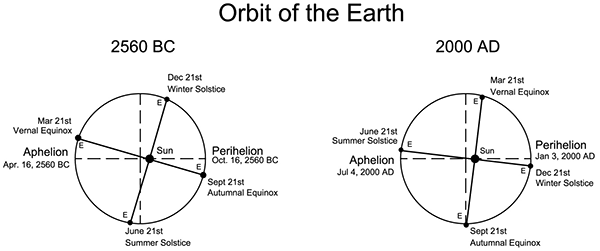Fig. 2 (the eccentricity of the orbit and location of the Sun is not to scale)

In order to calculate the length of a Solar Year from one Winter Solstice to the next one needs to calculate the Precession of the Earth’s Axis relative to a Sidereal Year. Firstly, one needs to allow for the change in rotation rate of the Earth due to Tidal Friction that was stated earlier as 2.3 Milliseconds per Day per Century. As stated earlier this accounts for 38.3 seconds or .000443 days. Additionally we must take into account that the orbit of the Earth has changed over time by a total of 24.3 Seconds or .000281 days over the last 4560 years as was stated earlier.

Therefore we may add these values to the length of the Sidereal and Mean Tropical Years of the present period as follows:

 Present Sidereal Year Change in Rotation Period Sidereal Year 2560 B.C. 365.256363 days + 365.256363 days = 365.256806 days Present Mean Tropical Year Change in Rotation Period Change in Rotation Period Mean Tropical Year 2560 B.C. 365.242189 days + .000443 days + .000281 days = 365.242913 days

With this known one may use the following equation to determine the difference in time between the Sidereal Year and a Winter Solstice Year in the period of 2560 B.C. :Therefore we arrive at a value of 365.242822 days for the duration of a Winter Solstice year in approximately 2560 B.C. (the period when the Great Pyramid was built). This figure may be rounded off to 365.243 days as the difference amounts to 13 Seconds. It is within reason that, over the course of a few hundred years of exact study, the length of the Winter Solstice Year may have been determined to this accuracy in using Sunrise measurements in a Solar Temple such as exists at Luxor.

In view of the importance of the Winter Solstice to the Ancient Egyptians it is certainly within reason to suggest that the length of one Solar Year from one Winter Solstice to the next Winter Solstice may have been incorporated as a measure for the Base Perimeter of the Great Pyramid. In theory 365.243 days may have been multiplied by 100 to arrive at 36,524.3 Pyramid Inches for the Base Perimeter.

If the Base perimeter of the Great Pyramid was by design meant to represent 36,524.3 Pyramid Inches then multiplied by 1.0011 this would be equal to 36,564.477 British Inches. This value multiplied by 2.53999779 would equal 92,873.690 cm or .92873690 km. By multiplying this measure by 43,200 the product is 40,121.434 km. We shall see the importance of this distance a little further on in this paper. The number 43,200 has been suggested in various sources as the factor by which one may multiply the size of the Pyramid to arrive at the size of the Earth to within a reasonable approximation, however, it shall be seen that the use of this number was purposefully chosen to convey highly accurate knowledge of the Earth. It should be noted that 43,200 x 2 = 86,400 which is the number of seconds in one Solar day.

Within the architecture of the Great Pyramid, Davidson observed that the average slope of the Ascending Passage, Entrance Passage and Grand Gallery Floors a ratio of rise to slope is evident as follows: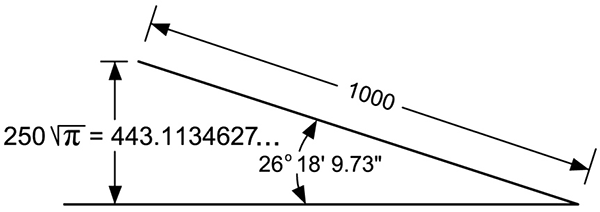Fig. 3(19)

The number 443.1134627 is the product of the equation 250 √π ( π=3.141592654...). As noted by Davidson, this number is used in the scaling of the Royal Cubit to the size of the Great Pyramid Base Perimeter. With a refined estimate of 365.243 days to a Winter Solstice Year at the time of construction of the Great Pyramid we can multiply this number by 100 to arrive at the Base Perimeter of the Great Pyramid in Pyramid Inches. If you divide 36524.3 by 4 the ratio is 9131.075 Pyramid Inches, which represents one base side of the Great Pyramid. If one divides 9131.075 by 443.1134627 the ratio is 20.6066296 PI ” (Pyramid inches). By multiplying 20.6066296 by 1.0011 this equals 20.6292969 Imperial British Inches for the length of one Royal Cubit which clearly compliments Sir Isaac Newton’s figure of 20.63 British Inches and is well within the range of Professor Petrie’s estimate of 20.632 + - .004 British Inches. Furthermore, multiplying 20.6292969 by 2.53999779 the product is 52.3983685 cm. The dimension of 20.6066 Pyramid Inches has been cited in various sources as the most likely dimension of the Royal Cubit (20). It differs from Prof. Petrie’s mean determination by a mere 0.0027 British inches or 0.0685 mm. which falls well within his tolerances.

In the matter of the height of the Great Pyramid it was determined by Professor Piazzi Smyth that the relationship was one in which the Base Perimeter divided by 2π would yield the original design height whereby 36,524.3 / 2π = 5813.023 PI. By measuring the slope angle of casing stones which are still in place he determined that the original slope angle was 51 deg 51' 14.3" (21a). This is precisely the angle, namely the arctangent of 4/π, required for this relationship to hold. In this geometrical relationship the Base Perimeter is equal to the Circumference of a Circle drawn with the height taken as the radius of the Circle. It should be noted that Professor Flinders Petrie, in measuring the slope of the faces of the Pyramid determined that the mean slope angle is 51 Degrees, 52 Minutes +- 2 Minutes (21b). Therefore, to verify the intended slope angle one needs to closely examine other features of the Pyramid which may clarify this point. In the case of a true Pi relationship, attention is drawn to the Royal Cubit dimension and why this particular measure was adopted. If one draws a Pyramidion with the base side of 1 RC ( Royal Cubit ) = 20.6066296 PI = 52.3983685 cm) and a height of 4 RC/2π then the height would be 13.1185878 PI or 33.357837 cm.

## 08The Pyramidion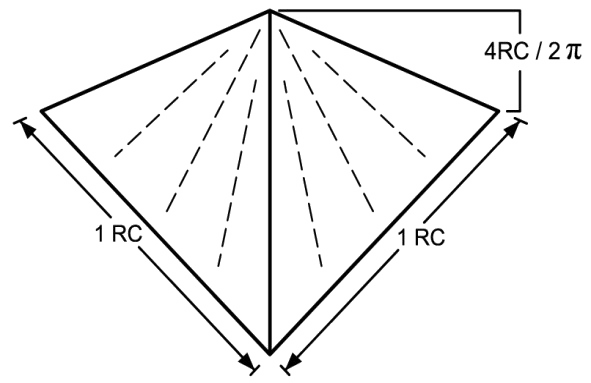Fig. 4

It is of interest to note that the scale of the Pyramidion to the size of the Great Pyramid would be as follows:

 For a Base Side: For the Square Base: For the Volume: 250 √π : 1 (250 √π)2 : 1 (250 √π)3 : 1

At this point we may take a moment to recognize the importance of the value of the square root of Pi (√π).

Throughout History there has been an interest in the mathematical conundrum of "squaring the circle". This is to say, to find an equivalency of the area of a circle to that of a square and vice versa. As we shall see, the link between this equivalency is in fact the square root of Pi (√π).

If we draw a circle with a radius of 1 unit, and since its area is equal to (πr2 ), its area would be equal to :

π 12 = π = 3.1415926...

If we draw a square with each side equal to √π then its area would be simply:

(√π)2 = π = 3.1415926...

To reverse this relationship, let us assume a square with a side length of one unit, whereby its area would simply be:

12 = 1

A circle with equal area would therefore have a radius of 1/√π as may be appreciated in the equation as follows:

π(1/√π)2 =1

It is therefore given that the equivalency of the area of a circle and a given square is derived using the value of the square root of Pi (√π)

It should be understood however, that this is a theoretical equivalency since Pi is an Irrational Number and cannot be written as the ratio of two integers. This was proven by Johann H. Lambert in 1761. (22)

Furthermore, it is important to note that Pi (π) is transcendental whereby there is no polynomial with rational coefficients of which Pi is a root. This was proven by Ferdinand von Lindemann in 1882. (23) The consequence of this is that Pi is not constructible, which is to say that one cannot “construct” a circle equal in area to a square using a compass and straight edge.

Therefore the theoretical equivalence is not constructible. The allure of the Square Root of Pi for the builders of the Great Pyramid, it appears, was simply that they reached the limit of the possible. A further study into the Symmetry of the Great Pyramid and Pi Symmetry in general is explored as follows:

## 09The Symmetry Of The Great Pyramid

The Great Pyramid is proposed to be in a Pi (π) Symmetry and in a square root of Pi (√π) relationship to the Pyramidion as is seen in the scaling of the Pyramidion to the Pyramid. It is therefore of interest to enquire into Pi (π) symmetry in general. It is herewith proposed that the height of the Pyramid was intended to be 2 times the base length divided by Pi (π). This is the same as stating that the Height is equal to the Base Perimeter divided by 2 Pi (π). The following diagram shows a triangle drawn in a Pi (π) Symmetry whereby AD = 2BC / π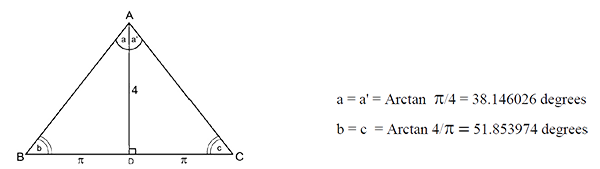Fig. 5

The following is a Pi Symmetry Triangle shown with a Nine-Point Circle and a Circum-Circle: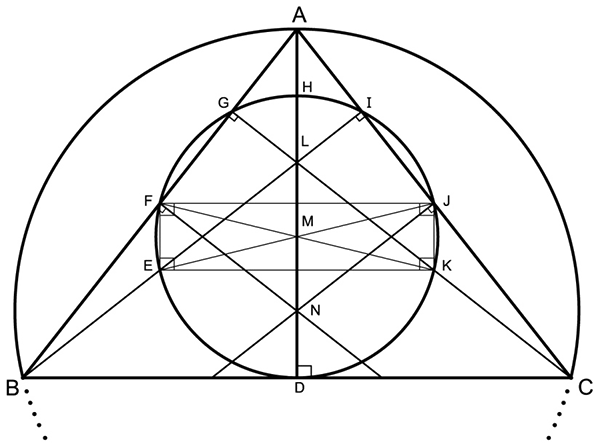Fig. 6

This triangle, like all triangles, has a Nine-Point Circle whereby the points of intersection are D,E,F,G,H,I,J and K. (Point D serves 2 functions; It is the point of intersection of the line AD, which originates from the Vertex A, that is perpendicular to the Base Line BC. It is also the point that bisects the base line BC) Points F and J are the mid points of AB and AC respectively. Points E, H and K are the mid points of the line segments BL, AL and CL respectively where L is the Ortho-centre of the Triangle ABC. (The Ortho-centre is the point of intersection of the lines through the Vertices perpendicular to the opposite sides) Point N is the Circum-centre of the Triangle ABC (the point of intersection of the lines perpendicular to the mid points of the sides of the Triangle)

The distinguishing features of a Pi (π) Symmetry Triangle are as follows:

The Ortho-Centre and the Circum-Centre of the Triangle are equal distant from the Centre of the Nine-Point Circle at Point M in the diagram. (Points L and N are equal Distant to M)

The distance AH, HL and ND are equivalent. ( The distance from the Base line BC to the Circum-Centre N is equivalent to the Midpoint of the distance from the Vertex A to the Ortho-centre L). These distances are all in a π relationship to the height of the Triangle namely: AH = HL = ND = AD((1-(π/4)2) / 2)

The Ortho-Centre is in a Pi (π) relationship to the height of the Triangle namely: LD = AD ( π/4)2

The Diagonals EJ and FK of the Rectangle EFJK intersect precisely in the Centre of the Circle at M. The Diagonals are Diameters of the Circle.

The Nine-Point Circle is Tangent to the Base Line BC.

The Circum-Circle (The Circle intersecting at A, B and C) is precisely twice the Diameter of the Nine-Point Circle. The 9-Point Circle Diameter is in a Pi (π) relationship to the Triangle height, namely:

In addition to the Symmetry of the Nine-Point Circle we may investigate the Symmetry of an Inscribed Circle as per the following: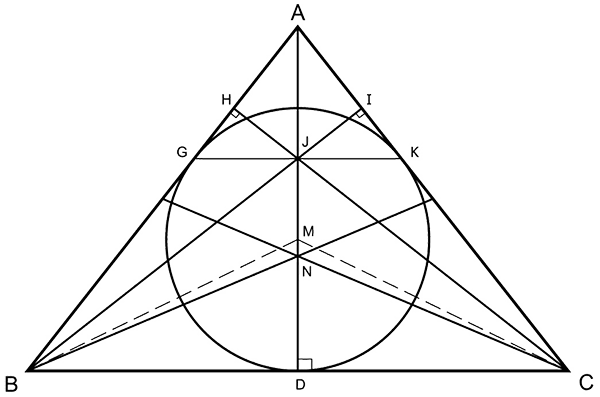Fig. 7

The distinguishing feature of the Inscribed Circle of a Pi (π) Symmetry Triangle is that the Circle intersects the sides of the Triangle precisely at the elevation of the Ortho-Centre, namely the points G and K are the end points on the line GK which is parallel to the Base Line BC and passes through the Ortho-Centre point J. As noted earlier, the Ortho-Centre is itself in a Pi (π) relationship with the height of the Triangle whereby JD = AD (π /4 )2.

Point M is the Centre of the Inscribed Circle and it is determined by drawing lines which bisect the angles of the Vertices A,B and C respectively.

In the case of an Isosceles Triangle, such as this one, the Centroid of the Triangle, which is Point N in this drawing, is located at 1/3 of the height of the Triangle. This point is the Centre of Mass.

Another distinguishing feature in comparing the Inscribed Circle, Nine-point Circle and Circum-Circle is as follows: The Ratio between the Inscribed Circle's Circumference and the Circum-Circle's Circumference is precisely twice that of the Ratio between the Inscribed Circle's Circumference and that of the Nine-Point Circle's Circumference. This is a note-worthy point.

It is to be appreciated that these distinguishing features of a Nine Point Circle, Circum-Circle and the Inscribed Circle in relationship to a Pi (π) Symmetry Triangle may very well have been instrumental in the choice of this particular shape of the Great Pyramid by the Architect. It should be noted however, that this is not constructible since the value of Pi (π) is an irrational number and cannot be written as a ratio of two integers. Furthermore, it is transcendental, whereby there is no polynomial with rational coefficients of which Pi is a root. The nature of a Pi (π) Symmetry Triangle is that it may be regarded as a theoretical ideal which remains elusive as it may not be constructed using compass and ruler.

The following Diagrams show the relationship of a Pi (π) Symmetry Triangle with Circles and Squares which are also noteworthy: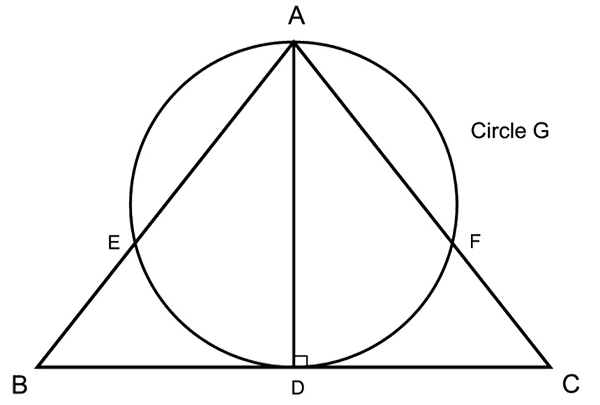Fig. 8

The Fig. 8 shows a circle which has a diameter equal to the height of the Pi (π) Symmetry Triangle and it is noteworthy to find that the area coverage of the Circle and the Pi Triangle is identical.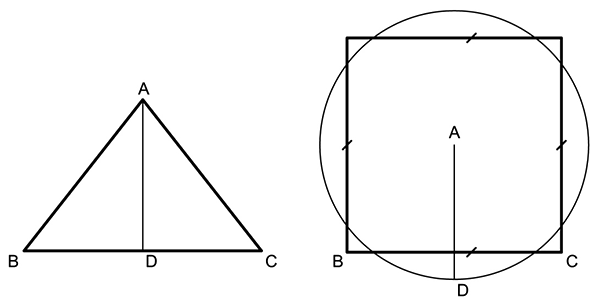Fig. 9

Fig. 9 shows a Circle with a Radius equal to the Height of the Triangle and a Square which has sides equal to the Base of the Pi Triangle. The Circumference of the Circle and the Perimeter of the Square has an equivalent measure. This "Squaring of the Circle" could very well have been the principal consideration in the shape of the Great Pyramid. This relationship was explored by Professor Piazzi Smyth in his book " Our Inheritance in the Great Pyramid".

Further points of considerable interest are to be found in the relationship of 3-dimensional Pi (π) Symmetry.

Consideration may be given the following:

1. A square based Pyramid that has, as its height, the relationship of 4 times its base side divided by 2π as is the case with the Great Pyramid and the scaled down Pyramidion.
2. Secondly, a Sphere that has, as its diameter, a measure equal to the height of such a Pyramid.
3. Thirdly, a Cube that has each side equal to a base side of such a Pyramid.

The following mathematical relationships are evident:

The Volume of the Pyramid multiplied by 1 1/2π is equal to the Volume of the Cube.

The Volume of the Sphere multiplied by 1/2π is equal to the Volume of the Pyramid.

The Volume of the Sphere multiplied by 3/4π2 is equal to the Volume of the Cube.

The Surface Area of the Sphere multiplied by 1 1/2π is equal to the Surface Area of the Cube.

As may be appreciated, Pi (π) Symmetry Pyramids, and there from derived Cubes and Spheres are all relational through the value of Pi (π)

This should not come as a surprise, however, consideration of this fact further demonstrates that the builders of the Great Pyramid would, undoubtedly, have been influenced by this mathematical relationship in the choice of its shape.

Clearly, the ratio of the circumference of a circle to its diameter is multifaceted in two and three dimensional shapes as may be appreciated in recognizing the symmetries explored in this chapter. This nature of Pi (π) is remarkable.

## 10Internal Geometric Square Circuit

In addition to the External Geometric Circuit (Perimeter) of the Great Pyramid which is given as 36,524.3 Pyramid Inches, there is an Internal Geometric Circuit which was measured by Professor Petrie in the late 1800s. Since most of the outer cladding of the Pyramid had been removed, the shape of the Pyramid was extrapolated based on some remaining cladding. The measurements, given in British Inches, as stated in his book “The Temples and Pyramids of Gizeh” are as follows :

 North Side East Side South Side West Side Mean length 9069.4 9067.7 9069.5 9068.6 9068.8

His measurements are given to an accuracy of + - .5 Inches (24). It should be given consideration that the North Side is where the Entrance Passage is located and it is in this way the most important side of the Pyramid. Its length therefore would have been most important during the construction and the other lengths would have been secondary in relevance. If one adds the margin of error in measurements (.5 Inches) to the mean value of 9068.8 one arrives at the value of 9069.3. Furthermore, Professor Petrie used the North Side of the Pyramid as the basis point from which to measure the other lengths therefore the error in measurement was least in regard to the North Side. On this basis one could say that the measurement of the North side most closely reflected the intended size. Therefore, the measure of 9069.4 B” or 23,036.256 cm may suggest the intended base length of each side. The base length multiplied by 4 would give a base perimeter of 921.45 meters. In 1925 Ludwig Borchardt, Director of the German Institute of Archeology in Cairo commissioned J. H. Cole to determine the exact dimensions of the Great Pyramid. His survey concluded the measurements of the base side lengths of the Pyramid given in meters as follows: (given on a drawing prepared for Ludwig Borchardt.)(25)

 North Side East Side South Side West Side Total Perimeter 230.253 230.391 230.454 230.357 921.455

In adding these lengths one finds a Perimeter of 921.455 meters which is longer than Prof. Petrie’s measurement of the North side multiplied by 4 by a mere .005 meters or 0.5 cm. In taking an arithmetic mean between the value derived from Professor Petrie’s survey and that as recorded by Ludwig Borchardt one finds a value of 921.4525 meters. If one multiplies this measure by 43,200 one arrives at a value of 39,806.748 km. The radius of a circle with this circumference would be 6335.4407 km. The Radius of Curvature in a Meridian plane at the Equator as calculated from WGS 84 data is 6335.4393 km (26). The difference in these two values is .0014 km or 1.4 meters in radius.

Evidently, the internal square circuit of the Great Pyramid represented the Meridional Radius of Curvature of the Earth at the Equator to an extremely high degree of accuracy. It has been stated in various sources that the perimeter of this square circuit multiplied by 2 is equal to 1 Minute of Latitude at the Equator.

## 11Relationship of Inner Square Circuit and Outer Square Circuit

The relationship between the Inner square circuit and the original Outer square circuit is proposed to be as follows: D. Davidson suggested, in his book “Great Pyramid, Its Divine Message”, that the casing stones (cladding) were originally as thick as the hollowing in of the sides of the Pyramid which he stated was 35.76 PI ”and that this added to each of the corners would add a “Displacement Factor” of 286.1 PI” to the overall Perimeter.(27) He went on to state that this factor was also given in the measurement by which the Entrance Passage is offset from the Mid-line of the North Side of the Pyramid. Professor Petrie measured this offset as follows :

The Entrance Passage is offset from the Midline of the Pyramid at the Entrance opening by 287. + - .8 B” or 286.7 PI” . He goes on to state that the Offset at the end of the Passage is 286.4 + - 1.0 B” or 286.1 PI”. Therefore, a simple average would be appropriate; ( 287 + 286.4) / 2 gives a value of 286.7 B” which is equivalent to 286.4 PI “. The measurement of the Internal Geometric Circuit as stated above was found to be 921.455 meters which equals 36,237.9 PI”. If one adds 286.4 PI" to the value 36,237.9 PI” the sum is 36,524.3 PI” . Clearly, it can be maintained that the External Circuit of the Pyramid could have been by design 36,524.3 PI” as has been earlier proposed based on the number of days in a Winter Solstice Year estimated for a period 4560 years ago. The thickness of the casing stones would have been 286.4/8 =35.8 PI” or 35.84 B” which equals 91 cm.

The Pyramid Inch was, it seems evident, determined as of a certain distance to incorporate the right number in relation to the days in a Winter Solstice Year. In other words, the precise measure of the Pyramid Inch was determined after the appropriate dimension of the Pyramid was set out.

The following drawing shows the geometry of the Inner Square Circuit and the Outer Square Circuit: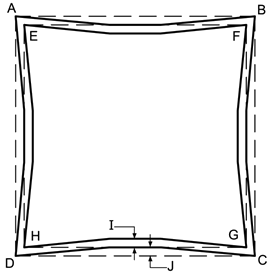Fig. 10 (hollowing of sides and distances are not shown to scale)

ABCD = 928.7369 Meters
ABCD = 36524.3 Pyramid Inches

EFGH = 921.455 Meters
EFGH = 36237.9 Pyramid Inches

I = 35.8 Pyramid Inches
J = 35.8 Pyramid Inches

It should be noted that Professor Petrie calculated that the original height of the Greet Pyramid was 5776. + - 7. British Inches. This height would apply to the Inner Pyramid casing. If one allows for an outer casing of a thickness of 35.8 Pyramid Inches ( 35.84 British Inches ) then the original height of the external Pyramid of 5813 Pyramid Inches would be achieved well within reasonable tolerances.

## 12Reference Circumference

The measurement of 40,121.434 km (the External Base Perimeter of the Great Pyramid multiplied by 43,200) is of particular interest as it relates to the shape of the Earth. The Earth has a Transverse Radius of Curvature at the Equator of 6378.137 km (which is identical to the radius) and a Polar Transverse Radius of Curvature of 6399.5936 km which is identical to the Meridional Radius of Curvature at the poles. It should be noted that the Polar radius is 6356.7523 km as per WGS 84 Data. The reason that the Radius of Curvature at the Poles is larger than the actual radius is that the Earth is an Oblate Spheroid. The Meridional Radius of Curvature at the Equator is given by the equation:

b2 /a = Polar Radius2 /Equatorial Radius = 6356.752322 / 6378.137 = 6335.4393 km

The Meridional and Transverse Radius of Curvature at the poles is given as follows:

a2/b = Equatorial Radius2 / Polar Radius = 6378.13722 / 6356.7523 = 6399.5936 km

To calculate the Meridional Radius of Curvature as a function of Geodetic Latitude one can use the following equation: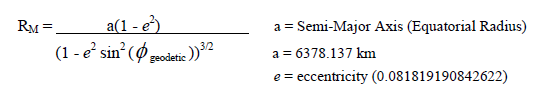To calculate the Meridional Radius of Curvature as a function of Geodetic Latitude one can use the following equation: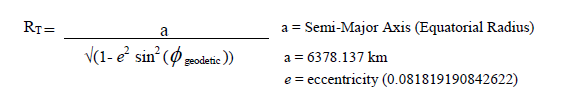The following diagram illustrates an example of a Transverse Osculating Circle:Fig. 11

The Geodetic Latitude at which the Earth has an Osculating Circle of 40,121.434 km which yields a Transverse Radius of Curvature of 6385.5245 km occurs at 35.9955 degrees Geodetic Latitude. As noted earlier the value of 40,121.434 km is given from the Great Pyramid’s perimeter measure multiplied by 43,200. It is of considerable interest to note that at precisely 36 degrees Geodetic Latitude the Transverse Osculating Circle of the Earth is 40,121.441 km which constitutes a difference of .007 km or 7 meters. In terms of Transverse Radius of Curvature, this constitutes a difference of 1.12 meters.

It should be given due consideration that the Cosine of 36 degrees is precisely
½ Phi = ½ Φ = ½ x ((1+ √5)/2) = ½ x 1.618033989 = .809016994 .

Also to be given consideration is that if one multiplies the Transverse Radius of Curvature of any sphere at 36 degrees Geodetic Latitude by ½ Phi (½ Φ) one arrives at the Lateral Radius of that sphere at that Geodetic Latitude. For instance, at precisely 36 degrees Geodetic Latitude the Earth has a Transverse Radius of Curvature of 6385.525666 km.

Multiply 6385.525666 x ½ Φ = 5,165.99878 km.

Note that 5,165.99878 x 2π = 32,458.92765 km which is precisely the Lateral Circumference of the Earth at 36 degrees Geodetic Latitude.

In the case of the measurement as given by the Great Pyramid: 6,385.5245 x ½ Φ one arrives at a value of 5,165.9978 km and multiplied by 2π this equals 32,458.92147 km in circumference. This constitutes a difference of 6.2 meters in circumference or 0.98 meters in radius.

It is quite clearly evident that the builders of the Great Pyramid intentionally chose a perimeter measurement that would relate to 36 degrees Geodetic Latitude and in so doing expressed, not only a highly precise knowledge of the Earth’s size and shape, but also demonstrated a precise

knowledge of the value of the Golden Ratio or Phi (Φ) the value of Pi (π) and their relationship to a spherical body.

As we have seen, the Transverse Osculating Circle of the Earth at 36 degrees Geodetic Latitude is 40,121.434 km which is equal to the Base Circumference of the Pyramid multiplied by 43,200 to within an extremely accurate tolerance.

As the Base Circumference is equal to 1000 vπ Royal Cubits, then the following equation is evident:

It is clearly evident that the Royal Cubit is derived from the shape of the Earth at 36 degrees Geodetic Latitude and which involves the value of the Square Root of Pi (vπ)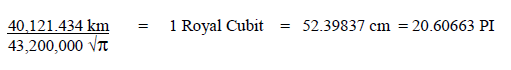## 13The Golden Ratio

The Golden Ratio ( Phi ) is believed to have been used in architecture and art since at least the Renaissance. We have seen however that the Golden Ratio was used in determining the perimeter of the Great Pyramid in its relationship to the shape of the Earth given that it relates itself to 36 degrees Geodetic Latitude , and furthermore, we shall see that the values of Φ , √ Φ , and 1/Φ are discernable in the construction measurements of the 35th Course of Masonry.

The Golden Ratio in geometry is given, by example, in the division of a line whereby the ratio of the long segment is to the total length as the short segment is to the long segment. Algebraically this may be expressed as follows:

 a + b = a    a       b Where a = long segment of line      b = short segment of line

An equation that solves for the Golden Ratio is as follows:

 a = ( 1 + √5 ) = 1.618033989... b         2

Furthermore the Golden Ratio may be expressed in a relationship geometrically as follows:
Golden Ratio = Φ

 Φ = 2 x cosine 36 degrees Φ = 1.618033989...

In nature one finds the Golden Ratio in plant growth patterns, in the shape of Nautilus shells and in the shape of Spiral Galaxies for example.

The external measurements of the Great Pyramid indicate quite clearly that the Golden Ratio was in fact known.

## 14The Symmetry of the Giza Plateau

On the Giza plateau there are three principal Pyramids; The Great Pyramid of Khufu, also known as Cheops, The Pyramid of Khafre (The Son of Khufu) and the Pyramid of Menkaure (The Grand-son of Khufu). In addition to these three principal pyramids there are 3 relatively small pyramids to the East of The Great Pyramid of Khufu as well as 3 small pyramids to the south of The Pyramid of Menkaure. These pyramids have been named "Pyramids of Queen's". Additionally, there is a small Subsidiary Pyramid to the south of The Pyramid of Khafre.

The Sphinx is located East of the Pyramid of Khafre, slightly to the north of the East End of a Causeway originating at the Mortuary Temple of Khafre which is, itself, located at the East Side base of The Pyramid of Khafre. To the South of the Sphinx is the Tomb of Queen of Khafre and to the East lies the Sphinx Temple and South-East, The Valley Temple of Khafre.

Other features of the Giza Plateau are a Mortuary Temple at the East Side Base of the Pyramid of Menkaure wherefrom another Causeway leads to the Valley Temple of Menkaure.Fig. 11 A

At this point, we may investigate the relationship of the placement of the Giza Pyramids from a purely mathematical perspective.

It has been determined by others, from Satellite photos and mapping that a circle with a diameter measurement of exactly 2400 Royal Cubits and a therein inscribed square encompasses all the principle structures on the Giza Plateau and this can readily be seen in studying the drawing below.Fig. 11 B

Note that the North East corner of the Great Pyramid of Khufu intersects the 2400 Royal Cubit diameter Circle at point A on the drawing. Furthermore, The North-East Corner of the northern most Pyramids of Queens of the Great Pyramid of Khufu intersects the circle at point B. Also, we may appreciate that the South West corner of the western-most Pyramids of Queens of The Pyramid of Menkaure intersects this circle at point E on the drawing. These points all intersect the Circumference of a Circle which has a Diameter of exactly 2400 Royal Cubits. Very significantly, it is also found that the Sphinx itself lies on the circumference of this 2400 Royal Cubit diameter circle.

Furthermore, we see that the southern edge of The Pyramid of Menkaure sits on the southern boundary of the inscribed square that is formed within the 2400 Royal Cubit Diameter Circle.

Further on in this book, it is substantiated that the number 2400 is encoded in the internal dimensions of the Queen’s Chamber, and it is contended that this "Code Number" unlocks the mystery of the dimensions of the Great Pyramid's Passageways and Grand Gallery. In a sound mathematical logic, the derived measurements of passageways indicate astonishing knowledge of the Earth's shape and size as well as Astronomical and Astrophysical knowledge possessed by the builders at the time of construction. Please refer to the Chapter Queen's Chamber Code Number.

To find that the number 2400 is used in the boundary of the organization of the entire Giza Plateau's components is of the greatest significance and substantially signifies an over-arching intelligent design intended by the builders to convey information pertaining to the purpose of the entire edifice.

In addition to what others have found, a further significant point is that that the distance between the Centre of the Great Pyramid of Khufu (Point H) and the Centre of the Pyramid of Menkaure (Point G) is precisely 1800 Royal Cubits.

When one multiplies 2400 by 1800 the product is 4,320,000 and divided by 100 this equals 43,200.

As we have seen, the scaling of the Great Pyramid of Giza to the Earth is 43,200:1

We have also seen that the perimeter of the Great Pyramid, with a measure of 36,524.3 Pyramid Inches, denotes the number of days in a winter Solstice Year in 2560 BC multiplied by 100 and we can then appreciate the factor of 100 used in relationship to the Giza Plateau in general.

Also, the number 2400 could be divided by 100 to arrive at 24 which is the number of hours in a day. As we may appreciate, The Great Pyramid relates to the Hemi-sphere of the Earth and, clearly, 43,200 x 2 = 86,400 which is the number of Seconds in one day.

In addition to what others have earlier found, we may now go on to examine significant angles by which the principal pyramids are arranged within the 2400 Royal Cubit diameter Circle.

Of great interest is to study the North-South Axis of The Great Pyramid of Khufu, which is Line HO in the drawing, and the East-West Axis of the Pyramid of Khafre which is Line PC in the drawing. Specifically, our attention should be drawn to Point C, which is the end point of the East west Axis of the Pyramid of Khafre at the Eastern Intersection with the 2400 Royal cubit Diameter Circle that incorporates the Giza Pyramids. Point O is the Southern point of Intersection of the North-South Axis of The Great Pyramid of Khufu and the square which is inscribed in the 2400 Royal Cubit Diameter Circle and which, furthermore, defines the southern edge of the Pyramid of Menkaure. We will note that a line drawn from Point O to point C, defined as angle HOC, has an angle of 36 degrees. We will also see that the head of the Sphinx (Point D) is the end point of a line that is 36 degrees from the North-South Axis of the Great Pyramid of Giza as given in Angle OHD.

As we have seen, the Perimeter of the Great Pyramid of Giza multiplied by 43,200 defines the Transverse Osculating Circle of the Earth at 36 Degrees Geodetic Latitude. As we have seen, the Cosine of 36 Degrees is equal to 1/2 Phi (1/2 The Golden Ratio of 1.618033989...= .8090169945...)

It is therefore of great significance to find that the angle of 36 degrees is found as a defining measure of the placement of the Great Pyramid of Giza on the Plateau in relationship with the Pyramid of Khafre and the alignment of the Head of the Sphinx with the North South alignment of the Great Pyramid of Khufu.

As we continue to investigate the placement of the Principle pyramids on the Giza Plateau, we find evidence that undoubtedly supports the contention that the design criteria of the Great Pyramid of Giza encompasses Pi (π) Symmetry. Upon careful examination of the Site Plan Drawing of the Giza Pyramids, we find that the East-West Axis of the Pyramid of Khafre, indicated as PC on the drawing, intersects with the North-South Axis of the Great Pyramid of Khufu indicated as HO on the drawing at Point M.

Special attention should be given to the Angle JMK. One will note that the angle JMK is 51.854 Degrees. This angle is derived from lines originating from the South-West and South-East corners of the Great Pyramid of Khufu, which are Points J and K respectively, drawn to intersect the East West Axis of the Pyramid of Khafre at M. Furthermore, we find that the centre of the Pyramid of Khafre at P, is placed at a 51.854 degree angle with the North-South Axis of The Great Pyramid of Khufu at Point O, (the Point of Intersection with the Inscribed Square in the 2400 Royal Cubit Diameter Circle), as in Angle POH in the drawing.

The reason that this angle is particularly important is that the Arc-Tangent of 4/π. is 51.854...Degrees. This angle is identical to the Slope Angle of the Sides of the Great Pyramid of Khufu as seen in the earlier chapters and signifies Pi (π) Symmetry.

It is, therefore, found that two of the three principal pyramids on the Giza Plateau, in their placement, signify a Pi (π) Symmetry. This is a significant finding and supports, undoubtedly, intent on the part of the builders that cannot be discounted as arbitrary and, rather, fully corroborates the other findings. It, undoubtedly, supports the contention that the Giza Plateau is a thoroughly intelligent and integrated design.

The next item of interest pertains to the nature of Pi (π) Symmetry and specifically the relationship of a Circle that has the same measure in its Circumference as the measure of the Perimeter of a Square as we have seen in the earlier chapter "The Symmetry of the Great Pyramid" on Page 12.Fig. 11 C

Once a Square is inscribed within Circle # 1, which has a diameter of 2400 Royal Cubits, the next step is to draw a complimentary second circle. Circle # 2, which has a Circumference theoretically equal in length to the perimeter of the Inscribed square of Circle # 1, itself has a diameter of 2160.76 Royal Cubits.

Although there is a lack of documented survey evidence, which is due in part to the present state of the Pyramids of Queens, we should take note that from Satellite Photos and numerous drawings of the Giza Plateau, this circle does certainly appear to intersect with the South-West corner of the Northern-most pyramid of the Pyramids of Queens of The Great Pyramid of Khufu at Point C as well as the South-East corner of the Southern-most pyramid of the Pyramids of Queens of The Great Pyramid at Point D. We also find that Circle # 2 appears to intersect the North-East corner of the middle Pyramid of Queens of The Pyramid of Menkaure at Point G as well as the South-East Corner of the Eastern-most Pyramid of Queens at Point H.

Circle # 2, therefore, appears to intersect at 4 distinct points on the Giza Plateau and this certainly does imply a design intention that compliments other findings. The idea of a Circle with equal measure to that of a square has been the subject of much interest in ancient times and to find it here on the Giza Plateau is certainly very plausible and compliments the findings concerning the symmetry of the Great Pyramid itself.

At this point, we may now focus our attention on Circle # 3 which has its centre at Point M which is the centre of the Subsidiary Pyramid that lies just South of the Pyramid of Khafre. This pyramid appears, at first glance, to be insignificant, however, we shall find that its choice of placement on the Giza Plateau certainly signifies a mathematical logic that supports the design criteria of the entire complex and, most significantly, that of the Great Pyramid of Khufu particularly.

We will note that this circle intersects the South-West corner of the Great Pyramid of Khufu at Point J in the drawing. We should take note that a line drawn from the centre of the Subsidiary Pyramid of Khafre at Point M to point J is precisely 60 degrees, as in Angle RMJ. It is very important to note that 60 Degrees is also denoted as 1.04719755... Radians, which is precisely Pi divided by 3 (π/3) Radians.Fig. 11 D

A right angle triangle with 60 degrees has the following values: Sine = √3/2, Cosine = √1/2 and Tangent = √3. It is defined as one of the Moebius Triangle, meaning that it tessellates the plane via reflections in its sides. In all, there are 3 such triangles. The other 2 Moebius Triangles are 60-60-60 and 45-45-90 Angle triangles.

Furthermore, if this line, originating from M, is projected through Point J, the Great Pyramid of Khufu's South-West Corner, it ends at Point N. Point N is notable for it is a point at which the Northern base line of the Great Pyramid of Khufu intersects with Circle # 2. As we will recall, Circle # 2 has an identical Circumference to the 2400 Royal Cubit Diameter Circle's (Circle # 1) Inscribed Square's perimeter. This supports the contention that Circle # 2 is relevant to the layout design of the Plateau Structures. We should also take careful consideration that a line drawn from Point M to Point P, which is the South-West corner of the Middle Pyramid of Queens of The Great Pyramid of Khufu is exactly 36 Degrees as in Angle RMP. As we have seen earlier, 36 degrees is particularly important in Phi, (Φ) Symmetry since the Cosine of 36 Degrees is 1/2 Phi. Here again, the significance of 36 degrees supports the basis of the Great Pyramids Perimeter dimension.

Furthermore, we see that Circle # 3 intersects the North-South Axis (Line SK) of the Great Pyramid of Khufu at Point K which is co-existent the point of intersection with the Inscribed Square of Circle # 1 which has a 2400 Royal Cubit Diameter. Furthermore, we may appreciate that this Circle goes on to intersect the South-East corner of the middle Pyramid of Queens of the Pyramid of Menkaure at Point F in the drawing.

Certainly, at this point, we may be cognizant and appreciative that the sizes and placements of each of the Pyramids of Queens were most apparently chosen so that they fulfill the function of having points of intersections with Circles or provide angles of lines as part of a fully integrated design imperative that highlights Pi and Phi Symmetry and two of these intersections define the boundary of the 2400 Royal Cubit diameter circle.

We may now go on to see a further most interesting aspect as found in the Circle # 3's dimension and relationship with Circle # 2.

Circle # 2 has a calculated diameter of 2160.76 Royal Cubits and Circle # 3 has a measured diameter of 1698.75 Royal Cubits based on Satellite photos and site plans drawn of the Giza Plateau Pyramids.

Note that 2160.76 / 1698.75 = 1.272

It should be noted that the Square Root of Phi, the Golden Ratio, is equal to 1.2720196496... as in: √Φ = √1.618033989... = 1.2720196496...

The square root of Phi is a very important mathematical value which is to be found readily in the natural world in plant growth patterns and, notably, in cosmological order on a grand scale. This proportion was used in Greek architecture in ancient times and is found in numerous artwork throughout the ages. We shall see that it was used in the 35th Course of Masonry of the Great Pyramid itself in a later chapter.

At this point we might take note, that, the principal 3 pyramids are different in size from one another. The possible reasoning of this is herein explored. The Pyramid of Khafre is only slightly smaller than The Pyramid of Khufu, whereas, The Pyramid of Menkaure is substantially smaller than either. This difference in sizes begs the question as to why they are different and, more specifically, by what measure.

The Great Pyramid of Khufu is subscribed to have been 443.113 Royal Cubits on each Base Side which is equal to 250 √π Royal Cubits. This measurement is based on a calculation that includes Casing Stones which formerly encased the Pyramid. The total Perimeter of the Great Pyramid is found to have had a theoretical measure of 36,524.3 Pyramid Inches denoting the number of days in a Winter Solstice Year in the Year 2560 BC multiplied by 100 Inches to the day.

It is herein maintained that the Pyramid Inch was devised after the intended size of the Pyramid was laid out and not a-priori. The Base Area Coverage of the Great Pyramid of Khufu would have been, theoretically, 443.1132 Royal Cubits= 196,349.13 Royal Cubits2

In review of Professor Petrie's measurements, as documented in his book "The Pyramids and Temples of Gizeh, 1883", we find that he determined that the mean length of a base side of the Pyramid of Khafre is 8474.9 British Inches. This would equate to 8465.6 Pyramid Inches.

If one divides by 20.60663 (Pyramid Inches per Royal Cubit) we find that the Base Side is equal to 410.82 Royal Cubits. The Base Area Coverage of the Pyramid of Khafre would therefore be 410.822 Royal Cubits = 168,773.07 Royal Cubits2

Furthermore, the Pyramid of Menkaure was measured by Professor Petrie and documented in his book " The Pyramids and Temples of Gizeh, (1883) in which he stated that the Mean Base Side measured 4153.6 British Inches, which would equate to 4149.0 Pyramid Inches.

This would be equal to 201.343 Royal Cubits. The Area Coverage of the Pyramid of Menkaure would be 201.3432 Royal Cubits = 40,539.00 Royal Cubits2

If one adds the Base Area Measure of the Pyramid of Khufu and that of the Base Area Measure of the Pyramid of Khafre, as in 196,349.13 + 168,773.07, this equals 365,122.2 Royal Cubits2

At this point we may compare the relationship of the sum of the area coverage of the Pyramids of Khufu and Khafre with that of Menkaure as in 365,122.2 / 40,539.00 and find that there is a ratio of 9.0067 . For all intents and purposes, this suggests a ratio of 9:1 . We may consider the fact that there are 3 Principal Pyramids, therefore, the idea of dividing 9 by 3 to arrive at the number 3 may be considered as, perhaps an underlying logic. Alternately, one might consider √9 = 3; as this would mathematically "un-square" the Base Areas.

At this point we may consider the ratio between the Base Areas of the Pyramids of Khafre and Khufu as in 168,756.64 / 196,349.13 which equals .85947 . Rounded this equals .86

Note that 1-.86 = .14

If we add these two derived numbers as in 3 + .14 we arrive at 3.14 which is a reasonable approximation of the value of Pi (π).

It is, therefore quite plausible that the reason that the three principal pyramids are of different sizes and in a specific ratio was to indicate the value of Pi and this furthermore supports the contention that the Great Pyramid of Khufu itself was built in Pi (π) (3.14) Symmetry.

Taken in isolation, the above relationship may be deemed tenuous and inconclusive, however, taken together with all the other corroborative findings, it could certainly indicate intent on the part of the builders.

At this point, we shall investigate additional "Angles and Alignments on the Giza Plateau" which are very noteworthy and clearly demonstrate intentional conveyance of intelligent design with mathematical acumen.Fig. 11 E

In careful examination of this drawing, one finds co-existent intersections which indicate a precision in design which is truly magnificent.

As seen in the previous drawing, the North-South Axis of The Great Pyramid of Giza, line SK intersects precisely at the co-incidental intersection point of Circle # 3 and the Inscribed Square of Circle # 1 at Point K.

Note also, that the Line TW which is a vertical line connecting the points of intersection of Circle # 2 and the Inscribed Square abcd of Circle # 1, at Point T, along the Northern Boundary and Point W, along the Southern Boundary, intersects Circle # 3 at Point V.

Point V is co-existent the point of intersection of the line YV which is the East-West Axis of the Pyramid of Menkaure. This co-existent intersection is most remarkable as it defines very accurately the size and placement of the Pyramid of Menkaure.

We should take note that a line originating from the Centre of the Pyramid of Menkaure at Point Y intersects the North-South Axis of The Great Pyramid of Khufu, Line SK, at Point X. The Horizontal line which originates at X is the East West Axis of the Sphinx and in this way defines the placement of the Sphinx on the Giza Plateau. This is a very noteworthy point as the line YX is at an angle of 26.3... Degrees from the East-West Axis of The Pyramid of Menkaure as in Angle VYX.

The importance of this cannot be overstated.Fig. 11 F

Note that the Sine of 26.30270169... Degrees = √π/4 = .4431134627...

Consideration is to be made that one base side of the Great Pyramid is equal to 443.113 Royal Cubits as in 1000 x √π/4 Royal Cubits or 250 √πRoyal Cubits.

As we will see in later chapters, 26.3 Degrees is found in the slope angle of the Descending, Ascending and Grand Gallery floors in the Interior of the Great Pyramid. We will also see that it is instrumental in the slope of a diagonal of the Rectangular facing of the King's Chamber Coffer.

The next point of interest is that the measurement of the Sphinx's Head, Point BB, is from the centre of the Great Pyramid, Point S, precisely 1000 Royal Cubits as evident in the line S- BB.

One should recall that the perimeter of the Great Pyramid is equal to 1000√πRoyal Cubits.

The Angle of this line is, furthermore, exactly 36 Degrees from the North-South Axis of the Great Pyramid which once again supports the Great Pyramid's Base Perimeter as being to scale with the Transverse Osculating Circle of the Earth at 36 Degrees Geodetic Latitude.

We also see that a line drawn at 36 Degrees from Point J, The South-West Corner of the Great Pyramid extends to point CC, between the Paws of The Sphinx.

The Intersection of these two lines, S-BB and J-CC, is at Point AA and a horizontal line from AA to The Pyramid of Khafre Intersects at Point Z. This point is Co-existent the point at which a 36 Degree Line originating from the Centre of the Subsidiary Pyramid of the Pyramid of Khafre, Point M , passes through the Eastern Base line of the Pyramid of Khafre as it extends to Point P, the South-West Corner of the middle Pyramid of Queen's of the Great Pyramid of Khufu.

This drawing very clearly illuminates the complexity and highest intelligence in design that is manifested in the layout of the Giza Plateau. One certainly needs to make very careful examinations to absorb the intricacies involved.

Ultimately, to find the Sphinx to have its placement on the Giza Plateau in accordance to all these co-existent relationships is quite extraordinary and has far reaching import in understanding the high level of intelligence in the design of the entire Giza Complex and in particular, as we shall see in later chapters, the intelligence of the design of the Great Pyramid itself.

In summary, it can be contended that the placement of the Sphinx supports the dimensions of the Great Pyramid in the relationship of the Sine of the Angle of 26.3 Degrees alignment multiplied by 1000.

Furthermore, the angle of 36 Degrees has been instrumental to the placement of the Sphinx in a multitude of relationships and this also supports the Base dimension of the Great Pyramid.

The next item of interest is to examine alignments which establish noteworthy Triangles on the Giza Plateau involving the Pyramid of Queen's of The Great Pyramid of Khufu and those of the Pyramid of Menkaure as may be appreciated in the following drawing:Fig. 11 G

The first angle of interest is 45 Degrees which is evident when a line is drawn from the centre of the Easternmost Pyramid of Queen's of the Pyramid of Menkaure at Point F and ends at the centre of Northernmost Pyramid of Queens of the Great Pyramid of Khufu.

The line DG is a horizontal line and passes through the centres of Pyramids D,E and F and is in effect the East-West Axis of these pyramids. The line AG is effectively the North-South Axis of Pyramids A,B and C. Angle GFA is 45 Degrees.

A right angle triangle with 45 degree Angles is another of the Moebius Triangles, meaning that it tessellates the plane via reflections in its sides. We have already seen that the 30-60-90 Triangle, one of the other Moebius Triangles, was involved in the alignment of the Great Pyramid of Khufu. The 45-45-90 Degree Triangle may also be referred to as the Square Root 2 Triangle.Fig. 11 H

The second Triangle of interest originates from a line drawn starting from the centre of the middle Pyramid of Queen's of the Menkaure Pyramid at Point E and ends at Point B, the centre of the middle Pyramid of Queen's of the Great Pyramid of Khufu.

The angle formed from the base line, DG, is 41.41 Degrees.

A right Angle Triangle with 41.41 Degrees has a very interesting proportionality which we will investigate here to better understand its relevance in relationship to Pi Symmetry.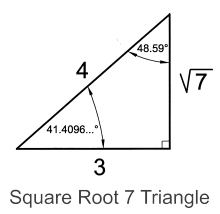Fig. 11 I

An Isosceles Triangle with 41.41 Degrees is as follows:Fig. 11 J

A Square root 7 Triangle may be constructed easily using the proportionality of 4 units for the sides of an Isosceles Triangle and a base of 6 Units as seen in the above drawing.

It is of particular interest to note that the height of the Triangle is the Square Root of 7.

If a Circle is drawn with a radius using the height of this triangle, which is √7 Units, then the Circle would have an area of πR2 = π( (√7)2 ) = 21.991148... Square Units.

The value of 21.991148... can be rounded to 22 with an accuracy of 99.96%.

The value of Pi (π) due to its Irrational value cannot be expressed as a true fraction, however, for a very reasonable approximation it is very often conveniently ascribed a value very nearly 22/7.

22/7 = 3.142857... whereas Pi (π) has a value of 3.141592654... thereby, 22/7 is nearing an accuracy of 99.9598%.

The value of 22/7 can be used for everyday convenient measurements where this sort of accuracy is well within tolerances, for instance in the construction of buildings, measurement of land areas, Grain Storage, artistry, crafts and commodities of various sorts which would be in typical use in rural areas, towns and cities.

The interesting aspect of constructing a circle derived from the aspect ratio of 4 and 6 is that it can easily be scaled larger or smaller in equal proportion by division or multiplication and in using square roots, the resulting area of the circle can be very accurately fine tuned.

For instance if one desires an area of a Circle 4 times as large as 22 Square Units, as in 88 square Units, then one needs only to multiply the side lengths and the base length of the Isosceles Triangle by 2 which is the square root of 4. A circle drawn using the height of the Triangle created would give you an extremely accurate area of 88 Square units. In this way, the areas of Circles can be very accurately determined and it is very plausible that the Angle of 41.41 degrees was manifested in the alignment of the Pyramid of Queen's due to this very practical relationship with Pi and the area of circles.

The last alignment of the Pyramid of Queen's involves the angle of 38.173 Degrees which is found in the line from Point D, the Centre of the westernmost Pyramid of Queen's of the Menkaure Pyramid and Point C, The South-East Corner of the Middle Pyramid of Queen's of The Great Pyramid of Khufu. A Right Triangle with this angle is as follows:Fig. 11 K

The properties of the angle of 38.1727.. Degrees are:

 Sine =   1/Ф =   .618033989 Cosine =   √Ф/Ф =   .786151377 Tangent =   1/√Ф =   .786151377

Note that the Cosine and Tangent have equal values.

This Golden Right Angle Triangle has a Phi Symmetry that is quite remarkable in that its proportions are found in the natural world. It is most interesting that it was incorporated into the alignment of pyramids on the Giza Plateau as it substantiates a highly intelligent design.

Also to be found on the Giza Plateau are a number of additional triangles of interest to be examined as follows:Fig. 11 L

The first angle of interest is that of a line originating from W which is the point of intersection of Circle # 2 and the Inscribed Square at the South-East Corner. We should recall that the Circumference of Circle # 2 is identical to the Perimeter of the Inscribed Square, abcd of Circle # 1. The end point of this line is Point H, which is the South-East Corner of the Subsidiary Pyramid of the Pyramid of Khafre. A triangle with this angle is as seen below:Fig. 11 M

This Triangle has the classical proportions of 3, 4, 5 and it is used very readily in architecture construction and in simply finding square alignment in land surveying etc. Its name indicates that it was first found by Pythagoras in Ancient Greece, however, it most certainly was known by the Ancient Egyptians beforehand. It is also to be found in the proportions of the King's Chamber in the interior of the Great Pyramid as we will see in later chapters. It is fitting to find this triangle manifested in an alignment on the Giza Plateau.

The next angle of interest is that of 18 Degrees which is found in a line originating from the Centre of Circle # 1, the Inscribed Square of Circle # 1 and also the centre of Circle # 2. This Centre is found at Point K in the drawing. The end point is at Point M which is the point of intersection of the North-South Axis of the Pyramid of Queens of the Great Pyramid of Khufu and the South Base of the Southernmost Pyramid of Queens.

18 Degrees is a principal angle to be found in a Pentagram Construct which is a most wonderful example of Phi Symmetry as we shall see this in the following drawing:Fig. 11 N

In Pentagram Symmetry one finds Phi, 1.6.18033989... and 1/2 Phi, .809019994... recurring. the Angle of 36 Degrees is found in the Vertices of the Star Points and it is to be considered that the choice of the Perimeter of the Great Pyramid as being scaled to the Transverse Osculating Circle of the Earth at 36 Degrees Geodetic Latitude could have been influenced by the geometry of the Pentagram. In Ancient Egyptian Mythology, The Goddess Sodet, the Goddess of Agriculture, was associated with the Star Sirius which was represented as a 5-Pointed Star in Hieroglyphs. This will be discussed in later chapters.

In any event, the Pentagram has within it a Pentagon and one may continue to create ever decreasing subsequent Pentagrams and therein Pentagons indefinitely. One may also create Pentagrams surrounding the Pentagram by joining the Vertex Points and go on to create further Pentagrams and thereafter more Pentagons in ever increasing sizes into spatial infinity. The nature of Pentagrams and Pentagons are in a Golden Aspect Ratio and are thus quite unique amongst geometric forms.

At this point we might further examine the Design of the Giza Plateau for a Pentagon Alignment:Fig. 11 O

Point R is a point of intersection of the North-South axis of Circle # 1, which has a diameter of 2400 Royal Cubits.

Point Z is a point of intersection of a side of the Pentagram at North-West corner of the middle Pyramid of Queens of the Great Pyramid of Giza.

Point N, as we have seen earlier, is a point of intersection of a spoke of the Pentagon at the point at which the North-South Axis of the Pyramid of Queens intersects the southern base of the southernmost pyramid.

A very noteworthy intersection of a spoke of the Pentagon occurs at Point W, which is a vertical line originating from Point X, which is the point of intersection of The great Pyramid of Khufu's northern base line and Circle # 2. The importance of this alignment cannot be overstated as it clearly indicates a very accurately placed position of the Great Pyramid of Khufu in order that it supports the alignment of the Inscribed Pentagon.

Point Y is a point of intersection of a side of the Pentagon with the North-West corner of the westernmost Pyramid of Queens of the Pyramid of Menkaure.

And lastly, Point Q is a point of intersection of a spoke of the Pentagon at the intersection of the East-West Axis of the Pyramid of Queens of Menkaure and the Eastern Base of the westernmost Pyramid of Queens of the Menkaure Pyramid.

Furthermore, we see the angle of 36 Degrees reoccurring in relation to the southern spokes of the pentagon, line KU and line KT in relationship to the North-South Axis of Circle # 1.

All in all, there are 6 Points of Intersection that validate that an Inscribed Pentagon is intentionally manifested in the design layout of the Pyramids on the Giza Plateau.

The Pentagon is a geometrical form which was in the very distant past and continues in the present day to have great significance in a metaphorical sensibility.

The final point of interest is to investigate the mathematical Symmetry of the Pentagon itself as seen in the following drawing:Fig. 11 P

As we may appreciated, the Pentagon has a multitude of occurrences of Phi (Ф) and 1/2 Phi (1/2 Ф). The Pentagon has been used from ancient times to the present as a symbolic presentation of Health of Body and Mind and its Golden proportions are emblematic of eternal cosmic order and balance. Since antiquity in various cultures, The Pentagram/Pentagon represented: Earth, Sky, Water and Fire combined with Spirit as emblematic of the 5-Fold Nature of Life very early in Human metaphysics indeed as early as 8000 years ago in Europe. To find the Pentagon manifested in the layout of the Giza Plateau speaks of an underlying message being presented in using geometrical form to promote metaphorical meaning.

In summary; it is to be found that the entire design layout of the Giza Plateau is a highly intelligent and fully integrated manifestation that embodies principal angles, 3 circles and a square with interrelated symmetries, alignments and measurements commensurate with an intent to convey values and knowledge of geometry which supports and corroborates other findings contained within and without the structure of the Great Pyramid of Giza itself. It is found that all of the Pyramids and Sphinx on the Giza Plateau are of very specific sizes and are placed in a highly intelligent distinctively organized arrangement with specific underlying mathematical logic.

## 15The 1st Course of Masonry

The first course of masonry is the thickest course and in this way clearly draws attention to its relevance. It is herein portended that the Course thickness is used in a mathematical relationship with the Base Perimeter of the Great Pyramid and its scaling to the Earth's Transverse Osculating Circle at 36 Degrees Geodetic Latitude. In this way, the Perimeter of the Course is associated with its thickness and construes a logical relevance by association.

Flinders Petrie documented its dimensions in his book The Temples and Pyramids of Gizeh, 1883, Chapter 6, Section 23, Plate 8, in which he assigned a thickness of the First Course on the North Side as 58.6 British Inches, which would be equivalent to 58.5 Pyramid Inches, and a thickness on the South side of 57.6 British Inches, which would be equivalent to 57.5 Pyramid Inches. The mean thickness is thereby 58 Pyramid Inches.

As we can appreciate, from the chapter " Reference Circumference", the Great Pyramid is scaled to the Transverse Osculating Circle of the Earth at 36 Degrees Geodetic Latitude and constitutes a measure of 43,200,000 √π Royal Cubits, therefore it is plausible that the number 43,200,000 has relevance in an equation that involves the thickness of the 1st Course.

The Great Pyramid represents the Hemi-Sphere of the Earth, thereby, we might multiply the number 43,200,000 by 2 to equal 86,400,000 in order to relate to the total Earth Sphere. Furthermore, we have seen the number 100 used as a factor in the relationship of the Base Perimeter to be simultaneously equal to 36,524.3 Pyramid Inches, as in the number of days in a Winter Solstice Year in 2560 BC , the period when the Pyramid was to have been built, multiplied by a factor of 100. The number 100 may be squared since the base area of the Great Pyramid would be in square units, thereby, the number 1002 could have relevance.

With this in mind we may create an equation that incorporates these inter-related numbers, and there-from determine whether there may exist intentional logic on the part of the builders to construct the 1st Course of Masonry in this specific thickness to impart some specific knowledge concerning the Earth.

(43,200,000 x 2 / 1002) x 58 = 501,120 1002

The value of 501,120 Inches is equivalent to 1,272,844 cm or 12.728 km

As we understand, the circumference of the Transverse Osculating Circle of the Earth at 36 Degrees Geodetic Latitude has a measure of 40,121.434 km of which the Radius would be 6,385.524 km.

If we were to subtract the value derived from the thickness of the 1st Course of Masonry which is 12.728 km from 6,385.524 km we arrive at a value of 6,372.796 km:

6,385.524 km - 12.728 km = 6, 372.796 km

Of great interest, it should be noted that the Quadratic Radius of the Earth as given by WGS 84 data is calculated to be 6372.79755 km as given by the following equation:

Qr = √(( 3a2 + b2 ) / 4) = 6372.79755 km

 where a = Semi-Major Axis (Equatorial Radius) a = 6378.137 km and b = Semi-Minor Axis (Polar Radius) b = 6356.7523 km

The difference in these values is merely 1.55 metres in radius measure.

This most remarkable accuracy of a measurement of such intrinsic importance concerning the Earth's size could very plausibly have been the intent for the relationship between the First Course's Thickness and its base perimeter measure.

We shall see, further in this book, a continued logic of using the "Reference Circumference" of 40,121,434 km, as a basis point to add or subtract incremental measures gleaned from simple equations using a "Code Number" factor to arrive at significant measurements of the Earth at specific points of Geodetic and Geometric Latitude.

We shall, furthermore, find that the location of the Great Pyramid itself was chosen to imbue knowledge of the shape of the Earth and that the Quadratic Radius of the Earth measure of 6,372.796 is found once again imbedded in a simple algebraic equation. In this way, we will find a corroboration of values between that which is found within and without the Great Pyramid's measure in a sound mathematical logic.

## 16The 2nd Course of Masonry

The 2nd Course of Masonry is nearly as thick as the 1st Course and may have a particular relevance which we shall herewith examine. Professor Flinders Petrie measured each course of masonry to an exactitude of .01 British Inches, and from his survey one may calculate that the mean elevation of the midline of the 2nd Course of Masonry is 83.52 British Inches above the Pavement Surface surrounding the Great Pyramid. This measure is equivalent to 83.43 Pyramid Inches. If you divide 83.43 by 5813 ( the original height of the Great Pyramid) you arrive at a ratio of .014352868 . The perimeter of the Pyramid at this point relative to the Base Perimeter would be ( 1- .014352868 ), which yields a value of .985647131. Therefore, we may multiply the base perimeter by .985647131 in order to determine the perimeter of the Great Pyramid at the elevation of the mid-line of the 2nd Course of Masonry as follows:

36,524.3 x .985647131 = 36,000.07 Pyramid Inches

To have arrived at a value of an even number of 36,000 clearly indicates that it must have been intentional on the part of the builders. It is conceivable, in dividing 36,000 by 100 and arriving at 360, that the intent was to indicate the number of days in a Common Calendar Year. Alternatively, it may be suggested that the number be divided by 1000 since the Base Perimeter is equal to exactly 1000 Royal Cubits x √π . If one divides 36,000 by 1000 one arrives at 36, which may be an attempt at relaying the significance of 36 degrees Latitude which we have seen is the Latitude at which the Earth has a Transverse Osculating Circle equal to the Base Perimeter multiplied by 43,200. If the Base Perimeter was not 36524.3 Pyramid inches then the measure of the 2nd course would not be an even 36,000 Pyramid Inches. Furthermore, a highly accurate value of Pi (π) must have been known and the Pyramid must have been intended by design to be in a Pi (π) relationship. In this way, the 2nd Course of Masonry may have been intended to lend support to the measure of the Base Perimeter as well as the shape of the Pyramid.

## 17The 203rd Course of Masonry and the Capstone Theory

The Great Pyramid comprises of 203 courses of Masonry. It is of interest to investigate the number 203 itself and to determine its relevance. If one divides the total height of the Great Pyramid which was theoretically 5813.023 PI by 203 one arrives at the number 28.6356 . Multiply this number by 10 and one arrives at 286.356 PI. As noted in the chapter "Pyramid Base Perimeter", the theoretical measure of the External Base Perimeter was 36,524.3 PI which defines the number of days in a Winter Solstice Year in 2560 BC at 100 inches to the day. If one subtracts 286.356 from 36,524.3 one arrives at a measure of 36,237.94 PI. This measure multiplied by 1.0011 is equal to 36,277.8 British Inches and furthermore multiplied by 2.53999779 this is equal to 92,145.5 cm ( 921.455 meters).

As documented in 1925 by Ludwig Borchardt, Director of the German Institute of Archaeology in Cairo, from a survey completed by J.H. Cole, the Internal Square Circuit measurement at the base of the Great Pyramid was determined to be 921.455 meters. (see chapter "Internal Geometric Square Circuit"). This measure multiplied by 43,200 was found to be equivalent to the Meridional Osculating Circle of the Earth at the Equator again to within a very small margin of error.

It is clearly evident that the 203rd Course of Masonry substantially reinforces the quintessence of the Great Pyramid's external measurements whereby the External and Internal Square circuits relate to the Transverse Osculating Circle of the Earth at 36 Degrees Geodetic Latitude and the Earth's Meridional Osculating Circle at the Equator respectively. It is clearly reinforced that the External Base Perimeter was theoretically 36,524.3 PI which defines the number of days in a Winter Solstice year in 2560 BC at 100 Inches to the day. The contention that the Great Pyramid's shape is constructed with the proportions in Pi Symmetry is clearly supported.

At present the Capstone of the Great Pyramid is not present. From historical records it appears that the Capstone was already "missing" in ancient times as noted by visitors from Ancient Greece. It is possible that the Capstone was removed at some time in the very distant past although it is not known and may never be known with absolute certainty that the Great Pyramid was ever completed with a Capstone. It is nevertheless of interest to investigate the size of the space that a Capstone would occupy.

In measuring the Great Pyramid's course elevations, Professor Flinders Petrie assigned an elevation of 5451.8 British Inches for the top of the last course of masonry of the Great Pyramid when he took measurements in the late 1800's. This measure was taken in relationship to the pavement surface which surrounds the base of the pyramid. This measurement is equivalent to 5445.8 PI" (Pyramid Inches).

Since the theoretical height of the Great Pyramid, given the Great Pyramid's Pi (π) symmetry, would have been 5813.023 PI (Pyramid Inches) then the space a Capstone would occupy would not have exceeded (5813.023 - 5445.8) = 367.223 PI" in height.

Consideration may be given that the vertical height of the Capstone, or possibly the space left open above the last course, was intended to have been 365.243 PI" and thereby indicate the number of Solar Days in one Winter Solstice Year in 2560 BC, the period when the pyramid was built. It should be recounted that the Base Perimeter of the Great Pyramid was theoretically 36,524.3 PI" (the number of days in a Winter Solstice Year multiplied by 100 Inches to the day). As one may appreciate, the difference between 367.223 PI" and the theoretical measure of the height of the Capstone of 365.243 PI would be1.98 PI". It could be considered that this difference is the result of erosion over the course of approximately 4560 years since the pyramid was built.

It is interesting to note that the ratio of height between a Capstone of 365.243 PI" in height and that of a complete pyramid of 5813.023 PI" would simply be 100/2π:1 and in terms of volume it would simply be (100/2π)3 :1 It should be noted that the factor of 100 is used repeatedly throughout the pyramid's architecture.

## 18The 35th Course of Masonry

The 35th Course of Masonry is quite notable in that it is particularly thick. At approximately 50 inches, it is the thickest course other than the base courses. The majority of courses range between 25 and 35 inches. As measured by Professor Petrie, the midline of the 35th Course occurs at 1162.4 B” (British Inches) from the Pavement level which is equivalent to 1161.1 Pyramid Inches. Professor Piazzi Smyth and subsequently D. Davidson subscribed a value for this elevation at 1162.6 Pyramid Inches with the explanation that 1162.6 x 10π =36,524 Pyramid Inches which is the Base Perimeter. Based on Professor Petrie’s meticulous measurements this is not evident. On careful examination, there is however, a discernable intention on the part of the builders of the Great Pyramid to convey a critical mathematical knowledge of the value of the square root of Phi (√Φ) = √1.618033989 = 1.27201965 .

This can be seen as follows: The level of the bottom of the 35th Course of masonry as measured by Professor Petrie has a measure of 1137.6 B” (British Inches) on the North Side of the Pyramid and a measure of 1137.7 B” (British Inches) on the South Side. It should be noted that the precision of the construction is within .1 inches and this after 4560 years! The mean measurement is 1137.65 B” which is equivalent to 1136.4 Pyramid Inches. As noted earlier the Royal Cubit has a measure of 20.60662964 Pyramid Inches (52.398 cm) and when one multiplies this measure by the square root of Phi (√Φ) : 20.60662964 x 1.27201965 this equals 26.212 (26.2) Pyramid Inches.

If one adds this value to the level of the base of the 35th Course of masonry : 1136.4 + 26.2 this equals 1162.6 Pyramid Inches. This is the value which may be subscribed for the 35th Course of masonry, albeit not as the midline as suggested by Professor Piazzi Smyth, but rather one which becomes apparent once one interjects the square root of Phi (√Φ) . The reason for this may be explained by studying the importance of the value of the square root of Phi (√Φ) in relationship to geometrical forms including, specifically spheres.

In relationship to the shape of the Earth the following point is of interest; if one divides the Semi-Major Axis (Equatorial Radius) of 6378.137 km by the square root of Phi √Φ= √1.618033989 ) : 6378.137 / 1.27201965... one arrives at a value of 5014.181 km. The Earth has a lateral radius of 5014.181 km at 38.266237 degrees Geodetic Latitude which coincides with 38.07925 degrees Geocentric Latitude.

The following schematic drawing illustrates this relationship:Fig. 12

The Tangent of the Geodetic Angle (Geodetic Latitude) of 38.2662375 is .788796059 and the Tangent of the Geocentric Angle ( Geocentric Latitude ) of 38.07925173 is .783515559 . The Geometric mean
(v(.788796059 x .783515559 ) ) is .786151375 for which the arctangent is 38.17270755 degrees. This angle has the following properties: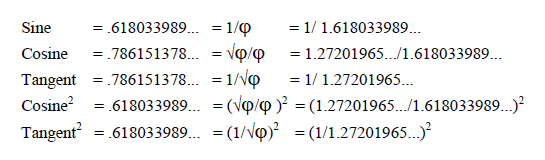This is the only angle in existence where the Cosine and the Tangent have the same values and where the Sine of the angle is equivalent to the Cosine and Tangent squared ! A drawing of a “Golden Right Angled Triangle” with this angle is as follows:Fig. 13

The Golden Right Angled triangle is found in the symmetry of, among other things, the shape of Nautilus shells and remarkably in the shape of Spiral Galaxies. The Value of Phi (Φ) the reciprocal of Phi (1/Φ) and the square root of Phi (√Φ) are all related in a perfect, indeed in a Golden symmetry.

The 35th Course of Masonry at the elevation of 1162.6 Pyramid Inches occurs at .2 of the total height since 1162.6 x 5 = 5813 Pyramid Inches whereby the circumference of the Pyramid at this level would be .8 of the Base Circumference. The Pyramid’s shape is such that the circumference is directly proportionate to its height. The thickness of the 35th Course of Masonry as measured by Professor Petrie is on average 49.5 B” which is equivalent to 49.44 Pyramid Inches. Divide this number by 100 (since the factor of 100 was seen in the Base Perimeter relationship) and we arrive at .4944 . Note that .8 divided by .4944 is equal to 1.618 which is a reasonable approximation of the value of Phi (Φ) (1.618033989) and furthermore .4944 divided by .8 is equal to .618 which is in turn a reasonable approximation of the value of the reciprocal of Phi, namely,1/Φ (.61803399...). In this way the builders of the Pyramid apparently incorporated the values of the Golden Right Angled Triangle in the design elements of the 35th Course of Masonry.

In addition to the foregoing relationship between the square root of Phi (√Φ) and a spherical body such as the Earth or of a circle or an ellipse, there is also a relationship of interest which relates to the fraction .8 of the radius of a sphere, the semi-major axis of an ellipse or a circle.

If one multiplies the measure of the Semi-Major axis of a spheroid, an ellipse, or in the case of a perfect circle, the radius, by the fraction .8 one can generate a ‘Pythagorean Triangle’. This can be appreciated in viewing the following schematic drawing:Fig. 14

The fact that the 35th Course of Masonry is .8 of the base circumference may have been intentionally designed to bring attention to the nature of geometrical symmetry.

It might also have been intentional that the number 35 was to be thought of in terms of a fraction as in 3/5. If this was the intention than it should be given consideration that if one multiplies the measure of the Semi-Major axis of a spheroid, an ellipse, or in the case of a perfect circle, the radius, by the fraction 3/5, which is equal to .6, one can also generate a ‘Pythagorean Triangle’. This can be appreciated in viewing the following schematic drawing:Fig. 15

In view of all of the above it can be argued that the 35th course of masonry points to a most interesting mathematical relationship with the shape of the Earth.

An alternate possibility for the choice by the builders of the Great Pyramid of the number 35 to be regarded as the fraction 3/5 could be that 3/5 x 43,200 = 25,920. We have seen that 43,200 is the scale of the Great Pyramid to the Earth. The number 25,920 has been subscribed as the number of years for the Axis of the Earth to precess 360 degrees. In reality, the exact number of years for the axis to precess changes over time, however, its current rate is 243.8 micro-radians or 50.3 arc-seconds per year. This would translate into 25,765 years for one complete rotation.

For an approximation, a precession rate of 50 arc-seconds per year would translate into one degree in 72 years, hence a period of 25,920 years for one cycle. In view that we have 12 Primary Constellations in our Astrological Charts this would mean that each Astrological Age would be 2,160 years in duration. Most Astrologers use this value.

At this point we may interpret whether this value of 25,920 is incorporated in any other aspect of the Great Pyramid. For this we shall investigate the internal dimensions of the Great Pyramid specifically a measurement of considerable importance, albeit somewhat concealed. This point of interest is the elevation at which the Grand Gallery Floor intersects the South Wall of the Grand Gallery which lies within the Great Step leading to the King’s Chamber.

This elevation as determined by Professor Flinders Petrie is 1689.0 +-.5 British Inches above the Pavement level surrounding the Great Pyramid. If we take the highest value of 1689.5 British Inches and thereby allow for possible subsidence this would be equivalent to 1687.64 Pyramid Inches. In view that the Pyramid was theoretically 5813.022 Pyramid Inches in height then as a ratio this would constitute :The Base Circumference of the Great Pyramid was theoretically 36,524.3 Pyramid Inches therefore:

36,524.3 x .709679406 = 25,920.5 Pyramid Inches

For this calculation to equate exactly to 25,920 then the elevation of the intersection of the Grand Gallery Floor and the South wall would have to be 0.08 British Inches or 2 mm higher. Consideration should be given to the exactness of the measurement over a period of 4560 years and which points to the highest level of competency in workmanship.

This calculation points out that at the elevation of the Grand Gallery Floor at the intersection with the South Wall at the point of entry into the King’s Chamber Passage , the circumference of the Great Pyramid has, for all intents and purposes, a theoretical measure of 25,920 Pyramid Inches.

This may be considered as quite noteworthy and points to an intentional design element in the construction of the Great Pyramid to convey knowledge of the Precession of the Earth’s Axis over a period of 25,920 years.

## 19Queen's Chamber's Apex Relationship to Entrance Passage

The Queen's Chamber Apex is 1080.1 British Inches above the Pavement Level as determined by Professor Flinders Petrie. The Entrance Passage is 524.1 British Inches horizontally inside the North edge of the Pyramid Casing. 1080.1 British Inches divided by 1.0011 gives a value in Pyramid Inches of 1078.9 . If one divides 1078.9 by the number of Pyramid Inches in one Royal Cubit one, namely 20.60663, one arrives at a ratio of 52.357. When one multiplies this value by 10 one arrives at a figure of 523.57 . This number taken as a value in Pyramid Inches would be equivalent to 524.145 British Inches. As noted, the Entrance Passage, as measured by Professor Petrie is 524.1 British inches from the North Edge of the Pyramid casing. It would appear that there is an obvious relationship between the Entrance Passage and the Apex of the Queen's Chamber incorporating the Royal Cubit measure of 20.60663 Pyramid Inches as a factor. One must take into account nominal values. This logic is found throughout the geometry of the Pyramid.

## 20Queen's Chamber Relationship to Pyramid Base

An interesting mathematical relationship may be found in regard to the Queen’s Chamber and the Base Perimeter of the Great Pyramid which is as follows. In order to arrive at this relationship one must first determine the cubic volume of the Queen’s Chamber and for this purpose we may view the schematic drawing as follows:Fig. 16

The volume may be calculated as follows:

Width x Length x Height ( of the cubic rectangle )

205.85 x 226.47 x 184.47 = 8,599,779 Cubic British Inches

Thereto one adds the volume of the Triangular Apex

½ x 205.85 x 60.63 x 226.47 = 1,413,250.4 Cubic British Inches

Therefore the total volume of the Queen’s Chamber is as follows:

8,599,779 + 1,413,250.4 = 10,013,029.40 Cubic British Inches

In order to determine the cubic Pyramid Inches one divides:

10,013,029.40 / 1.00113 = 9,980,059 Cubic Pyramid Inches

And furthermore, to determine the volume in Cubic Royal Cubits one divides:

9,980,059 / 20.606629643 = 1140.54 Cubic Royal Cubits

Therefore the Queen’s Chamber has an interior volume of 1140.54 Cubic Royal Cubits.

If we divide the value of 1140.54 Cubic Royal Cubits by 1,000,000 (10002) we arrive at a value of .00114054 .

Consideration should be given that the Great Pyramid relates to a Hemi-Sphere, that is to say half a sphere, therefore, one may multiply the value of .00114054 times 2 arriving at a value of .00228108 . It is to be given consideration that this final value should be taken simply as a numerical value and not as a specific volume. In other words what is important is the number in and of itself. We shall see this logic throughout the Pyramid’s measurements. As stated, if we multiply the base circumference of the Great Pyramid by 43,200 we arrive at the measure of 40,121.434 km as we have already seen. Now if we divide 40,121.434 km by
( 1 + .00228108 ) we arrive at a measure of 40,030.122 km for which the radius of this circumference is 6,370.9918 km. It is of considerable interest to note that the Harmonic Mean Radius of the Earth is 6,370.9928 km, which is a difference of .001 km or 1 mtr. If this Equation involving the Queen’s Chamber and the Base Circumference was purely by chance it would be extraordinary, however, we shall see further relationships which will compliment this finding and will verify that the size and shape of the Earth was known to an exceptional degree of accuracy.

The following is the calculation to determine the Harmonic Mean Radius of the Earth:

Harmonic Mean Radius of the Earth = 3ab/(a+2b)

a = Semi-Major Axis (Equatorial Radius) = 6378.137 km

b = Semi-Minor Axis (Polar Radius) = 6356.7523 km

## 21Queen's Chamber "Code Number"

Inquiry into further evidence to corroborate these findings leads us into the internal geometry of the Great Pyramid’s chambers and passages. Here we shall refer to Professor Flinders Petrie’s measurements as compiled in his book “The Pyramids and Temples of Gizeh”, 1883. It has been said that no individual has documented the measurements of the Great Pyramid to the same extent and exactitude as Professor Petrie. The accuracy of Professor Petrie’s measurements is within tolerances of 1/10s of an inch for most of his data and even 1/100s of an inch in some instances.

We will start our inquiry in the dimensions of the so-called Queen’s Chamber. As taken from Professor Petrie’s data, the size of the chamber is on average 205.85 B” (British inches) wide, 226.47 B” long, 184.47 B” high on the North and South walls and 245.10 B” high to the top of the roof ridge on the East and West walls (28). These measurements should be converted to Pyramid inches in order to appreciate the mathematical proportions which we shall see are encoded in the dimensions.

The chamber, measured in Pyramid inches, is on average 205.62 PI” (Pyramid inches) wide, 226.22 PI” long, 184.27 PI” high on the North and South walls and 244.83 PI” high to the top of the roof ridge on the East and West walls.

A schematic drawing is as follows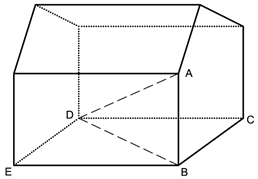Fig. 17Note: RC = Royal Cubit ( 20.60663 PI")

Once the values are converted to RC ( Royal Cubits ) then a mathematical equation can be appreciated when one adds the squares of the Height AB with the Floor Diagonal BD and the Top Corner to Bottom Opposite Corner Diagonal (hypotenuse) AD.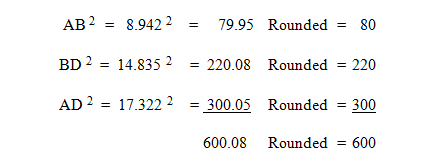Since there are four corners in the chamber one can multiply 600 by 4 = 2400.

The so-called Queen’s Chamber can be associated with the numerical value of 2400. We shall see that the number 2400 is the “Code Number” which is used to decipher the relevance of the principal measurements of the Great Pyramid’s Internal Passage measurements.

We should recall that the Giza Plateau Pyramids were arranged within a 2400 Royal Cubit diameter Circle and therein inscribed square. To find this "Code Number" imbedded within the Great Pyramid in the dimensions of the Queens Chamber, clearly corroborates an intelligent design imperative in which mathematical logic is imbued.

## 22Ante Chamber

Coming now to the Ante Chamber we will once more review the mean dimensions as taken by Professor Petrie and these are as follows: The length of the chamber is116.30 B” (116.17 PI”), breadth is
65.00 B” (64.93 PI”) and the height is 149.35 B” (149.19 PI”) (29).

A schematic drawing is as follows: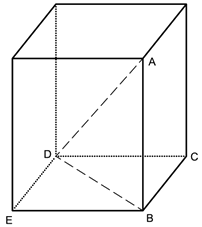Fig. 18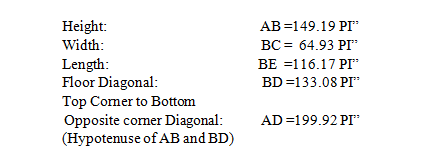The measure AD 199.92 PI” can be rounded to 200 as it falls short by a measure of only.08 Pyramid Inches which is equivalent to just 2 mm. In view that imaginary diagonal lines may be drawn from each upper corner of the chamber to the opposite lower corners, their intersection would be precisely in the center of the chamber whereby each intersected line segment would be 100 PI” in length. As there are four such diagonal lines the value would be 4 x 100 = 400. The Antechamber Chamber then can be associated with the numerical value of 400.

## 23King's Chamber

Coming now to the so-called King’s Chamber we can summarize the dimensions as follows: The base of the chamber has a mean length of 412.66 B” (412.21 PI”) and a mean base width of 206.295 B” (206.068 PI”) (30). In the matter of the height we need to look for a suitable point of reference since the floor is irregular varying by as much as 2.3 B” and the base of the wall is below this level a further 5 B” on average. It is then most suitable to use the elevation of the floor level at the end of the passage that leads into the chamber exactly where it meets the floor of the chamber for it is uniform at this point. Its elevation over the pavement level that surrounds the exterior of the building which serves as the base line from which all elevations are taken by Professor Petrie is 1692 B” (31).

It should be noted that this elevation will prove instrumental in another relationship to be examined further on in this paper.

The ceiling mean height is given as 1922.65 B”. The effective wall height is then derived by subtracting 1922.65 -1692 which yields a value of 230.65 B” or 230.397 PI” rounded to 230.4 PI”.

A schematic of the chamber is as follows:Fig. 19

 Height: Width: Length: Diagonal across End Wall: Top Corner to Bottom Opposite corner Diagonal: (Hypotenuse) AB = 230.40 PI” = 11.18 RC BC = 206.07 PI” = 10.00 RC BE = 412.21 PI” = 20.00 RC BD = 309.11 P ” = 15.00 RC ED = 515.23 PI” = 25.00 RC

The relationship between BD (15 RC), BE (20 RC) and ED (25 RC) each divided by 5 is clearly the so-called Pythagorean 3,4,5 triangle. This relationship found in the King’s Chamber is well known, however, the derived value as herein given is not immediately apparent. The associated value of the so-called King’s Chamber, may be interpreted in the following way; if one adds the cubed products of these dimensions in Royal Cubits one will find the highest value.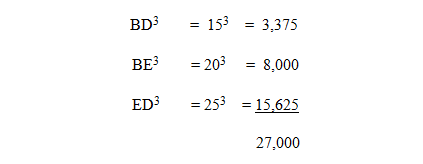As there are 4 corners in the chamber one can multiply 27,000 by 4 to arrive at a value of 108,000 which can be subscribed as the Associated Value for the King’s Chamber.

As stated earlier the Associated Value of the so-called Ante Chamber is 400 and if one multiplies the King’s Chamber Value of 108,000 by 400 one arrives at the value of 43,200,000.

## 24Granite Plug

In addition to this computation, consideration may be given to a noteworthy feature of the Great Pyramid which has served to encumber investigation of the interior chambers and has in this way underscored its prominence. This feature is the Granite Plug which blocked the lower section of the Ascending Passage. In order to by-pass this obstacle an alternate excavation was chiseled through the core masonry in order to gain access to the upper passages and chambers. This Granite Plug as measured by Professor Petrie is 15 feet long which equates to 180 Inches (32). If one multiplies 180 by the sum of the vertical and transverse height of the Ascending Passage which is 100 Pyramid Inches ( 52.78 + 47.22) then one arrives at a value of 18000. At this point one multiplies by the “Code Number” of the so-called Queen’s Chamber which as stated earlier is 2400 and the product is as follows: 180 x 100 x 2400 = 43,200,000 .

Thereby the Associated Value of the Granite Plug is 43,200,000.

## 25Great Step

Attention may now be directed to the Great Step at the top end of the Grand Gallery which has a most unusual height and thereby draws attention to its intended relevance. The measurements as taken from Prof. Petrie’s data assigns a tread depth ( from the front edge of the step face to the South wall of the Grand Gallery) of 61.7 B” and a height of 34.88 B” (33). If one divides 61.7 by 34.88 the ratio is 1.77. At this point it should be noted that the square root of Pi (√π) is 1.77245385... . It may be taken then that the associated value of the Great step is representative of the square root of Pi (√π)

At this point we can combine the associated values of the Ante Chamber and the King’s Chamber or the associated value of the Granite Plug as well as the associated value of the Great Step and multiply these by the Royal Cubit dimension as follows:

400 x 108,000 x √π x 1 RC =

43,200,000 x 1.77245385 x 52.3983685 = 4,012,143,407cm

4,012,143,407 cm / 100,000 (cm/km) = 40,121.434 km

It should be noted that the base perimeter of the Great Pyramid multiplied by 43,200 as stated earlier was 40,121.434 km and may be referred to as the Reference Circumference.

## 26Ascending Passage

At this point we may interpret the value of the Ascending Passage. Professor Petrie determined a dimension of 1546.5 B” for the length of the Ascending Passage from the intersection of the Entrance Passage floor to the Grand Gallery doorway on the E. side determined with a plumb line from the North Wall of the Grand Gallery (34). It should be noted however, that a distance of 59.8 B” is the height of the Entrance Passage from the floor to the ceiling in the angle of the Ascending Passage. Therefore, Professor Petrie determined that the actual length of the Ascending Passage floor from the ceiling of the Entrance Passage is (1546.5 - 59.8 ) = 1486.7 B”.

If one multiplies 1486.7 by the “Code Number” of the so-called Queen’s Chamber (2400) then one arrives at a value of 3,568,080 B” or 9,062,915 cm which equals 90.629 km. Subtract this value from the Reference Circumference which is 40,121.434 km and one arrives at 40,030.805 km. The radius of a circle with this circumference is 6371.100 km. The Earth has an Arithmetic Mean Radius of Curvature of 6371.100 km at a Latitude of 35.4283 degrees. At this specific Geodetic Latitude the Earth has a Radius of 6370.991 km.

The Mean Harmonic Radius of the Earth as determined by using WGS84 datum (World Geodetic System 1984) is 6370.9928 km. The difference between the value derived from the Great Pyramid’s Ascending Passage length and the actual Mean Harmonic Radius is 1.8 meters.

The equation to arrive at this value is as follows: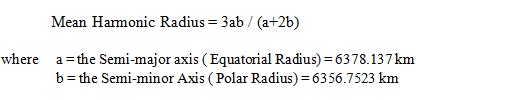In order to have calculated the precise Latitude at which the Earth has an Arithmetic Mean Radius of Curvature of 6371.100 km one can use the equations to determine the Meridional Radius of Curvature and the Transverse Radius of Curvature ( perpendicular to the Meridional) which are as follows:

Meridional Radius of Curvature as a function of Geodetic Latitude: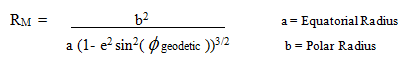Transverse Radius of Curvature as a function of Geodetic Latitude:At 35.4283 degrees Latitude the Meridional Radius of Curvature of the Earth is 6356.877 km and the Transverse Radius of Curvature is 6385.323. The Arithmetic Mean is derived as follows:

( 6356.877 + 6385.323 ) / 2 = 6371.100

## 27Passage to Queen's Chamber

The Passage into the Queen’s Chamber has a distinctive feature in that there exists a substantial step of 20.63 B” at a point 1307.0 B” from the Doorway at the Grand Gallery.(35) As one enters the Passage from the Grand Gallery there is a minor step with a height of 5.32 PI” or 5.325 B”.(36) If one adds these values (1307.0 + 5.325) the sum is 1312.325 B”. One may multiply this value by the Associated Value of the Queen’s Chamber ( 2400 ) and arrive at a product of 3,149,580 B” or the equivalent of 7,999,926 cm which is 79.999 km. Subtract this value from the Reference Circumference ( 40,121.434 km -79.999 km ) and one arrives at a measurement of 40,041.4347 km. This circumference yields a radius of 6372.7923 km. The Polar Circumference of the Earth according to WGS84 is 40,007.863 km and the Equatorial Circumference of the Earth is 40,075.017 km. The Arithmetic Mean between these two circumferences is (40,007.863 + 40,075.017) / 2 = 40,041.440 km or a radius of 6372.793 km . The difference in the circumference as given by the Pyramid’s geometry and the mean value based on WGS84 measurements is 5.3 meters. In terms of radius this would constitute a difference of only 0.8 meters.

## 28The Significance of The Location of The Great Pyramid

The significance of the geodetic location of the Great Pyramid cannot be overstated, for it verifies that the shape and size of the Earth was known to the Ancients. The mathematical relationship its location has to the Earth’s principal geometric attributes are herein addressed.

Professor Charles Piazzi Smyth (Astronomer Royal of Scotland) determined the Great Pyramid’s Astro-Geodetic Latitude using the most advanced telescopic equipment available to him in the latter 1800's. In order to determine Geodetic Latitude using Stars or the Sun one must take into account refraction of light in the atmosphere which varies due to atmospheric pressure, temperature and humidity. Astronomers make use of a set of formulas to correct for refraction of light. Refraction becomes more acute at lower Latitudes and multiple sightings of different stars may be taken in order to reduce errors. Professor Smyth’s determination of the Astro-Geodetic Latitude of the Great Pyramid in 1877 was:

29 Degrees 58 Minutes and 51 Seconds North (29.98083 Degrees)

His sighting was taken from on top of the Great Pyramid in order to reduce the effect of gravitational distortion of his plumb-line due to the mass of the Pyramid.

This Astro-Geodetic Latitude was re-confirmed by Andre Pochan in 1978. (37)

The present day geodetic location as given by GPS is :

29 Degrees 58 Minutes and 49 Seconds North (29.98027 Degrees)

A distinction must be made between the methods employed to derive the Geodetic locations. Obviously, taking sightings of stars would have been the means employed by the ancients to determine latitude as they would not have had satellite technology. As a comparison of accuracy, it is interesting to note that Airy’s Eye of the Greenwich Observatory in England (established as the Prime Meridian of the World in 1884) has a posted Latitude of 51 Degrees, 28 Minutes, 38 Seconds North which would be its Astro-Geodetic Latitude. The GPS coordinates of the Greenwich Observatory are 51 Degrees, 28 Minutes and 40 Seconds North. The reason for this variance is in part that the local gravity at any location of the Earth and hence a plumb-line, is influenced by the density of the Earth’s crust, which varies considerably from place to place. Particular variations occur in the proximity of mountain ranges and high iron concentrations in the crust strata. It is also to be given consideration that the exact position of the North Pole relative to the Earth experiences what is called “Polar Motion” due to changes in the center of Mass ie: changes in Ocean Currents.

This can effect Latitude readings by as much as 1.5 arc-seconds or 20 meters on the Earth’s surface. For all intents and purposes it is therefore to be given consideration that the Astro-Geodetic location of the Great Pyramid be taken as the location that the ancients would have had the means to measure. Any calculations for the Earth’s geometry giving consideration to the Pyramid’s location would have been contingent on the Astro-Geodetic determinations.

The Passage into the Queen’s Chamber provides a measurement which has relevance to the Pyramid’s location on Earth. If one follows the floor line from the major step back to the Grand Gallery and continuing a straight line through the minor step and proceeding down the slight incline to where the floor intersects the North wall of the Grand Gallery you will find this distance to be 1308.3 B”. Multiply this length by the Queen’s Chamber value ( 2400 x 1308.3 ) and one arrives at a product of 3,139,920 B” or 7,975,389.9 cm which equates to 79.7539 km. Subtract this value from the Reference Circumference ( 40,121.434 - 79.7539 ) and one arrives at a value of 40,041.680 km of which the radius would be 6372.831 km. It is of considerable interest to know that the radius of the Earth at the location of the Great Pyramid determined as at 29.98083 degrees North Geodetic Latitude is 6372.8306 km. It would appear that the location of the Pyramid was known to the Ancients to a level of accuracy comparable to that which was known in the late 1800's . The radius of the Earth at 29.98083 degrees North was known. The difference in radius is only 0.7 meters.

The choice of 29.98083 degrees North Latitude appears at first glance to be either an attempt to fix its location at 30 degrees North, with a considerable inaccuracy, or that it was just simply a convenient location. Neither of these contentions appear to be the case when one gives careful consideration to the following mathematics which must have been considered in the choice of location of the Great Pyramid by the Ancients.

In order to understand the relevance of the location one must first calculate the Meridional Radius of Curvature for 29.98083 Degrees North Latitude, which is 6351.3585 km.

Secondly, consideration is to be given to the “Radius of a Sphere of Equal Volume” as that of the Earth which is given by the equation:The ratio between these values is as follows: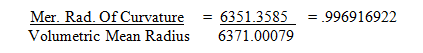Once this ratio is known then consideration may be given to the following mathematical steps:

1) one may take its cubed root namely: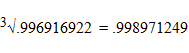2) one may divide its cubed root by the square root of 3 namely: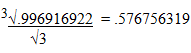3) one may take its cubed root and multiply it by the square root of 2 and divide it by the square root of three as follows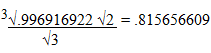Each of these three derived ratios have a distinct geometric relevance with the shape of the Earth in relation to the Lateral Radius of the Earth at 29.98083 Degrees North Latitude. These points of interest shall be herewith addressed.

It is important to note that at 29.98083 Degrees North Latitude ,(the position of the Great Pyramid as determined by Professor Piazzi Smyth and confirmed by Andre Pochan in 1978), the Earth’s Lateral Radius is 5529.3188 km.

At this point we may multiply the Lateral Radius of the Earth at 29.98083 Degrees Geodetic Latitude by the three separate derived ratios that we have noted above namely:

1. 5529.3188 x .998971249 = 5523.6305 km
2. 5529.3188 x .576756319 = 3189.0696 km
3. 5529.3188 x .815656609 = 4510.0254 km

These three Lateral Radii occur on the Earth at significant Latitudes which are addressed as follows:

1) The Lateral radius of 5523.6305 km occurs at 30.08335922 Degrees Geodetic Latitude.

1. The Geo-centric Radius of the Earth at this Geodetic Latitude is 6372.7976 km. It should be noted that the Quadratic Radius of the Earth as given by WGS 84 data is 6372.79755 km as given by the following equation:
• Qr = √(( 3a2 + b2 ) / 4) = 6372.79755 km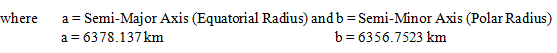Furthermore, it should be noted that the Equatorial Radius of 6378.137 multiplied by the square root of 3 and divided by 2 is equal to 5523.6287 km as given in the following equation: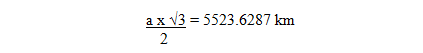The difference between the Lateral Radius calculated from the Great Pyramid’s location and this radius calculated from the Earth’s Equatorial Radius using WGS 84 data is 1.8 meters.

It should be noted that the Lateral Radius of 5523.6287 km occurs at precisely 30.08339202 Degrees Geodetic Latitude. This point is precisely the Geometric Mean between 30 Degrees Geocentric Latitude and 30 Degrees Geodetic Latitude.

(Note that 30 Degrees Geocentric Latitude occurs at 30.16692385 Degrees Geodetic Latitude.)

It is also of considerable importance to note that at precisely 30.08339202 Degrees Geodetic Latitude the Earth’s Semi-Minor Axis is exactly bisected.

The Geodetic Latitude derived from the Great Pyramid’s Location ( as determined by Professor Piazzi Smyth ) through the above mathematical equation is 30.08335922. This constitutes a difference of .0000328 degrees which is equivalent to 0.118 Seconds of Arc.

2) The second Lateral Radius as given from the aforementioned equations is 3189.0696 km.

It is immediately apparent that this measure relates to the distance that bisects the Equatorial Radius (Semi-Major Axis) as may be appreciated in the following equation:The difference in Radii is 1.1 meters.

The Geodetic Latitude at which the Lateral Radius is exactly half that of the Equatorial Radius occurs at 60.08325223 Degrees which coincides with the Geocentric Latitude of 59.91660774. Note that the Geometric Mean between these two angles is exactly 60 Degrees.

The Tangent of 60 degrees is equal to the square root of 3 (√3). It is of interest to note that the Lateral Radius of 3189.0696, derived using the Pyramid's Location as a basis point, involved a division by the square root of 3 (√3) as may be appreciated in reviewing the above noted equations.

The Geodetic Latitude derived from the Great Pyramid’s Location ( as determined by Professor Piazzi Smyth ) through the above mathematical equation is 60.08324084.This constitutes a difference from the geodetic Latitude at which the Equatorial Radius is exactly bisected of .0000114 degrees which is equivalent to 0.04 Seconds of Arc.

3) The third Lateral Radius as given from the above equations is 4510.0254 km

This Lateral Radius occurs at 45.0962013 Degrees Geodetic Latitude, which coincides with 44.903777 Degrees Geocentric Latitude. The Geometric Mean between these two angles is 44.99998915 Degrees. In other words its variance from exactly 45 degrees is 0.00001085 degrees or 0.04 Seconds of Arc.

The Lateral Radius of the Earth which compliments an exact Mean angle of 45 Degrees is given as follows: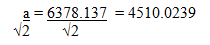The difference in Radii is 0.00148 km or 1.48 meters.

It is of interest to note that the derived Lateral Radius of 4510.0254 involved a multiplication by the square root of 2 (√2) as may be appreciated in reviewing the above noted equations.

The Lateral Radius of the Earth at the location of the Great Pyramid is significant as it relates mathematically to three particularly important geometric considerations. As it is apparent, these points of interest include the bisection of the Semi-Minor Axis, the bisection of the Semi- Major Axis and the lateral Radius at a point at 45 degrees (Geometric Mean) and in all cases to an exceptional degree of accuracy.

In order to have determined the precise Geodetic Latitude appropriate to locate the Great Pyramid which would satisfy the equations as noted, mathematical equations named “Quartic Equations” would have to be solved. Conversely, if this was not done then trial and error calculations would have to be made to determine the ideal location to have the equations work out.

In view of the exactitude and sheer brilliance behind the architecture of the Great Pyramid it is more probable than not that Quartic Equations were applied.

It is of great interest to note that these equations are the highest level of Algebra that may be solved and where “the solutions are given in terms of root extractions and rational operations performed on the coefficients of the given equation.” In relatively recent history, during the Italian Renaissance, it was Geronimo Cardano that published the general method for solving quartic equations in Ars Magna (1545) although the solution was credited to Cardano’s assistant Ludovico Ferarri. Many attempts by the greatest minds in mathematics attempted to solve higher level algebraic equations, however it was proven by A. Ruffini (published in 1813) and independently by N.H. Abel (published in 1827) that higher order equations could not be solved by radicals. (38)

In short, to locate the Great Pyramid precisely, the highest level of mathematics would need to have been employed, not to mention extraordinary exactitude in determining the Astro-Geodetic Latitude.

In this regard, the location of the Great Pyramid is the ideal location to indicate an exceptional degree of knowledge in the size and shape of the Earth, an extraordinary proficiency in astronomy combined with an exceptional level of competency in advanced mathematics. The full understanding of the location of the Great Pyramid is of utmost significance.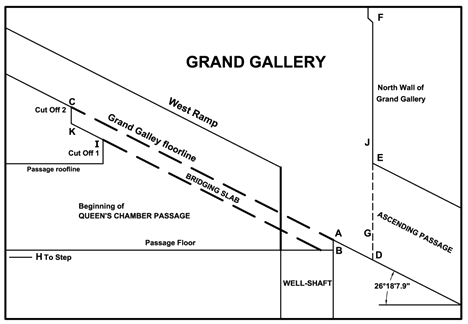Fig. 20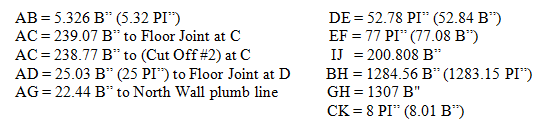## 30Bridging Slab at Entrance to Queen's Chamber Passage

At the entrance to the passage that leads to the Queen’s Chamber there once existed a Bridging Slab that sealed off the passage. The dimensions of this slab are of considerable interest. The beginning of the slab would have been at the small step at the entrance to the Queen’s Passage which is 25 PI” (25.03 B”) from the point at which Professor Petrie measured the beginning of the Grand Gallery floor. The distance to the face of the 2nd “Cut Off” along the Grand Gallery floor was determined by Professor Petrie to be 263.8 B” from the beginning of the Grand Gallery Floor. There is also a joint in the floor at 264.1 B” from the beginning of the Grand Gallery floor. (39) In taking the mean between these two values (263.8 +264.1) / 2 we arrive at a value of 263.95 B”.

Therefore the length of the Bridging Slab would have been (263.95 - 25.03) = 238.92 B” or 238.66 PI”. Consideration should be given to the step height which would be concealed once a slab is in place. This step height was seen as instrumental in understanding the intended value of the Queen’s Passage measurement as we have seen earlier. It is evident that its intended purpose was to fulfill an important function in determining pertinent measures. In this case we may add its dimension to the length of the Bridging Slab whereby 238.92 + 5.326 = 244.246 B”. The width of the Bridging Slab is 2 Royal Cubits which may be considered as simply a numerical value, therefore we might multiply the value of 244.246 B” x 2 to arrive at a value of 488.492 B”. As before this value should be multiplied by the Queen’s Chamber Associated Value whereby 2400 x 488.492 = 1,172,380.8 B” which equals 2,977,844 cm or 29.778 km. It is of great interest to note that the Average Orbital Velocity of the Earth in its orbit about the Sun is 29.783 km per Second. This constitutes a difference of 0.0168 %. Furthermore one should give consideration to the measurement of the thickness of the slab which is 8 PI”. (40) If one multiplies this measure by 2400 ( the Queen’s Chamber Code Number) one arrives at 19,200 PI” which is equivalent to .488 km. When one adds this value to 29.778 km one arrives at 30.266 km. It is of interest to note that the maximum orbital speed of the Earth is 30.287 km/ second.

Furthermore if one subtracts .488 km from 29.778 one arrives at a value of 29.290 km. The minimum orbital speed of the Earth is 29.291 km/ second. To have arrived at the knowledge that the Earth orbits the Sun and to have been able to calculate its speed this accurately is a giant leap of knowledge and it is most befitting that the Bridging Slab is placed precisely at the entrance to the Grand Gallery and Queen’s Chamber whereby it serves to gain access to the upper Gallery and subsequently the King’s Chamber. With the Bridging Slab removed one gains access to the Queen’s Chamber wherein one finds the code number 2400 which is the key to understanding the relevance of the passage ways and Grand Gallery measurements. It serves in this way as a striking symbolic value which underscores a scientific breakthrough. The rediscovery over 4400 years later that the Earth orbits the Sun by Nikolaus Kopernicus has had a profound effect on the understanding of our Universe and our place in it, not only in a physical sense, but rather, perhaps more profoundly, in a philosophical sense

## 31Elevation of Passage to Queen's Chamber

As we have seen, the Bridging Slab served to indicate the Velocity of the Earth in its orbit about the Sun with considerable accuracy. It is now of interest to give consideration to the importance of the elevation of the Passage to the Queen's Chamber which the Bridging Slab would conceal if it was in place and determine whether there exists complimentary information concerning the Earth and the Sun.

Professor Petrie measured the elevation of the Passage above the exterior base of the Pyramid (Pavement Level) at 5 points along its length from the entry at the Bridging Slab to the Major Step. The average of his measurements was found to be 856.54 B" (British Inches) which would correspond to 855.6 PI" (Pyramid Inches).

If one multiplies 856.54 B" by 2400 (The Queen's Chamber's Associated Value) and by 180,( the Associated Value of the Granite Plug in the Ascending Passage), one would in effect be multiplying by 432,000 which is 10 times the ratio of the Pyramid to the Earth as we have seen earlier. Furthermore, one may multiply this value by 100 and by 1000 as both of these factors are seen in the geometry aspects of the Pyramid. The end result is simply; one may take the elevation of the Queen's Chamber Passage and multiply it by
4.32 x 1010 as follows: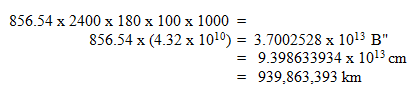The distance of 939,863,393 km is a considerable value as it is the Orbital Circumference of the Earth in its path about the Sun to an extremely high degree of accuracy. It should be noted that the Orbit of the Earth is slightly elliptical however, without resorting to Calculus one may reasonably take the value of 939,863,393 km and divide it by 2π and arrive at a radius of 149,583,905 km which is an appropriate approximation of the mean radius.

The generally accepted Semi-Minor Axis of the Earth's Orbit is 149,576,999.8 km and the Semi-Major Axis is 149,597,887.5 km therefore a simple average is 149,587,443.7 km. The Value as given from the elevation of the Queen's Chamber Passage is accurate to 99.9976%.

Furthermore, if one divides 939,863,393 km by 86,400 (seconds in one Solar day) and by 365.25636 (Sidereal Year: the number of days for the Earth to complete one revolution of its orbit) one arrives at a value of 29.782 km per second as on average Orbital Velocity of the Earth about the Sun. The generally accepted average Orbital Velocity is 29.783 km per Second as has been stated earlier. One should take note that the Bridging Slab indicated a value of 29.778 km per Second. Allowing for tolerances in measure, it is apparent that the Bridging Slab and the Passage into the Queen's Chamber are complimentary in providing accurate information in respect to the Orbit of the Earth about the Sun.

## 32Cut Off Under Bridging Slab

Under the Bridging Slab there exists a “Cut Off” of the Passage to the Queen’s Chamber at the point of intersection with the Grand Gallery floor which was measured by Professor Petrie to be 223.7 B” from the beginning of the Grand Gallery floor at the end of the Ascending Passage. It should be noted that the point plumb from the North Wall is .3 B” further North therefore we must add .3 B” to 223.7 B” for the total length of 224 B” from the point plumb with the North Wall. Since the slope of the floor is 26 degrees 18 minutes and 9.7 seconds this gives us a calculated horizontal distance from the North Wall to the Cut Off of 200.808 B”. As before this value should be multiplied by the Associated Value of the Queen’s Chamber whereby 2400 x 200.808 = 481,939.2 B” which equals 1,224,124.5 cm and which is equivalent to 12.2412 km. This value may be subtracted from the Reference Circumference as follows: (40,121.434 - 12.2412) = 40,109.1928 km which when taken as a circumference would yield a radius of 6383.5763 km. The Earth has a Transverse Radius of Curvature of 6383.5763 km at 30.29395 degrees Latitude and at which point it has a Meridional Radius of Curvature of 6351.6618 km. The Gaussian or Geometric Mean Radius of Curvature is 6367.5991 km which is given by the following equation:

• 6367.5991 = √(6383.5763 x 6351.6618)

It is of interest to note that the Meridional Radius of Curvature at exactly 45 degrees Geocentric Latitude is 6367.597 km. Evidently the Cut off indicates the Latitude at which the Gaussian or Geometric Mean Curvature of the Earth is equal to the Meridional Radius of Curvature at 45 degrees Geocentric Latitude to within an accuracy of 2.1 meters.

The Grand Gallery, which is above the Ascending Passage, is the next item to be given consideration. Professor Flinders Petrie determined that the sloping length of the ceiling of the Grand Gallery from the North wall to the South wall is 1838.6 B” and the floor length from the North wall to the South wall is 1883.6 B” (41). The difference in length between the floor and ceiling is thus (1883.6 - 1838.6) = 45 B”. There are 7 laps along the length of the Grand Gallery walls at various spacings vertically. The following are schematic drawings of the Grand Gallery:Fig. 21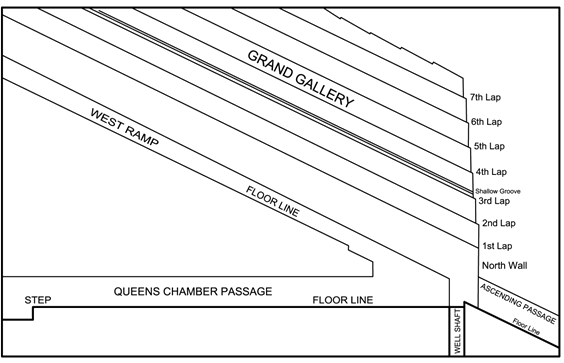Fig. 22Fig. 23

Consideration, should be given to the virtual length of the Grand Gallery at these Lap elevations as these measurements are of considerable importance. The maximum height of the Grand Gallery is 346 British Inches (the average of 15 measurements) as measured by Prof. Piazzi Smyth. (42) The virtual length of the Gallery decreases proportionately with its height whereby once the elevation of a lap is determined then the virtual length can be calculated easily. Since the length of the ceiling as determined by Professor Petrie is 1838.6 British Inches and the length of the floor is 1883.6 British Inches, the proportion is 45 / 346 , which is equal to 0.13006. This is to say, that for every British inch in height, the Gallery’s virtual length is shorter by 0.13006 B” as one progresses upward from the floor.

The North and South walls are vertical up until the second lap whereby the first lap length is the same as the floor length at 1883.6 B”. The 7th lap is located at 33.6 B” from the ceiling or 312.4 B” from the floor. By multiplying the height by the ratio we arrive at the amount by which the Gallery is shorter than the floor length: 312.4 x .13006 = 40.6 B”. At this point one can subtract this value from the floor length: 1883.6 - 40.6 = 1843 B” . The virtual length of the 7th Lap is by this calculation 1843 B” in length. The Arithmetic mean between the lengths of the 1st and 7th Lap is : (1883.6 + 1843 ) / 2 =1863.3 B”. This value may then be multiplied by the Queen’s Chamber Code Number as follows: (2400 x 1863.3) = 4,471,920 B” or 11,358,667 cm which equals 113.5867 km.

This value may be subtracted from the Reference Circumference as follows: ( 40,121.434 - 113.5867 ) = 40,007.8473 km which would yield a radius of 6367.4467 km.

The Arithmetic Mean value between the radius of the Earth at the Poles and at the Equator is ( 6356.752 + 6378.137 ) / 2 = 6367.444 km. The difference in values is 2.7 meters.

The 2nd Lap is located at 129.78 PI” or 129.9 B” above the floor of the Grand Gallery.(43) (The Ascending Passage has a vertical measure of 52.78 PI” and t2nd lap is located at 77 PI” above the doorway into the Grand Gallery) Therefore, the 2nd Lap is located at 129.78 PI” or 129.9 B”. Multiply 129.9 x .13006 = 16.9 B”. This is the amount by which the virtual length of the Grand Gallery at the level of the 2nd Lap is shorter than the floor length, therefore, the virtual length of the Grand Gallery at the level of the 2nd lap is (1883.6-16.9) = 1866.7 B”. As before, this value may be multiplied by the Queen’s Chamber Code Number as follows: (2400 x 1866.7) = 4,480,080 or 11,379,393 cm which equals 113.79393 km. Subtract this from the Reference Circumference as before: ( 40,121.434 - 113.7939 ) = 40,007.640 which would yield a radius of 6367.4137 km. The Radius of the Earth at exactly 45 degrees Geocentric Latitude is 6367.4177 km. The difference in values is 4 meters.

The 3rd lap is located at 166.2 B” above the floor as measured by Professor Piazzi Smyth. In applying the same method as previously noted the virtual length of the Grand Gallery at this level would be 1862 B”. As before, this value may be multiplied by the Queen’s Chamber’s Code Number as follows: (2400 x 1862) = 4,468,800 or 11,350,742 cm which equals 113.50742 km. Subtract this from the Reference Circumference as before:( 40,121.434 - 113.50742 ) = 40,007.9266 which would yield a radius of 6367.4593 km.

At 45.072159 degrees Geodetic Latitude the Earth’s radius is 6367.4626 km and at this exact point the Meridional Radius of Curvature is also 6367.4626 km. This equivalency of radii exists only at this particular point of Latitude. The equation to solve for this equivalency is as follows:

Radius at Geodetic Latitude = Meridional Radius of Curvature at Geodetic LatitudeAt this specific Latitude the Transverse Radius of Curvature is 6388.8653 km which is identical to the Arithmetic Mean between the Transverse Radius of Curvature at the Equator and the Transverse Radius of Curvature at the Poles.

( 6378.137 km + 6399.5936 km) / 2 = 6388.8653 km

The 3rd Lap clearly indicates the point on Earth where the Meridional Radius of Curvature is equal to the Radius to an accuracy of 3.3 meters. It also indicates the Latitude at which the Arithmetic Mean Transverse Radius of Curvature occurs.

The 4th lap is located at 134.3 B” from the ceiling or 211.7 B” from the floor of the Gallery. In applying the same method as previously noted the virtual length of the Grand Gallery at this level would be 1856.1 B”. As before, this value may be multiplied by the Queen’s Chamber’s Code Number as follows: (2400 x 1856.1) = 4,454,640 or 11,314,776 cm which equals 113.14776km. Subtract this from the Reference Circumference as before:( 40,121.434 - 113.14776 ) = 40,008.286 which would yield a radius of 6367.5165 km. The Meridional Radius of Curvature of the Earth at the Equator is 6335.4393 km and at the Poles it is 6399.5936 km. If one calculates the Arithmetic Mean between these values as follows:( 6335.4393 + 6399.5936 )/2 this equals 6367.51645 km. The 4th lap clearly defines the Arithmetic Mean between the Meridional Radius of Curvature of the Earth at the Equator and at the Poles.

The 5th lap is located at 100.6 B” from the ceiling or 245.4 B” from the floor of the Gallery. Once again in applying the same calculation method as previously noted the virtual length of the Grand Gallery at the level of the 5th lap is determined to be 1851.7 B”. Multiply by the Queen’s Chamber’s Code Number as follows: (2400 x 1851.7) = 4,444,080 B” or 11,287,953 cm which equals 112.8795 km. Subtract this from the Reference Circumference as before:( 40,121.434 - 112.8795 ) = 40,008.5545 which yields a radius of 6367.559 km.

The Earth has a Meridional Radius of Curvature of 6367.559 km at 45.158 degrees Geodetic Latitude.

The Arithmetic Mean value for the Equatorial Meridional Radius of Curvature and the Polar Meridional Radius of Curvature is derived in the following equation:

Arithmetic Mean Rad.of Curv. = (Equatorial Merid. Rad of Curv. + Polar Merid. Rad. of Curv.)/ 2

6367.5165 km = (6335.4393 km + 6399.5936 km) / 2

Furthermore, it should be noted that the Meridional Radius of Curvature at 45 degrees Geocentric Latitude (45.19242 degrees Geodetic Latitude) is 6367.5973 km.

Furthermore, it should be noted that the Meridional Radius of Curvature at 45 degrees Geocentric Latitude (45.19242 degrees Geodetic Latitude) is 6367.5973 km.

As noted the measure as given by the 5th Lap is 6367.559 km constituting a difference of approximately 2 meters. The 5th Lap indicates the point on the Earth that is the Arithmetic Mean between the Meridional Radius of Curvature at 45 degrees Geocentric Latitude and the Point on Earth which is the Arithmetic Mean between the Polar and Equatorial Meridional Radius of Curvatures.

The 6th lap is located at 67.3 B” from the ceiling or 278.7 from the floor of the Gallery. Once again in applying the same calculation method as previously noted the virtual length of the Grand Gallery at the level of the 6th lap is determined to be 1847.4 B”. Then multiply by the Queen’s Chamber’s Code Number as follows: (2400 x 1847.4) = 4,433,760 B” or 11,261,741 cm which equals 112.6174km. Subtract this from the Reference Circumference as before:( 40,121.434 - 112.6174 ) = 40,008.8166 which yields a radius of 6367.601 km.

The Meridional Radius of Curvature at 45 degrees Geocentric Latitude (45.19242 degrees Geodetic Latitude) is 6367.5973 km. The difference amounts to 4 meters.

In addition to the 7 laps in the Grand Gallery, particular interest may be given to a scored groove that is located just above the 3rd lap that runs the full length of the Gallery’s walls. The lower edge of the groove is at 172.1 B” from the floor of the Gallery and the upper edge is at 179 B”, therefore, its midline is at 175.55 B”(as measured by Professor Flinders Petrie). To calculate the virtual length of the Grand Gallery at this point one can use the same formula as before, whereby the virtual length of the Gallery at this point is calculated to be 1860.8 B”. As before, this value may be multiplied by the Queen’s Chamber’s Code Number as follows: (2400 x 1860.8) = 4,465,920 B” or 11,343,426 cm which equals 113.43426 km. Subtract this from the Reference Circumference as before: ( 40,121.434 - 113.43426 ) = 40,007.9997 which yields a radius of 6367.4709 km.

It should be noted that at precisely 45.04812 degrees Geodetic Latitude (44.855696 degrees Geocentric Latitude) the Earth has a radius of 6367.471577 km. At this Latitude the Meridional Radius of Curvature is 6367.43568 km. It should be noted that the Geometric Mean between the Polar and Equatorial Meridional Radius of Curvature is 6367.43568 km. as can be seen in the following equation:

Mean Meridional Rad. Of Curv. = √(Polar Merid. Rad.of Curv. x Equat. Merid. Rad.of Curv.)

6367.43568 km = √( 6399.593626 x 6335.439327 )

Also at this Latitude, the Transverse Radius of Curvature is 6388.85631 km. It should be noted that the Geometric Mean between the Polar and Equatorial Transverse Radius of Curvature is 6388.85631 km. as can be seen in the following equation:

Mean Trans. Rad. Of Curvature = √(Polar Transv. Rad.of Curv. x Equat. Trans. Rad.of Curv.)

6388.85631 = √( 6399.593626 x 6378.137 )

It is also interesting to note the following relationship at 45.04812 degrees in the equation as follows:

1 - e2(Sine 45.04812 degrees)2 = .996647187 where e = .081819190842622

(Eccentricity of the Earth's shape)

The axis Ratio of the Earth, that is to say the ratio between the Minor and Major Axis, ( Polar Radius / Equatorial Radius ), namely 6356.7523 / 6378.137 = .996647187 .

Furthermore, as noted, at 45.04812 degrees Latitude, the Meridional Radius of Curvature is 6367.43568 km and the Transverse Radius of Curvature is 6388.85631 km. The Geometric Mean Radius is therefore :

√(6367.43568 x 6388.85631) = 6378.137 km

It is noteworthy to recognize that this is identical to the Equatorial Radius of the Earth (Semi-Major Axis).Therefore it is to be appreciated that the Groove that runs the length of the Grand Gallery indicates the Radius of the Earth at which the Latitude reflects the Geometric Mean between the Equator and a Pole to within an accuracy of less than I meter and indicates the point at which the Geometric Mean Radius of Curvature is identical to the Equatorial Radius.

Furthermore, as noted earlier, the lower edge of the Groove is found to be at 172.1 B” from the floor of the Gallery. If one multiplies this value by 2 and by 2400 (the Queen’s Chamber Associated Value) one arrives at 825,080 B” which equates to 2,098,241 cm or 20.98241 km. When you add this value to the Reference Circumference; 40,121.434 + 20.9824 you arrive at a value of 40,142.4164 km. The Radius for such a circumference is 6388.864 km. It should be noted that the Arithmetic Mean between the Polar and Equatorial Transverse Radius of Curvature is 6388.8653 km. as can be seen as follows:

Arithmetric Mean Trans. Rad. Of Curv. = (Polar Trans.Rad. Of Curv.+ Equat.Trans.Rad. Of Curv.) / 2

6388.8653 km. = (6399.593626 + 6378.137) / 2

The height of the bottom of the Groove in the Grand Gallery indicates the Arithmetic Mean Transverse Radius of Curvature of the Earth to within an accuracy of 1.3 meters.

Consideration should be given to the fact that 0.1 B” in the measurements of the Grand Gallery lengths translate into a measurement of 1 meter in terms of the calculated radius measurements. The precision of the construction detail is quite extraordinary and conveys an exceptional accuracy in Geodetic knowledge of the Earth.

The Grand Gallery Height has an interesting relevance when consideration is given to the South Wall. The maximum total height of the Grand Gallery is 346 British Inches which is the average of 15 measurements taken by Professor Piazzi Smyth. In the case of the South wall there exists a horizontal passage which leads into the King’s Chamber having a height of 43.75 British Inches (44). Furthermore the elevation of the floor of this passage at the South wall of the Grand Gallery is 3.9 British Inches (the average of 3 measurements as taken by Professor Flinders Petrie) above the floor level of the Grand Gallery (45). This so-called hidden step appears to fulfill the function of adjusting the elevation of the passage floor very accurately to define a crucial measurement. In view of these measurements we can calculate the height of the Grand Gallery South wall from the ceiling of the Horizontal Passage which leads into the King’s Chamber up to the ceiling of the Grand Gallery as follows: 346 - 43.75 - 3.9 = 298.35 British Inches which is equivalent to 298.022 Pyramid Inches. It is noteworthy to consider that the equation relating to the flattening of the North and South Poles of the Earth as per WGS 84 is given as 1/298.257223563 which equals .3352810665 % oblateness of the Earth sphere (46). It appears then, that the equation for the flattening at the poles was incorporated into the design height of the Grand Gallery to a very high degree of accuracy. The accuracy of the stone work or quite possibly the measurements as taken are within less than .25 Pyramid Inches (6 mm) in error.

Another feature of the Grand Gallery are Ramps on either side of the sloping floor, which are 21 PI” in height perpendicular to the slope.(47) If one multiplies this measure by the Associated Value of the Queen’s Chamber (2400 x 21) = 50,400 PI” converted to metric this would be 128,156.7 cm or 1.2816 km. Subtract this value from the Reference Circumference: 40,121.434 - 1.2816 = 40,120.152. This would yield a radius of 6385.3206 km. At 35.4214 degrees Geodetic Latitude the Transverse Radius of Curvature of the Earth is 6385.3206 km and it is at this point that the radius of the Earth is 6370.9935 km. As noted earlier, the Mean Harmonic Radius of the Earth is 6370.9928 km. The difference in values is 0.7 meters. The Ramps thereby appear to indicate the point on the Earth where the Mean Harmonic Radius of the Earth occurs.

In addition to the above noted measurements of the Grand Gallery one should appreciate that the over- all dimensions of the Grand Gallery may convey knowledge of the Earth’s overall dimensions

The maximum total height of the Grand Gallery as earlier stated is 346 British Inches. The length of the ceiling or roof is 1838.6 British Inches, whereas the length of the floor is 1883.6 British Inches. The difference in length between the ceiling and floor is therefore 45 British Inches. If one adds 346 + 45 this equals 391 British Inches. Since the Pyramid relates to a Hemi- Sphere we may multiply 391 x 2 giving a value of 782 British Inches. At this point we may subtract 782 from the total floor length of 1883.6 giving a value of 1101.6 British Inches. As before we may multiply this value by the Queen’s Chamber’s Code Number of 2400 arriving at 2,643,840 British Inches. This measure is equivalent to 6,715,347.7 cm or 67.1535 km.

As noted the Earth’s Polar Circumference as per WGS 84 data is 40,007.863 km and the Equatorial Circumference is 40,075.017 km. The difference between the minimum and maximum circumferences of the Earth is therefore 67.154 km.

The value as given by the Grand Gallery’s measurements has a variance of only 0.5 meters from the WGS 84 data.

The first consideration is that the Maximum Total Height of the Grand Gallery as determined by Professor Piazzi Smyth is 346 British Inches, which when divided by 1.0011, is equivalent to 345.6 Pyramid Inches. If one multiplies 345.6 times 250 one arrives at a value of 86,400. It should be recalled that 250 was seen as a factor in the exterior Base Perimeter measure, whereby each base side was found to be equivalent to 250v√ x RC (Royal Cubit). The number 86,400 is the number of Seconds in one Solar Day and to find it incorporated in the Design of the Grand Gallery is intriguing.

Secondly, we may give consideration to the total sloping length of the Grand Gallery which Professor Petrie determined to be 1883.6 British Inches. It is to be considered whether this measure conveys a relationship of values found elsewhere in the Pyramid's design. To start with, the number of Solar Days in a Winter Solstice Year in 2560 BC was found to have been 365.243 days. This value multiplied by 100 is suggested to have been the theoretical Base Perimeter of the Pyramid in Pyramid Inches. If one multiplies 365.243 times 25, as there are 25 Pyramid Inches in a Sacred Cubit, one arrives at 9131.075 Pyramid Inches, which is the length of each Pyramid Base Side. Now if one multiplies 9131.075 times the number of Pyramid Inches in one Royal Cubit, which has 20.60663 Pyramid Inches, one arrives at a value of 188,160.7 . By dividing this number by 100 one arrives at 1881.607 . If one regards this as a measure in Pyramid Inches and multiply this value by 1.0011 one arrives at an equivalency of 1883.677 British Inches. As indicated above, Professor Petrie determined the sloping length of the Grand Gallery to be 1883.6 British Inches which constitutes a difference of less than 8/100 ths of an Inch. If the distance was meant to convey the knowledge as herewith maintained, the construction accuracy is outstanding.

It may be appreciated that both the Maximum Height as well as the Sloping Length of the Grand Gallery floor was designed with the Orbital period of the Earth about the Sun and the Earth's Rotation Rate in mind.

## 35Height of The Ante Chamber

The Ante Chamber is located in the passage way leading into the King’s Chamber. The height of the chamber as measured by Professor Petrie is given as 149.64 British Inches as a mean height at 61 British Inches from the North Wall and 149.61 British Inches as a mean height at the South Wall. The average height is therefore 149.625 British Inches ((149.64 + 149.61)/2 = 149.625 British Inches). If one multiplies this measurement by 10 and by the Queen’s Chamber Associated Value of 2400 one arrives at a measure of 3,591,000 British Inches which is equivalent to 9,121,132 cm or 91.2113 km. If one subtracts this measurement from the Reference Circumference of 40,121.434 km one arrives at 40,030.2227 km for which the radius would be 6371.0078 km. It is of considerable interest to note that the Arithmetic Mean Radius of the Earth is 6371.00877 km which constitutes a difference of less than 1 meter.

Arithmetic Mean Radius = (2a+b)/3

(where a = Semi-Major Axis and b = Semi-Minor Axis)

Within the Geometry of the Great Pyramid are found values for the dimensions of the Earth to the highest degree of accuracy which are given by dimensions and associated values contained in the passage ways and chambers of the Great Pyramid and which further compliment the external dimensions. The Grand Gallery specifically details the oblate nature of the Earth in giving key measurements that detail the radii and radii of curvatures at specific points of interest.

The Location of the Great Pyramid itself indicates an advanced knowledge of the shape and size of the Earth

It is of interest to see the values for the shape of the Earth as determined in the past and in this way appreciate the advanced knowledge that is apparent in the Great Pyramid’s geometry.

## 36Historical Earth Ellipsoids

 Reference Ellipsoid name Modified Everest (Malaya) Everest (1830) Clarke (1880) International (1924) WGS66 (1966) GRS-67 (1967) WGS-72 (1972) WGS-84 (1984) Equatorial radius (m) 6,377,304.063 6,377,276.345 6,378,249.145 6,378,388. 6,378,145. 6,378,160. 6,378,135. 6,378,137. Polar radius (m) 6,356,103.039 6,356,075.413 6,356,514.870 6,356,911.946 6,356,759.769 6.356,774.516 6,356,750.52 6,356,752.3142 Inverse Flattening 300.801699969 300.801697979 293.465 297 298.25 298.247167427 298.26 298.257223563

## 37The Golden Ratio in The Internal Geometry

Knowledge of the Golden Ratio ( Phi ) was evident in the external measurements of the Great Pyramid. We shall now see that this value was incorporated in the internal geometry as well. Professor Petrie measured the horizontal length of the Grand Gallery and confirmed its measure to be 1688.9 B” at the floor level and 1648.45 B” at the roof giving a difference of 40.45 B”. The “Sacred Cubit” used in Egypt was 25 Pyramid Inches. For instance the side length of each base side of the Pyramid was 365.243 Sacred Cubits which when multiplied by 4 would give a total of 1460.972 Sacred Cubits. This number multiplied by 25 PI" per Sacred Cubit yields 36,524.3 Pyramid Inches.

The interesting fact is that the measurement of 40.45 / 25 = 1.618 which is an appropriate approximation of the value of the Golden Ratio. It is apparent that the Grand Gallery’s sloping walls served to establish varying lengths at each Lap elevation and in so doing to impart geodetic knowledge as we have seen, however, it is also apparent that the total amount of inward slope was designed to impart knowledge of the Golden Ratio. Professor Petrie’s measurements were in British Inches which are smaller than the Pyramid Inch by 0.11 %. This slight difference would amount to 4/100 of an inch in the case of the total inward slope of the Grand Gallery’s walls over a distance of 346 B” in height (Maximum Height of Grand Gallery) which is well within the acceptable tolerances of Professor Petrie’s measurements and indeed indicates an astonishing accuracy of construction and stability for over 4560 years.

Furthermore, if you multiply the measure of the Royal Cubit by 10 times the value of Φ you arrive at a value of 333.42 Pyramid Inches (20.60662964 x 10 x 1.618033989 = 333.4222716) which is equivalent to 333.8 British Inches. Professor Piazzi Smyth measured the height of the Grand Gallery and found that the average of 15 measurements indicated a height to the lower edge of the roof tiles is 333.9 British Inches. (The Grand Gallery has Roof tiles that are angled whereby the roof height has a Maximum height of 346 B” and Minimum height of 333.9 B”). The measure to the minimum height of the Grand Gallery was measured to within an accuracy of .1 British Inches. It appears that the height of the Grand Gallery was intended to convey knowledge of the Golden Ratio.

Further investigation into the use of the Golden Ratio brings us to the Entrance Passage, specifically the point at which the Ascending Passage begins. The Ascending Passage roof starts vertically 1110.9 British Inches on the sloping floor of the Entrance Passage from the beginning of the entrance at the face of the Great Pyramid’s northern face as measured by Professor Flinders Petrie. The accuracy of his measurement as stated in his data is + - .3 British Inches.(49) If one takes the measure of 1110.9 British Inches and adds the tolerance to the upper limit one arrives at 1111.2 British Inches which would be equivalent to 1110. Pyramid Inches. At this point we may appreciate the following equation, keeping in mind that the theoretical height of the Great Pyramid was 5813 Pyramid Inches as stated earlier in this paper: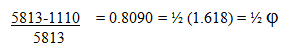It would appear that the intersection of the Ascending Passage and the Entrance Passage is in a Phi (Φ) relationship with the External measurement of the Great Pyramid’s height.

## 38King's Chamber Coffer

At this point we may turn our attention to the contents of the so-called King’s Chamber. In all of the passages and chambers there were reportedly no contents, save for the lidless box, or as it has been suggested, a sarcophagus. It was described by Professor Petrie as follows:

“The coffer in the King's Chamber is of the usual form of the earliest Egyptian sarcophagi, an approximately flat–sided box of red granite. It has the usual under–cut groove to hold the edge of a lid along the inside of the N., E., and S. sides; the W. side being cut away as low as the groove for the lid to slide over it; and having three pin–holes cut in it for the pins to fall into out of similar holes in the lid, when the lid was put on.

It is not finely wrought, and cannot in this respect rival the coffer in the Second Pyramid. On the outer sides the lines of sawing may be plainly seen: horizontal on the N., a small patch horizontal on the E., vertical on the S., and nearly horizontal on the W.; showing that the masons did not hesitate at cutting a slice of granite 90 inches long, and that the jeweled bronze saw must have been probably about 9 feet long. On the N. end. is a place, near the W. side, where the saw was run too deep into the granite, and was backed out again by the masons; but this fresh start they made was still too deep, and two inches lower they backed out a second time, having altogether cut out more than 1/10 inch deeper than they intended. On the E. inside is a portion of a tube drill hole remaining, where they tilted the drill over into the side by not working it vertically. They tried hard to polish away all that part, and took off about 1/10 inch thickness all round it; but still they had to leave the side of the hole 1/10 deep, 3 long, and 1.3 wide; the bottom of it is 8 or 9 below the original top of the coffer. They made a similar error on the N. inside, but of a much less extent. There are traces of horizontal grinding lines on the W. inside." (50)

Professor Petrie measured this box with the utmost attention to detail:

“The coffer was very thoroughly measured, offsets being taken to 388 points on the outside, to 281 points inside, or 669 in all; besides taking 281 caliper measures” The summary of measurements is as follows :

The mean planes to which the coffer surfaces are referred here, and from which the actual surfaces differ by an equal amount + and - , yield the following dimensions: -

 N. End thick Inside length S. End thick Outside length 5.67 78.06 5.89 89.62 E. Side thick Inside width W. Side thick Outside width 5.87 26.81 5.82 38.50 Inner depth Base thick Outer height Ledge depth 34.42 6.89 41.31 1.70

Particular attention should be directed to the mean height, length and width and one should take into consideration that the lid is missing, whereby its completed height needs to be inferred. There is, however, a suggestion of its completed height when one gives thorough consideration to relationships of dimensions found elsewhere in the Great Pyramid which might have been replicated here. The relationship in question is that of the scaling up of the Pyramidion to the size of the Great Pyramid’s external dimensions this being the number 443.1134627 which is the product of the expression 250 vπ. The height of the completed Coffer, could thereby have been 44.311 PI”. The Outer height as given above has a mean dimension of 41.31 B” ( 41.265 PI” ). When one assigns a completed height of 44.311 PI” and you subtract 41.265 PI” then the lid would occupy a measure of 3.046 PI” . At this point consideration should be given to the fact, as stated earlier, that this number 443.1134627 was seen as the rise in the average slope of the internal passages where a slope length has a dimension of 1000. Dividing 1000 by 10, the slope length or hypotenuse would be 100 PI” in the case of the side profile of the Coffer. The slope angle of the hypotenuse in the case of the Coffer would be identical to the average slope angle of the Ascending Passage, the Entrance Passage and Grand Gallery floors. A drawing will illustrate this relationship:Fig. 24

The length dimension resulting from this relationship as shown is 89.647 PI”. When one compares this length dimension with that determined by Prof. Petrie’s investigation where the mean length was found to be 89.62 B” ( 89.52 PI” ) the difference amounts to (89.647 - 89.52 P”)= 0.127 PI” which equates to about 1/8 of an inch or just 3.2 mm . This is certainly well within the tolerances of accuracy of surface texture and finish of the rough sawn exterior. With the dimensions of the height and length herewith determined the next issue before us is a correct determination of the intended width dimension and how this compares to the actual as measured by Professor Petrie. The mean width of the Coffer as determined by Professor Petrie is 38.5 B” (38.458 PI” ).

This measurement, however, may not define the original intended dimension since the Coffer was rough sawn. Nonetheless, when you multiply the width by the height including a lid and then by the length one arrives at the cubic volume as follows:

38.458 PI” x 44.311 PI” x 89.647 PI” = 152,768.568 cubic PI”

To determine the cubic cm volume one multiplies by:

(1.0011 x 2.53999779) 3 = 16.4411579

Therefore the volume of the Coffer is: 152,768.568 x 16.4411579 = 2,511,692 cu. cm.

Consideration can now be given to the intended relevance of this volume and how it would relate to the Earth, in short, whether it has Geodetic significance. As we have seen earlier, the number 43,200,000 was used in the scaling of the Royal Cubit to the Earth and the number 43,200 has been used in the scaling of the Great Pyramid to the Earth. Therefore, it is likely that a factor of 432 would determine a relationship of the Coffer volume with that of the Earth. If you multiply the volume of the Coffer by 4.32 x 1020 then a relationship is found as follows:

2,511,692 x 4.32 x 1020 = 1.08505 x 1027 cubic cm

= 1.08505 x 1012 cubic km

The volume of the Earth as calculated from the Ellipsoid model of WGS 84 is as follows:

Volume of a sphere = radius 3 x π x 4/3

Therefore: Volume of the Earth = 6371.00083 x π x 4/3 = 1.08320733 x 1012 cubic km

In comparing these two figures this amounts to an accuracy of 99.83 %. It should be noted, however, that the measurement of the width of the Coffer is subject to scrutiny as to whether it reflected the intended dimension of the design parameters since it was left in a rough sawn condition. If we review the knowledge exhibited in other features of the Great Pyramid which relate to this enquiry then we shall determine what the design intention might have been. Consideration should be given to the Pyramid’s geometry, which indicated a value for the Mean Circumference of the Earth as 40,030.167 km. To determine the volume of the Earth from this figure one must first calculate the radius as follows:

Circumference / 2π = radius

therefore: 40,030.167 km / 2π = 6370.999 km

Volume calculation is as follows : 6370.9993 x π x 4/3 = 1.0832064 x 1012 cubic km.

= 1.0832064 x 1027 cubic cm

If you divide 1.0832064 x 1027 cubic cm by 4.32 x 1020 you arrive at 2,507,422.2 cubic cm. When you divide by 16.4411579 then you arrive at 152,508.86 cu. PI”. In order to determine the design width of the Coffer one divides this number by the design height of 44.311 PI” and the design length of 89.647 PI” as follows:

(152,508.86 / 44.311)/ 89.647 = 38.3926 PI” which equals 38.435 B”

The difference between Professor Petrie’s determination at 38.5 B” and this value of 38.435 B” amounts to 0.065 B” which is just slightly larger than 1/16 of an inch or 1.68 mm. The overall accuracy of the Coffer is then within an extremely small tolerance in view that it was rough sawn. In summary it would be fair to say that the intended dimensions of the Coffer were as follows:

38.392 PI” wide x 89.647 PI” long x 44.311 PI” high (including the lid) or in British Inches; 38.435 wide x 89.746 long x 44.36 high. which equals 97.6 cm wide x 227.9 cm long x 112.7 cm high.

It is clearly evident that the external volume of the rough sawn Coffer indicates a ratio to the volume of the Earth of 4.32 x 1020 to one.

The following is a schematic drawing of the Coffer including a lid:Fig. 25

## 39Coffer Lid

We may now review the dimensions of the Coffer lid with an interest to see whether its dimensions could have been intended to convey Geodetic knowledge. As stated earlier, the design height of the coffer is suggested to have been 44.311 PI” . The height of the Coffer without the lid as measured by Professor Petrie is 41.31 B” which equates to 41.265 PI”. The space that the missing lid would occupy is then (44.311- 41.265) 3.046 PI”. We should take into consideration a tolerance for a joint between the lid and the Coffer box as well as the fact that the Coffer box is rough sawn. As we have seen in the case of the width dimension a tolerance of 0.066 inches was evident therefore it would be fair to allow a similar tolerance here. In this case we might be correct in saying that the lid might have been 0.066 inches smaller than the space allotted, in which case the lid would have had a design thickness of( 3.046 - 0.066) 2.98 PI”. If this was the case then the design dimensions of the lid would have been:

38.393 PI” wide x 89.647 PI” long x 2.98 PI” thick = 10,256.6 cubic PI”

multiplied by 16.4411579 = 168,630.4 cubic cm

It is to be seen whether this Volume has any relationship with the Pyramidion and in this way impart Geodetic knowledge. The volume of the Pyramidion is as follows:

 therefore which equates to Volume = (Base 2 x Height) / 3 Volume = (52.3983682 x 33.35784) / 3 Volume = 30,528.97 cubic cm

If one divides the lid volume by the Pyramidion volume: 168,630 cu. cm divided by 30,528.97 cubic cm, then one arrives at a ratio of 5.5236 : 1. Presently we use water as a benchmark to make comparisons of density and we have used water at the temperature of 1 degree Celcius to assign a value of 1 cubic cm to equal 1 gram. If we were to have used water at the temperature of 20 degrees Celsius, which is considered normal temperature, then 1 cubic cm would be equivalent to .9982336 grams (52). If we view the figure of 5.5236 as a relationship of density between material and water at 20 degrees Celsius then it would follow that we should multiply this number by .9982336 in order to find a comparison to density that we presently apply in our science. Therefore, 5.5236 x .9982336 = 5.5138 would be a ratio with water at 1 degree Celsius. In terms of mass then, it could be said that the Coffer lid to Pyramidion ratio denotes a density of 5.5138 grams per cubic cm or rounded 5.514 grams per cubic cm. The Mean Density of the Earth is approximately 5.5153 grams per cubic cm (5515.3 kg / cu meter) (53). Although the original lid is not present it is nonetheless conceivable that the intent of its dimensions was to convey this knowledge. If one multiplies this density by the volume of the Earth which was evidently known one shall arrive at the mass of the Earth as follows:

5.5138 g/cc x 1.08321 x1027 cc = 5.9726 x1027 grams or 5.9726 x 1024 kg.

The accepted value for the Mass of the Earth is 5.9742 x 1024 kg . This is an accuracy of approximately 99.98 %.

## 40Coffer Interior Volume

The Coffer interior volume was determined by Professor Petrie to be as described:

From the foregoing data the cubic quantities may be calculated of a simple rectilineal box, omitting all notice of the attachments for the lid, employing the mean planes :–

1.Contents– 72,030; solid bulk = 70,500; volume over all, 142,530 cubic inches. Or by the caliper results, instead of the mean planes, the bulk is 1/580 more, and the contents probably about 1/1000 less; hence the quantities would be :–

Contents = 71,960; solid bulk = 70,630; volume over all, 142,590.

These quantities have a probable error of only about 60 cubic inches on contents and volume, and 100 inches on the bulk. The bulk of the bottom is = 23,830; and hence one side and end is on an average = 23,335. Bulk of bottom x 3 is then = 71,490; and 3/2 x bulk of sides and ends = 70,000, subject to about 100 cubic inches probable error . (54)

It is suggested that the measure of 72,030 would be indicative of the intended size as it is taken from the mean plane measurements of the internal dimensions. The exterior dimensions were also derived from mean plane measurements.

The Coffer volume might be associated with the Royal Cubit or rather, in a cubic form, the Pyramidion that has the Royal Cubit as its base dimension. As stated earlier, the Pyramidion has a volume of 30,528.837 cubic cm . If the coffer volume was equated with an equal volume of water, which is indisputably the most precious resource on the planet, and if its mass is compared to the mass of a Pyramidion made of Gold, which was and remains highly valued for its intrinsic worth, then a unit of measure for volume would have been achieved that associates the worth of water and Gold.

The Volume of the interior of the Coffer as measured by Professor Petrie taken as 72,030 cubic B” ( mean plane measurement method ) equates to 71,793 cubic P” or 1,180,360 cubic cm. If we multiply this volume by the density of pure Water (air free at atmospheric pressure) at 20 degrees Celsius or 68 degrees Fahrenheit,( which is considered normal temperature) and which is .9982336 grams/ cubic cm we arrive at a mass of (1,180,360 x .9982336)= 1,178,275 grams or 1178.275 kg .Now if we take the volume of the Pyramidion which is 30,528.97 cubic cm and multiply this by the density of Gold which is 19.3 grams/ cubic cm we arrive at a mass of 589,209 grams. Since the Pyramidion is representative of a hemisphere we shall multiply this value by 2 in order to arrive at a spherical associated value (589,206 x 2 ) which is 1,178,418 grams or 1178.418 kg. The difference in the mass of Water in the case of the Coffer volume and the mass of the Pyramidon in Gold amounts to 0.143 kg which constitutes an accuracy of 99.99 %. Taken in water this difference represents only 143 cubic cm or 8.7 cu. inches. If the temperature of the measured water were to be a fraction of a degree colder its density would be higher and the relationship would be exact. The accuracy of the Coffer interior is rather quite remarkable. It would be fair to say that the relationship is evident that the interior volume of the Coffer can be equated with the volume of the Pyramidion in a relationship of mass equivalency of Water to Gold.

## 41Queen's Chamber Niche

We shall now return to the interior space of the so-called Queen’s Chamber within which there is a niche in the wall described by Professor Petrie as follows:

The general form of the niche was a recess 41 inches (2 cubits) deep back 62 inches (3 cubits) wide at base, and diminishing its width by four successive over-lappings of the sides (at each wall course), each of ¼ cubit wide, until at 156 high it was only 20 (1cubit) wide, and was finally roofed across at 184 high. Thus, of the 3 cubits width of the base, one cubit was absorbed on each side by the overlappings, leaving one cubit width at the top. This cubit is the regular cubit of 20.6 inches, and there is no evidence of a cubit of 25 inches here. The exact dimensions of every part are as follow, giving the mean dimensions, and the variations of each part, + or –, from the mean. All corrected for the salt exudation on the two lower laps, as estimated at each point; there is no salt on the upper three laps :—(55).

Professor Petrie goes on to give a most accurate detail of the measurements in which he subscribes a height dimension for the roof of this niche above the floor level of 183.8 B”. In and of itself this measurement may not appear to have any relevance, however when one multiplies this dimension by the so-called Queen’s Chamber’s Code Number of 2400 its relevance becomes clear.

183.8 B” x 2400 = 441,120 B”
441,120 x 2.53999779 = 1,120,443.8 cm
1,120,443.8 cm = 11.204438 km rounded = 11.2 km

The Escape Velocity from Earth is approximately 11.2 km / second (56)

The Ancient Egyptians are known to have used the same Second of Time as we use today.

Escape velocity is defined to be the minimum velocity an object must have in order to escape the gravitational field of the earth, that is, to obtain an open orbit. The object must have greater energy than its gravitational binding energy to escape the earth's gravitational field. Therefore :

1/2 mv 2 = GMm/R

Where m is the mass of the object, M is the mass of the earth, G is the Gravitational Constant, R is the radius of the Earth, g is the mean acceleration due to gravity on the Earth’s surface and v is the escape velocity. (the value of g varies dependant on the exact location on Earth)

This simplifies to:

v = √(2GM/R)
or v = √(2gR)
v = √(2 x 9.8066 x 6371008)
v = 11.18 km / sec rounded = 11.2 km / sec

Clearly a considerable amount of knowledge is essential in order to calculate Escape Velocity to the level of accuracy that the Niche exhibits. This corroborates the findings that were earlier proposed that the Mass of the Earth and the radius of the Earth were known to an exceptionally high degree of accuracy. It is herewith evident that either the Gravitational Constant and/or the Acceleration due to Gravity was known. We shall find further evidence of this contention.

## 42Queen's Chamber Length

Another measurement of the Queen’s Chamber should also be examined as it demonstrates a further understanding of Newtonian Mechanics in that its value relates to the Earth’s and the Sun’s gravitational attraction. The measurement in question is the length of the chamber as measured from the East to West walls, which is the length of the apex roof panels. This measurement is 226.47 B” (226.22 PI”). When this value is multiplied by the Code Number of the Queen’s Chamber it amounts to (226.47 x 2400) = 543,528 B” and converted to metric this amounts to (226.47 x 2.53999779) =1,380,559.9 cm or 13.8056 km.

The Escape Velocity from Earth to escape the solar system, that is to say, escape the combined force of the Earth’s and the Sun’s gravitational field in the direction of the Earth’s orbit which is East to West (relative to the Sun) is 13.807 km per second.

An equation demonstrates the relationship as follows: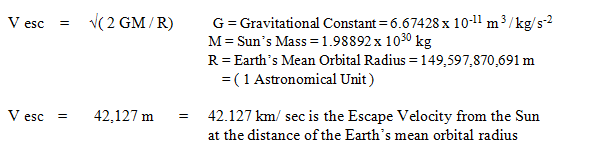In order to calculate the combined escape velocities of the Sun and the Earth’s gravitational field one uses the following equation: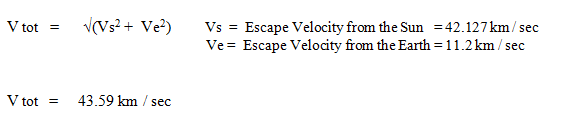Therefore, in view that the Earth is traveling in its orbit about the Sun at a velocity of approximately 29.783 km per second one can subtract this from the Total Velocity value if one sets out in the direction of the Earth’s Orbit which is from East to West.

43.59 km / sec
-29.783 km / sec
13.807 km / sec

It should be appreciated that the Orbital Velocity of the Earth was given in the Bridging Slab which was in place at the Entrance to the Horizontal Passage leading to the Queen’s Chamber. As stated, the length of the Queen’s Chamber from East to West multiplied by the Queen’s Chamber’s Code Number (2400) is 13.8056 km.

Therefore, it is clearly evident that the dimensions found within the Queen’s Chamber suggests an accurate knowledge of the Gravitational Constant and Newtonian Mechanics since there exist two distinct measurements which indisputably demonstrate a knowledge of the Escape Velocities from Earth and a measurement which incorporates the mean Orbital distance to the Sun. Given that it was known how many days it takes for the Earth to orbit the Sun, the Orbital Velocity could be calculated. It is important to note that it is essential to have known the Mass of the Earth and the Mass of the Sun as well as the distance to the Sun to a high degree of accuracy in order to have arrived at these values.

## 43Gravitational Constant

A definition for the Gravitational Constant is as follows:

"The Gravitational Constant, denoted G, is a physical constant involved in the calculation of the gravitational attraction between objects with mass. It appears in Newton’s Law of Universal Gravitation and in Einstein’s theory of General Relativity. It is also known as the “Universal Gravitational Constant”, “Newton’s Constant” and colloquially “Big G”. It should not be confused with “little g”, which is the local acceleration due to gravity, especially that at the Earth’s surface.

According to the Law of Universal Gravitation, the attractive Force (F) between two bodies is proportional to the product of their masses (m1 and m2), and inversely proportional to the square of the distance (r) between them: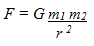The constant of proportionality, G , is the Gravitational Constant."(57)

"The official CODATA value for G in 1986 was given as G = (6.67259±0.00085)x10-11 m3Kg-1s-2 and was based on the Luther and Towler determination in 1982. However, the value of G has been recently called into question by new measurements from respected research teams in Germany, New Zealand, and Russia in order to try to settle this issue. The new values using the best laboratory equipment to-date disagreed wildly to the point that many are doubting about the constancy of this parameter and some are even postulating entirely new forces to explain these gravitational anomalies. For example, in 1996, a team from the German Institute of Standards led by W. Michaelis obtained a value for G that is 0.6% higher than the accepted value; another group from the University of Wuppertal in Germany led by Heinrich Meyer found a value that is 0.06% lower, and in 1995, Mark Fitzgerald and collaborators at Measurement Standards Laboratory of New Zealand measured a value that is 0.13% lower. The Russian group found a curious space and time variation of G of up to +0.7%. In the early 1980s, Frank Stacey and his colleagues measured G in deep mines and bore holes in Australia. Their value was about 1% higher than currently accepted. In 1986 Ephrain Fischbach, at the University of Washington, Seattle, claimed that laboratory tests also showed a slight deviation from Newton's law of gravity, consistent with the Australian results. As it may be seen from the Cavendish conference data, the results of the major 7 groups may agree with each other only on the level 10-1%. So, despite our great technology advancements in measuring equipment, we are still very close to the precision of 1% obtained by Cavendish in the 17th century. This controversy has spurred several efforts to make a more reliable measurement of G, but till now we only got further conflicting results."(58)

The 2006 Codata recommended value for the Gravitational Constant is:

G = 6.67428 x 10-11 m3 / kg/ sec2 ,+ - .00067

Since this measure is contingent on the metric usage of Meters and Kilograms we would need to convert this equation into a form using units of measure which would likely have been used by the builders of the Pyramid. These units of measure would likely have been the Royal Cubit and the Pyramidion Mass. The Royal Cubit (RC) has a measurement, as noted earlier, of 20.60662964 PI” or 52.3983685 cm. It should be noted that 1 meter is equivalent to 1.908456 Royal Cubits. The Pyramidion in Gold (which measures 1 RC at its base) would have a mass of 589.2 kg. This Mass was used in the earlier calculation concerning the equivalency of the mass of a Pyramidion and the interior volume of the Coffer regarding its equivalency in mass of water.

To convert the metric value of the Gravitational Constant (G) equation one must multiply the value by the equivalencies of volume and mass using the Royal Cubit and Pyramidion Mass as follows:

6.67428 x 10-11 x 1.9084563 x 589.2 = 2.73346 x 10-7

Therefore the equation using the Royal Cubit and Pyramidion Mass would be as follows:

2.73346 x 10-7 RC3 / Pyramidion Mass / second2

At this point we may examine this value of 2.73346 x 10-7 and determine whether it is given as a value within the Pyramid’s geometry.

We have seen that the Queen's Chamber and the Passage to it has provided significant measurements relating to the Earth's Orbit of the Sun which is directly related to Gravity and we have seen measurements that relate to Escape Velocities from the Earth which are also contingent on an understanding of the Force of Gravity. If a measure for the Gravitational Constant is to be found as a numerical value then in all likelihood it is to be found in a principal measure of the Queen's Chamber and Passage.

The measure of the distance from the beginning of the Passage to the Queen's Chamber at the Grand Gallery to the center of the Queen's Chamber which is, incidentally, also the lateral center of the Pyramid, was measured by Professor Petrie in which he found it to be 1626.8 B" (British Inches). This measure is equivalent to 1625.0 PI" (Pyramid Inches). If one divides 1625.0 by the Base Perimeter (36524.3 in Pyramid Inches) as well as the Height of the Pyramid (5813.023 in Pyramid Inches) one finds a value of
7.6536645 x 10-6 :

(1625.0 / 36524.3) / 5813.023 = 7.6536645 x 10-6

Furthermore, as we have seen extensively in the calculations involving internal measurements of the Great Pyramid, the "Code" Number of the Queen's Chamber, which is 2400, should be applied. Also, in this case, the Associated Value of the Ante Chamber, which is 400 as was seen earlier, may be applied as follows:

7.6536645 x 10-6 / (2400 +400) = 2.73345 x 10-9

We have seen that the factor of 100 has been applied in numerous cases before therefore, one may apply it here as well, namely:

2.73345 x 10-9 x 100 = 2.73345 x 10-7

The value of the Gravitational Constant using as units the Royal Cubit and Pyramidion Mass was 2.73346 x 10-7 RC3 / Pyramidion Mass / second2 as was stated earlier and the value derived from the Passage to the Queen's Chamber as given is 2.73345 x 10-7 . This accounts for a variance of only 0.000366 %. It may therefore be taken as evident that the value of the Gravitational Constant was imbedded in the dimension of the Passage into the Queen's Chamber and thereby compliment the other substantive measures.

## 44Chambers Above the King's Chamber

The chambers above the King’s Chamber, of which there are five, are difficult to access and were first rediscovered in the late 1800's. Access was gained by blasting through a passage at the very top of the Grand Gallery. The number of chambers is perhaps symbolic, however the overall height we shall see points to astronomical knowledge. From the ceiling of the King’s Chamber to the Apex of the roof of the uppermost chamber a measurement of 1519 cm (598 B”) has been recorded (59). Howard Vyse recorded a measure of 1520 cm for this distance in 1840. (60) It is important to note that the ceiling of the King’s Chamber is accurate to within a tolerance of + ,- 1.6 B” due to subsidence and in part workmanship tolerances. In this case one could reasonably add 1.6 B” to the value of 598 B” to arrive at the maximum value of 599.6 B” which could very well have been commensurate with the original design parameter. The floor of the King’s Chamber was not finished to a smooth and uniform level therefore it may have been intentional that a relevant measurement should rather be taken from the center of the chamber to the apex of the uppermost chamber. In this case we need to calculate the mid-point of the King’s Chamber. As noted earlier, the height of the chamber was determined to be 11.18 Royal Cubits, which is equivalent to 230.38 PI” or 230.635 B”. The mid-point would therefore be (230.38 / 2) =115.19 PI” or 115.32 B”. If one adds this value to 599.6 B” one arrives at a measurement of (115.32+599.6) = 714.92 B”. As we have seen earlier this value should be multiplied by the Code Number of the Queen’s Chamber therefore we arrive at (714.92 x 2400) =1,715,808 B”. This value equates to 4,358,148 cm or 43.58 km.

As noted earlier, the Escape velocity from Earth to achieve escape from the combined Gravitational Field of the Sun and the Earth is 43.59 km per second. This finding further corroborates the values which were found elsewhere in the Geometry of the Great Pyramid and is perhaps the most important value of all given that it demonstrates at once a comprehensive knowledge of the Earth and the Sun. It demonstrates a highly accurate knowledge of the Gravitational Constant, The Mass of the Earth, the Mean Radius of the Earth, the Mass of the Sun and the Earth’s mean Orbital Radius or distance from the Sun. Furthermore, it most certainly demonstrates a knowledge of Newtonian Mechanics.

## 45Equation for The Speed of Light

Consideration may now be given to the elevation of the King’s Chamber in its relation to the pavement level surrounding the base of the Great Pyramid. As stated earlier, the level of the passage that leads to the Chamber has an elevation of 1692 B” (1690.14 PI” ) above the pavement level. This elevation was used earlier in the calculation to determine the effective height of the chamber walls. It cannot be over emphasized that this elevation was not randomly chosen but rather, it is a fundamental element of the design criterion. If you multiply 1690.14 PI” times the Code Number of the Queen’s Chamber which is 2400 you arrive at a value of 4,056,336. Now if you multiply this value by the height of the Pyramid which was calculated to have been 5813.0229 PI” ( 36524.3/π ) you arrive at 2.3579574 x 1010 PI”. If you multiply this figure by the scale of the Great Pyramid to the Earth which is 43,200 you arrive at 1.0186376 x 1015 PI” which equates to 2.5901833 x 1010 km. The distance light travels in a vacuum or space in a period of one day is equal to 2.590206837 x 1010 km .If you divide the figure as given by the Pyramid's dimensions, which is 2.5901833 x 1010 km by 86,400 ( seconds per day ) you arrive at 299,789.7 km . The accepted value for the speed of light in a vacuum is 299,792.458 km/ sec.(61) The speed of light in air is notably slower.

The calculation based on the elevation of the floor level leading into the King’s Chamber amounts to an accuracy of 99.999 % .If the elevation of the passage was .0154 B” or 0.39 mm higher than the calculation would be 100 % accurate.

In addition to this relationship there exists another which should be given due consideration, namely the elevation of the Horizontal Passage leading into the Subterranean Chamber. The elevation of the floor of this passage below the pavement level surrounding the Great Pyramid is -1181 ,+ - 1, British Inches as measured by Professor Flinders Petrie. Converted to metric, this is equivalent to 2,999.7 cm. + - 2.54 cm. . Multiply this value by 10,000,000 and one arrives at a distance of 299,970 km + - 254 km. It is conceivable, thereby, that the elevation of the Subterranean Passage Floor was purposefully designed to be equivalent to the distance light travels in 1 /10 Millionth of a second to within an accuracy of 99.94 %. + - .08%.

## 46Girdle Stones in Ascending Passage

Within the Ascending Passage starting at approximately half way up there exist “Girdle Stones” as can be seen in the following schematic drawing: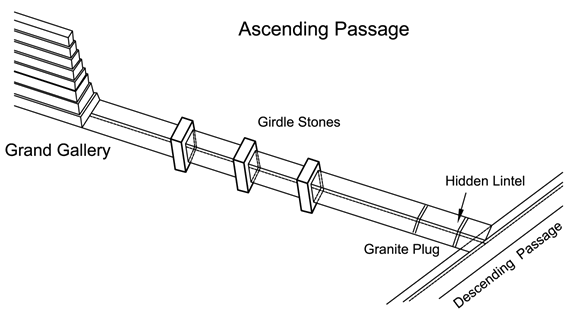Fig. 26

The measurements from the beginning of the Ascending Passage as well as the measurements between these Girdle Stones are worthy of investigation.

The measurements are indicated as follows:

Girdle Stone # 1 Distance from beginning of Ascending Passage      656.5 PI” to 688.5 PI”

Girdle Stone # 2 Distance from beginning of Ascending Passage      861.5 PI” to 894.5 PI”

Girdle Stone # 3 Distance from beginning of Ascending Passage      1069.5 PI” to 1101.5 PI”

The first point of interest is to review the total span from the beginning of Girdle Stone # 1 to the end of Girdle Stone # 3 (1101.5 - 656.5) which is a distance of 445 PI”. If one subtracts this distance from the total length of the Ascending Passage: 1485 - 445 one arrives at a value of 1040 Pyramid Inches. Multiply this distance by the Code Number of the Queen’s Chamber (2400) and one has a distance of 2,496,000 Pyramid Inches which is equivalent to 6,346,808 cm or 63.4681 km. Add this distance to the Reference Circumference of 40,121.434 (the base of the Great Pyramid x 43,200 ) as follows: 40,121.434 + 63.468 = 40,184.902 km. It should be noted that at the Equator the Earth’s present rotational velocity is .4651016 km per second (40,075.017 km (Equatorial Circumference)/ 86164 seconds (1 Sidereal Day)) and therefore, in one Solar day, with 86,400 seconds ( 86,400 x .4651016 ), the Earth rotates 40,184.781 km. at the Equator relative to the stellar background. The difference between the measure of 40,184.902, as given by the Girdle Stones, and the rotational distance at the Equator in one Solar Day amounts to 0.121 km. The Earth rotates this amount of difference in 0.265 Seconds.

In this respect it may be said that the accuracy of the clocks which must have been used and the accuracy of observation had an extremely small margin of error.

The next item to be given consideration is the distances between Girdle Stones. The Center of Girdle Stone # 2 is at 878 PI” from the beginning of the Ascending Passage and the End of Girdle Stone # 1 is at 688.5 PI”, therefore the distance is (878 - 688.5) 189.5 PI” . Multiply this distance by the Queen’s Chamber Associated Value of 2400 and one arrives at a distance of 454,800 PI” which equates to 11.5646 km. Given that the Earth rotated at a rate of .465102364 km. at the Equator per second this would constitute 24.9 seconds of rotation. The distance between the Center of Girdle Stone # 2 and the beginning of Girdle Stone # 3 is 191.5 PI”. which when multiplied by 2400 gives a distance of 459,600 PI” the equivalent of which is 11.6867 km. This distance would amount to 25.1 seconds of rotation. In order to understand the significance of this time duration one must understand that due to the change in speed of the Earth in its orbit about the Sun and due to the Axial Tilt of the Earth relative to the Ecliptic Plane, the Solar day varies in its length. The variance between a Solar clock and for instance a Water Clock or Mechanical Clock is approximately 22 seconds shorter at one time of the Year and approximately 29 seconds longer on a given day at another time of the Year. The average between these absolute values is 25.5 Seconds. This variance accounts for what is called the Equation of Time whereby the accumulation from one day to the next accounts for approximately 16 minutes early and a little over 14 minutes late at different times of the Year dependant on the orientation of the Earth’s Axis and the point of Aphelion (the point that the Earth is furthest from the Sun) and Perihelion (the point that the Earth is closest to the Sun).(62) It should be understood that due to Precession of the Earth’s Axis as well as the Advance of the Aphelion and Perihelion the calculation for the exact Equation of Time for a period 4560 years ago would require an enormous amount of calculations. It is sufficient to summarize in saying that the approximation of 25 Seconds variance greater or lesser than a Mean Solar Day of 86400 Seconds is a quite accurate observation of the length of a Solar day at different times of the Year. The Girdle Stone measurements appear to verify the contention that the builders of the Pyramid were aware of this variance in the length of the Solar Day, in short, they were aware of the Equation of Time.

The third item to be given consideration involves the distance of Girdle Stone # 3 from the beginning of the Ascending Passage. As per the above indicated measurements, Girdle Stone # 3 starts at 1069.5 PI” from the beginning of the Ascending Passage and ends at 1101.5 PI”. The length of the Girdle Stone is therefore 32 PI” At this point we may give consideration to the fact that the Great Pyramid’s location was relational to specific points of interest in the shape of a spheroid; namely 30 degrees and 60 degrees which indicate the Latitudes that bisect the Semi-minor and Semi-Major axis respectively. The Arctangent of (1/√3) is 30 degrees and the Arctangent of √3 is 60 degrees. If you multiply 32 PI” by 1/√3 you arrive at 18.48 PI”. If one adds this value to the beginning of Girdle Stone # 3 namely; 1069.5 + 18.48 one arrives at distance of 1087.98 Pyramid Inches. The total length of the Ascending Passage as measured by Professor Petrie is 1486.7 British Inches which is equivalent to 1485 Pyramid Inches.

When one subtracts the distance of Girdle Stone # 3 from the total length of the passage, namely 1485 - 1087.98 this equals 397.02 Pyramid Inches. As we have seen throughout the upper passage ways and chambers, this measure may be multiplied by the Queen’s Chamber Code Number 2400 whereby we arrive at a measure of 952,848 Pyramid Inches. This is equivalent to 2,422,894 cm or 24.2289 km . We may now subtract this value from the Reference Circumference of 40,121.434 km arriving at a measure of 40,097.2051 km . The radius of such a circumference would be 6381.6684 km.

At this point it is of interest to determine the Axial Tilt of the Earth that would have been evident 4560 years ago since the Axial Tilt of the Earth varies over a period of 41,000 years from approximately 22.1 degrees to 24.5 degrees. In order to determine the Axial tilt for the period in which the Pyramid was built we may apply an equation developed by Lieske in 1976 which is as follows:

E = 84,381.448 - 46.84024T - (59 x 10 -5 )T2 + (1,813 x 10-6)T3 Arcseconds

Where T is in Julian Centuries since ephemeris epoch of 2000

The Axial Tilt of the Earth at present as calculated by Lieske in 1976 and which was adopted by the International Astronomical Union in 2000 is accepted as being 23.43929 degrees relative to the Ecliptic Plane.(63)

In view that the Great Pyramid was built 45.6 Centuries ago, the Axis Angle relative to the Plane of the Ecliptic is calculated to have been 23.9845 degrees.

The Transverse Radius of Curvature at 23.9845 Degrees Geodetic Latitude is 6381.6675 km. As we have seen, the Transverse Radius of Curvature as given by Girdle Stone # 3 is 6381.6684 km. constituting a difference of 0.0009 km or 0.9 Meters. The level of precision in this feature is comparable to other measures that we have seen throughout the Great Pyramid.

It is obvious that the builders intended that the Girdle Stones would convey knowledge of the rotational velocity of the Earth at the Equator by indicating an extremely accurate distance that the Earth rotates in one Solar Day at the Equator as well as indicate the variance in the length of day from one extreme period in its orbit to the other. In short, the Equation of Time was known. Furthermore, a very accurate Geodetic Latitude is conveyed that directly relates to the Plane of the Ecliptic and Axial Tilt of the Earth.

## 47Entrance Passage

The next item of interest pertains to the Entrance Passage of the Great Pyramid, particularly its length from the entrance to its end where the roof line flattens out as it proceeds into the Horizontal Passage into the Subterranean Chamber. This measurement as given by Professor Petrie is 4143 B” (64). Due to the condition of the passage as well as having to allow for the casing stones which are no longer present, the margin of error is stated to be + - 2 B” . We shall nonetheless use his figure of 4143 B”, which equates to 4138.45 PI”, as an approximation of its intended length. If you multiply 4138.45 by 100 (sum of the vertical and transverse height of the passage taken as a numerical value) you arrive at 413,845 which is suggested as its intended value. It should be noted that the Entrance Passage is isolated from the other passages by the Granite Plug in the Ascending Passage which would symbolically preclude using a calculation involving the Queen’s Chamber value. If you multiply 413,845, taken as a numerical value, by the base perimeter of the Great Pyramid (413,845 x 36,524.3 PI”) you arrive at a value of 1.511539893x 1010 PI” which converted to metric equates to 3.8435312 x 1010 cm or 384,353 km .The mean distance to the moon is 384,399 km. (Approx. 363,104 km at Perigee, or closest approach and 405,696 km at Apogee or its farthest point ) (65). The difference between 384,353 km and 384,399 km amounts to 46 km or an accuracy of approximately 99.99 %.In order to have arrived at a mean value of 384,353 km, it must have been known that the orbit of the Moon is elliptical and not a perfect circle.

It is important to note that the value for the Apogee and Perigee are only approximations since these distances do vary considerably from one cycle to another, therefore multiple observations of the Moon are required to determine Mean values. It demonstrates again an understanding of advanced mathematics and advanced observational capabilities. It should be noted that if the Entrance Passage was to be .53 B” longer, then the value would be exact. Furthermore, it should be noted that the Entrance doorway is located on the 19th course of masonry and that the number 19 has been associated with the Moon due to its 19 year Metonic cycle. Which is to say, that the Moon returns to the same relative position with the Earth which in turn is in the same relative position to the Sun every 19 years. Further proof that the Entrance passage indicates an association with the Moon is given by the elevation of the passage mid-line. The Entrance Passage aligns with the 19th course of masonry and the bottom of the course, as measured by Professor Flinders Petrie, has an elevation of 668.3 B” which equates to 667.57 PI” above the pavement surface that surrounds the base of the Pyramid (66). The Vertical height of the Entrance Passage is 52.78 PI”.(67) The Mid-line of the passage would be half of the vertical height which would therefore be (52.78 / 2) = 26.39 PI”. If you add 26.39 PI” to the elevation of the bottom of the 19th Course which is 667.57 PI" you arrive at 693.96 PI". Multiply this value by 10 and you arrive at 6,939.6 which is in fact the number of days in the Metonic Cycle. The Metonic cycle of the Moon relates to a period of 235 complete orbits around the Earth and since each Synodic Orbit (Synodic Month) takes 29.5306 days you arrive at (235 x 29.5306) 6939.69 days. This period of time equates to 19 years within a couple of minutes (19 x 365.2422) = 6939.6 days. Clearly, from the evidence available it can be maintained that the Entrance Passage is associated with the Moon and that the Mean Distance to the Moon was known to a very high degree of accuracy. And furthermore, the elevation of the Entrance Passage points to knowledge of the Metonic Cycle of the Moon.

## 48Scored Line in Entrance Passage

Within the Entrance Passage there exists a scored line at a point 481.6 B” from the Entrance or ( 4143 - 481.6 ) = 3661.4 B” or 3657.38 PI” from the furthest end of the passage.(68) If one multiplies this value by 100 ( the sum of the Vertical and Transverse height of the Passage in PI” (Pyramid Inches) taken as a numerical value) and then by the Base Perimeter of the Pyramid : (3657.38 x 100 x 36,524.3) the product is 1.335832443 x1010 PI” or 3.396743767 x 1010 cm which equates to 339,674 km. It is of considerable interest to note that the Lagrange Point between the Earth and the Moon when the Moon is at its Mean Apogee of 405,700 km is 339,538 km distance from the Barycenter of the Earth-Moon center of Mass. This value is determined using the most current mass estimates for the Earth ( 5.9742 x 1024 kg) (69) and the Moon ( 7.3477 x 1020 kg). (70) If one uses approximations of the masses with the Earth at 5.97 x 10 24 kg and the Moon at 7.3 x 1020 kg the Lagrange Point between the Earth and the Moon is calculated to be at 339,677 km from the Barycenter. The value is in principle, exactly the same as the value derived from the Entrance Passage Scored Line.

The Lagrange Points, also known as Libration Points are positions in space,( in this case in the proximity of the Earth and the Moon ), where a small body of negligible mass affected by the gravity of the larger bodies can theoretically remain stationary relative to the large bodies. Lagrange Points are located where the combined gravitational attraction of the large bodies provide the precise centripetal force required for the small body to rotate in an orbit with them at a fixed distance from their center of Mass, also called their Barycenter, around which the large bodies rotate.

It is of interest to mention that the homographic solutions of the 3 Body Problem were solved by Lagrange and Euler in the 18th Century. These are the solutions for which the configuration of the 3 bodies remain in the same proportions over time. Leonhard Euler found all collinear homographic solutions for the 3 body problem. If M1, M2 and M3 are the masses of the 3 bodies as shown below and r12 and r23 are the distances between bodies 1 and 2 and bodies 2 and 3 respectively, then there is precisely one solution for X = r12 / r23 that gives rise to a homographic solution. Since there are 3 ways of arranging the 3 bodies on a line, clearly there are 3 collinear homographic solutions. In particular, if one of the 3 bodies has a negligible mass, then its location on the line relative to the other two is called a Lagrange Point. This means that in the case of the Earth -Moon system, there are 3 collinear Lagrange Points called L1, L2 and L3 as seen in Fig. 27 .

The following equation determines the co-linear proportional positions of the 3 bodies: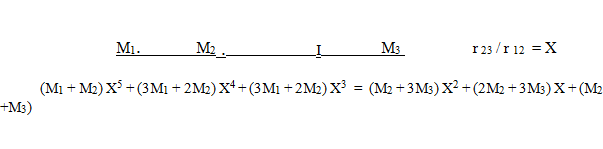In view that the Entrance Passage's scored line indicates the Lagrange Point between the Earth and the Moon, it is suggested that this equation was known in ancient times.

In addition to collinear Lagrange points, of which there are 3 possibilities (shown as L1, L2 and L3 in the following diagram ), it is of interest to note that there exist an additional 2 points that lie in an equilateral triangle configuration with the principal masses. These are seen in the following drawing as points L4 and L5.

Therefore there are a total of 5 Lagrange Points for a body of negligible mass to remain in a stationary orbit with 2 substantial masses as are indicated as L1 to L5 in the following diagram: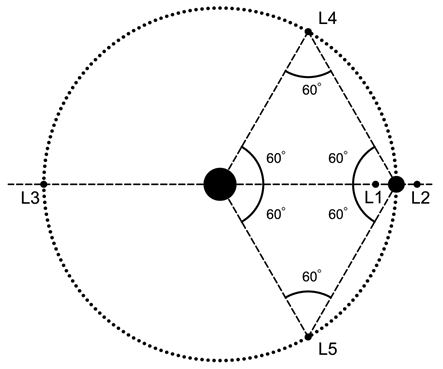Fig. 27

The drawing above is a schematic drawing only as the specific proportions of the Lagrange Points relative to the 2 principal masses is dependent on the relative masses of the principal bodies.

As seen above there exists only one Lagrange point (L1) that falls between the two bodies. It is suggested that this point is indicated by the scored line in the Entrance Passage.

## 49Rock Transition Points in Entrance Passage

The next items to be given consideration concern points within the Entrance Passage where the smooth walls of the passage abruptly change to rough rock. These transition points on the ceiling, floor and walls are at different distances from the beginning of the Entrance Passage and were measured by Professor Flinders Petrie whereby he found the following distances: 1318.5 , 1340.1 , 1347.5 and 1350.7 B”.(71) Given that the Entrance Passage has a length of 4143 B”, the distances from the end of the Entrance Passage would therefore be: 2824.5, 2802.9, 2795.5, and 2792.3 B” respectively.

In Pyramid Inches these measurements would be 2821.4, 2799.8, 2792.4 and 2789.2 PI” respectively. As with the measurement of the scored line, we may multiply these values by 100 ( the sum of the Vertical and Transverse height of the Passage in Pyramid Inches), taken as a numerical value and then multiplied by the Base Perimeter of the Pyramid :

2821.5 x 100 x 36524.3 = 1.030533125 x 1010 PI” = 262,043 km.

2799.8 x 100 x 36524.3 = 1.022607351 x 1010 PI” = 260,028 km.

2792.4 x 100 x 36524.3 = 1.019904553 x 1010 PI” = 259,341 km.

2789.2 x 100 x 36524.3 = 1.018735776 x 1010 PI” = 259,043 km.

In relationship to the Earth’s Orbit about the Sun, these measurements shall be found to be ofconsiderable interest.

To understand the significance of these distances we should investigate points in space between the Earth and the Sun which are called “Neutral Points of Gravity”. These are points in space between two Masses, such as the Earth and Sun, where the Gravitational Attraction between the two masses is equal in opposite directions and therefore the point between them may be called a “Neutral Point”. Given that the density of the Earth was known, as we have seen earlier in regard to the Coffer lid relationship to the Pyramidion, and the volume of the Earth was known very accurately, as we have seen, the total Mass of the Earth must have been known quite accurately. Furthermore, the Orbital Velocity of the Earth about the Sun was known, which can be calculated if one knows the mean distance between the Earth and the Sun and one knows the period of time for one complete orbit. The velocity of the Earth in its orbit about the Sun was given by the Bridging Slab and furthermore, the Escape Velocity from the Earth’s Orbit was also known.

We have seen that the Gravitational Constant must also have been known quite accurately.The Velocity of the Earth in its orbit about the Sun was given earlier as 29.783 km / second and given that the period for one orbit is 365.2564 days at 86400 seconds / day, the Orbital Circumference must have been known to be approximately 939,801,795 km. The Radius of this circumference would be 149,574,101 km. which is very close to the known average Radius of the Earth’s Orbit of 149,587,444 km (average of Semi-Minor Axis of 149,576,999.8 and Semi-major Axis of 149,597,887.5 km).

The average distance between the Earth and the Sun depends upon the Aphelion and Perihelion distances since the Earth has an elliptical Orbit around the Sun from one of the Focal Points of the Ellipse. At the point of Aphelion (the furthest point from the Sun) the Earth has a distance of 152.097,701 km from the Sun and at Perihelion (the closest point to the Sun) the Earth has a distance of 147,098,074 km from the Sun. The average between these distances is149,597,887.5 km. (72)

If one is given the Orbital Velocity of the Earth and its distance from the Sun, as well as the value for the Gravitational Constant one can calculate the Mass of the Sun. Since the Gravitational Attraction of the Sun for the Earth is the Centripetal Force causing the Earth’s circular motion around the Sun, we can use the Gravitational Constant to determine the Mass of the Sun.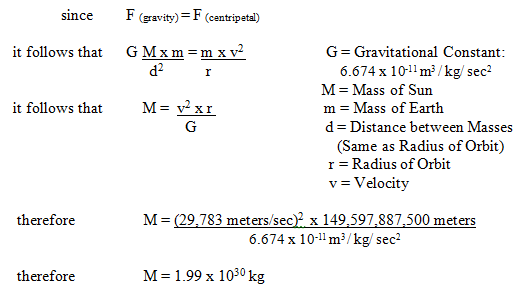Once the Mass of the Sun is determined and the Mass of the Earth is known then one can determine the Neutral Point of Gravity between them.

The relationship is as follows:

 r / R = √( m/M) r = radius to Neutral Point R = Distance between Sun and Earth m = Mass of the Earth M = Mass of the Sun

To solve for the Neutral Point one can rearrange the equation as follows:

r = R x √( m/M)

Or if the Neutral Point is known than one can solve for the overall distance between Massesas follows:

R = r / √( m/M)

Since the Mass of the Earth is approximately 5.97 x 1024 Kg and the Sun’s Mass is approximately 1.99 x 1030 kg the following equation would follow:

√(m/M) = √ (5.97 x 1024 Kg / 1.99 x 1030 kg) = .001732051

At this point we may take the measurements given by the Rock transition points in the Entrance Passage and determine what overall distances between the Earth and the Sun may have beensuggested on the basis that the measurements provided were indeed suggestive of Neutral Points of Gravity between the Sun and the Earth. We may hereby divide the distances by the value of.001732051 and determine the radius measures from the Sun to the Earth as follows: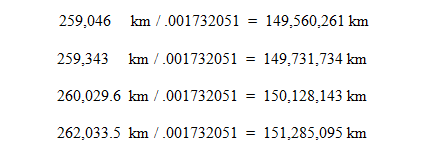These radius distances, as noted, may constitute different points along the elliptical orbit of the Earth around the Sun and in order to determine on which days in the Year they would occur for a period approximately 4560 years ago we need to consider two very important aspects about the Earth’s Orbit.

Firstly, it should be understood that the points of Perihelion and Aphelion shift over time. As was stated earlier in this paper. This can be appreciated by examining the difference between an Anomalistic Year ( the period from one Aphelion or Perihelion to the next ) which has a length of 365.259635864 days and comparing this to the Sidereal Year ( the days to complete one orbit about the Sun) which has a period of 365.256363 days. The difference in the Year lengths is .0032728 days. If we multiply this difference by 4560 years to a period of 2560 BC (the time when the Great Pyramid is said to have been built) we arrive at a figure of approximately 14.9 days. This is to say that the Aphelion and Perihelion occurred approximately 14.9 days earlier than they occur today. This effect is called the Advance of Aphelion/ Perihelion.

The second point to be considered is that the Mean Tropical Year is shorter than the Sidereal Year, whereby there are 365.2421895 days in a Mean Tropical Year as opposed to 365.256363 days in a Sidereal Year. The difference amounts to .0141735 days per year. If one multiplies this difference by 4560 years ( the number of years since the Great Pyramid was built) it amounts to approximately 64.6 days. As stated earlier, this difference is due to the Precession of the Earth’s Axis. In view of these considerations it may be determined, fairly precisely, the days at which the Earth was a specified distance from the Sun as defined by the Neutral Points of Gravity in the period of 2560 BC.

The following diagram indicates the point at which the Earth is at 90 degrees to the Major Axis of the Earth’s Elliptical Orbit: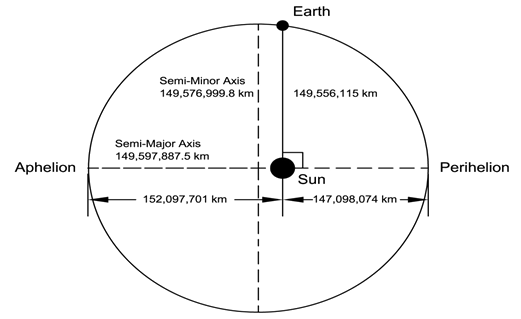Fig. 28 (The eccentricity of the Earth’s Orbit and position of the Sun is not shown to scale

The distance between the Earth and the Sun as indicated in the drawing is 149,556,115 km. One of the distances indicated by the Entrance Passage Rock Transition Points indicates a distance of 149,560,261 km. This amounts to a difference of 0.00277 % . It is interesting to note that the point at which the Earth is 90 degrees to the Major Axis of the Earth’s Orbit may be calculated by using the simple equation of b2/a , where b is the Semi-Minor Axis and a is the Semi-Major Axis.

The term that defines this relationship found in an ellipse is Semi-Latus Rectum. It is quite possible that this equation relating to an ellipse was known and that this value was indicated by the builders of the Great Pyramid by providing the distance to the Neutral Point of Gravity between the Earth and Sun at this particular point. In this way they could make it known that they were knowledgeable about the elliptical orbit of the Earth about the Sun and knew that the Sun is located at one of the Focii Points of the Ellipse. A very precise distance is given.

The second measure indicated by the Entrance Passage Rock Transition Points is calculated to relate to a distance of 149,731,734 km. The following schematic drawing indicates at which point the Earth would be at this distance from the Sun: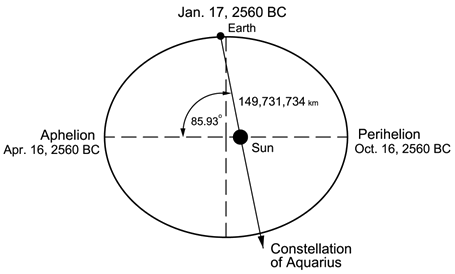Fig. 29 (The eccentricity of the Earth’s Orbit and position of the Sun is not shown to scale)

The date at which the Earth was 149,731,734 km from the Sun 4560 years ago is on or about January 17, 2560 BC . At Sunrise on this day the Constellation of Aquarius was rising at the Eastern Horizon. This occurrence is called a Heliacal Rising of a Constellation. The significance of this shall be reviewed after the other Neutral Points are identified.

The third measure indicated by the Entrance Passage Rock Transition Points is calculated to relate to a distance of 150,128,143 km. The following schematic drawing indicates at which point the Earth would be at this distance from the Sun: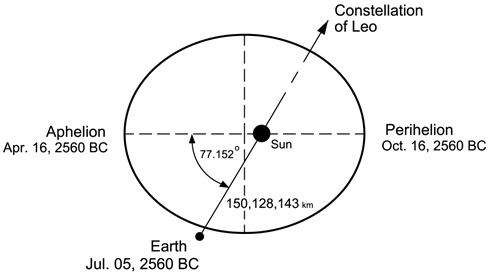Fig. 30 (The eccentricity of the Earth’s Orbit and position of the Sun is not shown to scale)

The date at which the Earth was 150,128,143 km from the Sun 4560 years ago is on or about July 5, 2560 BC . At Sunrise on this day the Constellation of Leo was rising at the Eastern Horizon.

This may be called the Heliacal Rising of Leo. At this point it is of interest to note that when the Constellation of Leo is rising at the Eastern Horizon, the Constellation of Aquarius is descending at the Western Horizon.

The fourth measure indicated by the Entrance Passage Rock Transition Points is calculated to relate to a distance of 151,285,095 km. The following schematic drawing indicates at which point the Earth would be at this distance from the Sun:Fig. 31 (The eccentricity of the Earth’s Orbit and position of the Sun is not shown to scale)

The date at which the Earth was 151,285,095 km from the Sun 4560 years ago is on or about June 4, 2560 BC . At Sunrise on this day the Constellation of Orion was rising at the Eastern Horizon. This may be called the Heliacal Rising of Orion.

At this point we shall examine the significance of the Constellations of Orion, Aquarius and Leo to determine why these particular Constellations would have been singled out amongst all the other Constellations. To appreciate the relationship we need to give consideration to Precession of the Earth’s Axis. During the Age of Leo, which is to say, when the Constellation of Leo has a Heliacal Rising at the Vernal (Spring) Equinox it has been determined that the Constellation of Orion would be found to lie at an Altitude at Meridian of 11 Degrees 8 Minutes of Arc as viewed from the Giza Plateau. It is of considerable importance to note that this is its minimum elevation . This occurred approximately in the period of 10450 BC.(73) Furthermore, in 10,450 BC the Constellation of Aquarius would have had its Heliacal Rising at the time of the Autumnal Equinox, the point directly opposite from the Vernal Equinox in the Earth’s orbit. It has been suggested by Graham Hancock that the Sphinx on the Giza Plateau is the symbolic Leo as it aligns with the Sun facing the Eastern Horizon on the Spring Equinox in the period of approximately 10,500 BC. Graham Hancock suggests that the Sphinx was in fact originally built during this remote period in time. (74) The Geologist Dr. Robert Schock has studied the weathering patterns on the Sphinx and verifies that the Sphinx could very well have been built during a period of heavy rainfall in the very distant past.(75)

At present we are entering the Age of Aquarius where this Constellation will have a Heliacal Rising at the Vernal (Spring) Equinox and Leo will have its Heliacal Rising at the time of the Autumnal Equinox. In 2550 AD the Constellation of Orion shall be found to lie at an Altitude at Meridian of 58 Degrees 11 Minutes of Arc as viewed from the Giza Plateau.(76) This is its maximum elevation. The following schematic drawing ( recreated from a drawing in Robert Bauval and Adrian Gilbert's book “The Orion Mystery”) shows this relationship: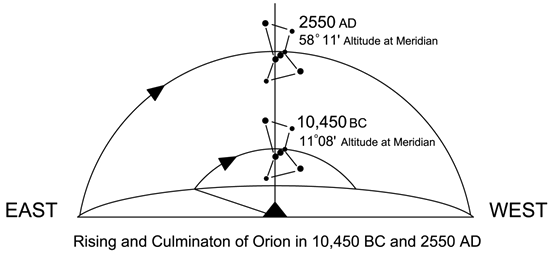Fig. 32

The Authors, Robert Bauval and Adrian Gilbert have studied the relationship between the Great Pyramid, Orion and Leo in their book “The Orion Mystery” and conclude that the builders of the Great Pyramid were well aware of the effect of Precession of the Earth’s Axis over time. The Cosmology of the Ancient Egyptians holds fast to the idea that Orion is associated with their god Osiris. Robert Bauval has substantiated that during the period that the Great Pyramid was built, which he maintains was in approximately 2450 BC, the “Star Shaft” which leads into the King’s Chamber on the South side which has an angle of 45 Degrees would have aligned with one of the principal Stars of Orion’s Belt as it past the Meridian of the Great Pyramid. Furthermore, it is maintained that the three principal Pyramids of the Giza Plateau are aligned to replicate on the ground the positions of the three belt Stars of Orion as seen in the sky. The following diagram shows the alignment of the “Star Shafts” which radiate outwards from the King’s Chamber and the Queen’s Chamber which would have aligned with particular stars at the point at which they would pass the meridian at the time of construction of the Great Pyramid: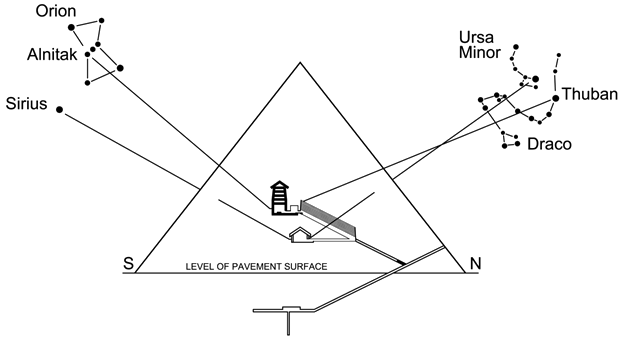Fig. 33

As noted, Orion appeared at its minimum elevation in approximately 10,450 BC and will appear at its maximum elevation in 2550 AD. The period of time between 10450 BC and 2550 AD is 13,000 years which is half of a Great Year of 26,000 years (the period for one complete rotation of the Earth’s Axis due to Precession). In this way it is clearly evident that the view of the Constellation of Orion is linked to the orientation of the Earth’s Axis as it varies due to Precession and that the Constellations of Aquarius and Leo are linked to this relationship. The Constellation of Leo is associated with Horus one of the principal gods of Ancient Egyptian mythology and the Constellation of Aquarius is associated with the Nile River god Hapi. For a complete understanding of the significance of these associations it would be helpful to study the mythology of the Ancient Egyptians thoroughly. For its part this paper is concerned principally with mathematics and Astronomical knowledge.

In view of the foregoing it may be appreciated that the Heliacal Risings of Aquarius, Leo and Orion would have warranted due consideration in the geometry of the Great Pyramid whereby the Neutral Points of Gravity between the Sun and the Earth would substantially indicate the precise points in the Earth’s Orbit where these alignments would occur. From this we can certainly infer that the Rock Transition Points in the Entrance passage were intended to convey a precise knowledge of the Elliptical Orbit of the Earth about the Sun. It is obvious that they had knowledge of the Masses of the Earth and Sun and determined exact distances in the Orbital path of the Earth about the Sun and in so doing determined Neutral Points of Gravity between the Earth and the Sun on specific dates.

## 50Horizontal Passage into Subterranean Chamber

The Passage leading from the Entrance Passage into the Subterranean Passage has a length of 218 B” or 217.76 PI” from the end of the Entrance Passage to the North Door of the Small Chamber as measured by Professor Petrie.(77) If one multiplies this value by the perimeter of the Pyramid; ( 36,524.3 x 217.76 ) = 7,953,531.6 PI” which is equivalent to 20,224,175 cm or 202.24 km. This value if taken as km/second is of special interest. The Solar System revolves around the Galactic Center at approximately 200 km/second. Although estimates vary concerning the distance to the Center between 25,000 to 28,000 light years and the Orbital Period has been estimated as between 225 to 250 Million years, NASA estimates a distance of 27,000 light years to the Galactic Center and a period of 250 million years to complete one orbit.(78) The distance of one light year is 9.46076 x 1012 km and multiplied by 27,000 this equals 2.5544 x 1017 .The circumference of the orbit would be 2.5544 x 1017 km x 2??= 1.60498 x 1018 km and if one divides this by 250 million years then the distance our Solar system orbits the Galactic Center in one year is 6,419,920,488 km. Divide this distance by the number of days in one year and the seconds in one day: ( 6,419,920,488 / 365.2422) / 86400 and one arrives at a value of 203.44 km / sec.

As stated earlier the value indicated by the Horizontal Passage is 202.24 km / second. The accuracy is quite remarkable. If one examines the next item we shall see further evidence that supports the idea that it was known at what speeds our Solar system travels through Space.

## 51Dead-End Passage from Subterranean Chamber

On the opposite end of the Subterranean Chamber there is a Dead-end Passage which measures 646 B” or 645.3 PI” in length from the South Door of the large Chamber to its end.(79) If one multiplies this value, as before, by the circumference of the Great Pyramid ( 645.3 x 36,524.3 PI”) one arrives at a value of 23,569,131 PI” which equals 59,931,392 cm or 599.3 km. Taken as km/ second this value closely matches the speed at which the Local Group of Galaxies including our Milkyway Galaxy is traveling relative to the Stellar background or Universe, which has been estimated by NASA to be approximately 600 km/second.(80) The Milky Way Galaxy is being drawn by the Gravitational pull of what is named the “Great Attractor” which is in the direction of the Virgo Cluster of Galaxies.

As can be seen, there exist two horizontal passages, one leading into the Subterranean Chamber and the other leading out of it, both of which appear to relate to the velocities of the Solar System. The Dead-End Passage in its very nature as being a dead-end suggests a final destination for our Solar System.

It cannot unequivocally be stated that the measurements given in the Subterranean Passages relate to the velocities of the Solar System, however, in view of the exactitude seen in other astronomical, astrophysical and geodetic data it can be suggested that the builders of the Great Pyramid might have incorporated these values in the geometry of the Pyramid.

## 52The 24th Case of Masonry

At this point we shall return to the exterior of the Great Pyramid and investigate two particular aspects. As we have seen, the number 2400 is the "Code Number" which was found imbedded in the dimensions of the "Queens Chamber". We have found that the dimension of a Circle of 2400 Royal Cubits in diameter and there-in its Inscribed Square organizes the entire Giza Plateau Pyramids and Sphinx.

This number was found to unlock the mystery of the dimensions of the Great Pyramid's Passages and Corridors. This number brings forth Geodetic, Astronomical and Astrophysical information concerning the shape of the Earth, Gravity, the Speed of Light and important Astronomical distances relating to the Earth's Orbit, the Sun and the Moon.

We, furthermore, realize that 2400 multiplied by 1800, which we found as the distance in Royal Cubits between the centre of the Great Pyramid and that of Menkaure, is a value of 4,320,000.

We understand that the value of 43,200,000 divided by the factor of 100 gives a value of 43,200 and that the Great Pyramid, representing the Hemisphere of the Earth, is scaled with the Earth in a ratio of 43,200 to One.

We should appreciate that the factor of 100 was used in the dimension correlation of the Base Perimeter of the Great Pyramid as being the number of days in a Winter Solstice Year represented by Pyramid Inches multiplied by 100.

Therefore, we could divide the number 2400 by 100 to arrive at the number 24 and hence the 24th Course of Masonry becomes of particular interest.

With all of this in mind, it would be incumbent to investigate the 24th Course of Masonry of the Great Pyramid to decipher whether there exists corroborative information imbedded in the dimensions of this Course.

From Flinders Petrie's book, The Temples and Pyramids of Gizeh, 1883, Chapter 23, Plate 8, we find that the height of the 24th Course of Masonry is 852.7 British Inches above the Pavement Level on both the North Side as well as the South Side of the Pyramid. Furthermore, we find that the Thickness of the Course is 32.3 British Inches on the North Side and 32.4 British Inches on the South Side, giving a simple average of 32.35 British Inches as reasonably indicative of the builder's intended Course thickness. This would be equivalent to 32.3 Pyramid Inches.

The truly astounding accuracy of this Course of masonry clearly demonstrates unparalleled skill of workmanship and design on the part of the builders to last for a period of thousands upon thousands of years and still retain such exactitude.

At this point, we may analyze whether a play with numbers shall reveal embedded information.

We may start with the number 43,200 as understood to represent the scaling of the Great Pyramid as it relates to the Hemi-sphere of the Earth. Therefore it would be suggested that 43,200 x 2 = 86,400 would relate to the Sphere of the Earth.

Next, we should be cognizant that the Value of Pi (π) (3.1415926...) is imbedded in the symmetry of the Great Pyramid itself and was also found in the symmetry of the layout of the Giza Pyramid placements on the Plateau. Therefore, the value of Pi could be instrumental here.

From this we may devise:

43,200 x 2 / π    =    27,501.974

The Value 27,501.974 can be rounded simply to 27,502.

Next, we can divide 27,502 by the height of the 24th Course of Masonry which was given earlier as 852.7 British Inches which would be equivalent to 851.76 Pyramid Inches rounded to 851.8 PI above the Pavement Surface. The calculation derived is as follows:

27,502 / 851.8    =    32.287    =    rounded to 32.3

We should recall that the thickness of the 24th Course of masonry is 32.3 Pyramid Inches.

Therefore, with simple clarity, we find that the 24th Course of Masonry does indeed appear to contain an imbedded relationship whereby the thickness and elevation equates to a relationship of the scaling of the Great Pyramid and Pi.

Certainly at first, this relationship may appear tenuous, however, in view of the accuracy of the 24th Course dimensions and corroborative logic to be found elsewhere within and without the Great Pyramid and Plateau, it is a plausible argument.

## 53The 36th Case of Masonry

The next course of masonry which could have some relevance to the underlying logic of a relationship of numbers with the overall design imperative is the 36th Course of Masonry.

From Flinders Petrie's Book, Temples and Pyramids of Gizeh, 1883, Chapter 23, Plate 8, we find that the height of the 36th Course of Masonry is 1228.7 British Inches above the Pavement Level on the North Side and 1228.5 British Inches on the South Side of the Pyramid for an average value of 1228.6 British Inches. This would be equivalent to 1227.3 Pyramid Inches. Furthermore, we find that the Thickness of the Course is 41.8 British Inches on the North Side and 41.1 British Inches on the South Side, giving a simple average of 41.45 British Inches as reasonably indicative of the builder's intended Course thickness.

This would be equivalent to 41.40 Pyramid Inches.

We have seen that the number 36 is found as the degrees of an angle found in Phi (Ф) Symmetry in relationship to the Vertices of a Pentagram and most importantly as the Geodetic Angle at which the Transverse Osculating Circle of the Earth is represented in the size of the Perimeter of the Great Pyramid scaled to the Earth in a ratio of 43,200 to One.

Furthermore, it is to be recognized that the Cosine of 36 Degrees is 1/2 Phi, (1/2 Ф); The Golden Ratio of 1.618033989 divided by 2 = .809016994…

In a simple mathematical equation we may find a relationship between the number 24 and the 36th Course of Masonry which involves the value of the Cosine of 36 degrees and the dimensions of the 36th Course itself.

The Course Elevation is 1227.3 Pyramid Inches, thereby we might multiply this value by the Cosine of 36 Degrees (1/2 Phi) as follows:

1227.3 x .809    =    992.9

Next we may divide this value by the number 24 as follows:

992.9 / 24    =    41.37

The thickness of the 36th Course of Masonry was approximated as 41.4 Pyramid Inches.

In view of this simple relationship with the elevation and Phi, it could be reasonably propounded that this could very well have been intentional on the part of the builders as it continues in the same vein of logic as we have seen throughout the Great Pyramid's dimensional attributes.

## 54Key Numbers of The Great Pyramid

Within the geometry of the Great Pyramid we have seen numbers which may be interpreted as“key numbers”. These numbers are as follows:

1.618 = the Golden Ratio
5 = the number of levels of granite beams and hence Chambers above the King’s Chamber
5 = the number of courses of masonry in the walls of the King’s Chamber
7 = the number of laps in the Grand Gallery’s walls
9 = the number of granite roof beams in the ceiling of the King’s Chamber
36 = the Latitude which the Great Pyramid’s Base Perimeter relates to (Osculating Circle)
36 = the number of Roof Tiles on the ceiling of the Grand Gallery
100 = the number of Pyramid Inches in the Vertical and Transverse measure of the Entrance and Ascending Passage height
100 = the number of Pyramid Inches in the diagonal of the Coffer in the King’s Chamber
180 = the Number of Pyramid Inches of the length of the Granite Plug in the Ascending Passage
2400 = the “Code Number” of the Queen’s Chamber
43,200 = the Scale of the Great Pyramid to the Earth
108,000 = the Associated Value of the King’s Chamber

It remains to be seen whether the choice of these numbers were arbitrary or whether the use of these specific numbers were intentional to draw specific attention to a specific geometrical form which would be indicative of advanced mathematics. The Great Pyramid itself is geometrically a polyhedron with 5 faces. It is to be seen whether a combination of the above noted numbers are to be found in the geometry of a single polyhedron which would be significant in a symbolic manner.

The number 5, used repeatedly in the King’s Chamber, is found geometrically in the number of sides of a uniform Pentagon. The Interior Angles found in a Pentagon are each 108 Degrees. It should be noted that the Associated Value of the King’s Chamber is 108,000 (108 x 1000). The External Angles of a Pentagon are each 252 degrees ( 7 x 36 ) as seen in the drawing below. It should be noted that there are 7 Laps in the walls of the Grand Gallery and there are 36 Roof tiles. Furthermore, the Base Perimeter of the Great Pyramid relates to the Transverse Osculating Circle of the Earth at 36 Degrees Geodetic Latitude. This relationship between the exterior of the Great Pyramid and the Grand Gallery may very well have been intentional.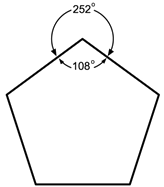Fig. 34

Furthermore, the External Angles found in a Pentagram are each 324 Degrees ( 9 x 36 ). The ceiling of the King’s Chamber consist of 9 Granite Beams . The Interior Angles of each Vertex of a Pentagram are 36 Degrees.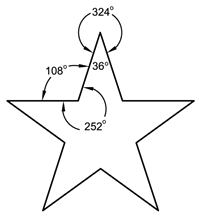Fig. 35

The Pentagram consists of a multitude of line segments which are related to one-another in the Golden Section or Golden Ratio which may be appreciated in viewing the following drawing: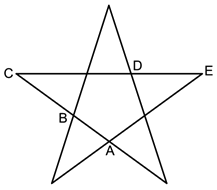Fig. 36

AC = BC = CE = CD = Φ = (1 + √5) = 1.618033989...
BC    AB    CD    DE                2

It is of interest to note that the maximum height of the Grand Gallery is 346 British Inches, which coincides with 345.6 Pyramid Inches. As there are 4 walls in the Grand Gallery we may divide 345.6 by 4 arriving at a value of 86.4 Pyramid Inches. If we divide this number by a factor of 2400 (The Code Number of the Queen’s Chamber) namely 2.4 (2400 / 1000) we arrive at a value of 36 .

Furthermore, 345.6 multiplied by 250 (a factor relating to the scaling of the Pyramidion to the Pyramid) one arrives at 86,400, which is the number of seconds in one Solar Day. This relationship was explored earlier on in this paper.

It appears quite likely that this relationship of numbers was intentionally designed into the Grand Gallery’s dimensions.

In an excerpt from a book written by John Anthony West entitled “Serpent in the Sky:The High Wisdom of Ancient Egypt”, he had this to say about ancient Egyptian usage of 5 foldedness and the Golden Section:

In Ancient Egypt, the symbol for a star was drawn with five points. The ideal of the realized man was to become a star, and “become one of the companyof Ra.”

He goes on to state:

The number of “love”, the number sacred to Pythagoras, the number symbolized by the Pentagon and Pentagram, which commanded the proportions of the Gothic Cathedrals, played a crucial but subtler role in Egypt. Apart from the hieroglyph of the five-pointed star, we find no overt instances of five-sided figures.Instead Schwaller de Lubicz found the square root of Five commanding the proportions of the “Holy of Holies”, the inner sanctuary of the Temple of Luxor.In other instances he found the proportions of certain chambers dictated by the Hexagon generated from the Pentagon. In others, crossed 8 x 11 rectangles,the four sided generators of the Pentagon from the square, commanded the proportions of wall murals symbolically related to those functions represented by Five. Egypt also made extensive use of the Golden Section which from the Primordial Scission, commands the flow of numbers up to Five. The Pentagram made up of Golden Section segments, is the symbol of unremitting activity;Five is the key to the vitality of the Universe, its creative nature.(81)

The symbol of the Five-pointed star was used in Egyptian hieroglyphics in association with the Goddess Sopdet who represented the brightest star Sirius. This star, which appears adjacent tothe constellation of Orion, was seen to have its heliacal rising (seen on the eastern horizon just before dawn) at the time of the annual inundation of the Nile river and thus marked the beginning of the agricultural year. It stood to reason that the Goddess Sopdet was called the “bringer of the New Year and the Nile Flood”.(82) It is of interest to note that the southern “Star Shaft” of the Queen’s Chamber was in alignment with Sirius as it crossed the Meridian at the time when the Great Pyramid was built ( see Fig.33 Page 78) according to Robert Bauval and Adrian Gilbert as detailed in their book “The Orion Mystery: Unlocking the Secrets of the Pyramids”.

At this point we may investigate a Polyhedron that embodies Pentagrams and Pentagons and incorporates the “Key” numbers found in the geometry of the Great Pyramid. The Polyhedron of interest is the Great Dodecadodecahedron which is referred to as Uniform Polyhedron # 36 (U36).Fig.37It is of interest to note that the Central Angle of the Great Dodecadodecahedron, which is to say from any two vertices of the Pentagrams to the center of the polyhedra (Circum. Radii) is 36 Degrees. The Cosine of 36 degrees is none other than ½ the Golden Ratio (.809016994...). The Circum. Radius relative to the distance between vertices is equal to the Golden Ratio (1.618033989...) whereby Tall Golden Isosceles Triangles are generated.

Furthermore, the number of Faces of the Great Dodecadodecahedron, namely 24, is equal to 2400 (Code Number of the Queen’s Chamber) divided by 100. The factor of 100 is seen in the perimeter measure of the Great Pyramid whereby, measured in Pyramid Inches it is the length of the Winter Solstice Year in days multiplied by 100.

If one multiplies the elements of the Great Dodecadocehedron together one arrives at a value of 43,200 , namely; Faces x Edges x Vertices:

24 x 60 x 30 = 43,200

As we have seen, the scale of the Great Pyramid to the Earth is 43,200 to one.

As noted, the Dodecadodecahedron has 12 Faces which are Pentagrams which have 5 Vertices of 36 Degrees each, whereby the sum of the Vertex angles is 180 per Pentagram. It is of interest to note that the Granite Plug in the Ascending Passage is 180 Pyramid Inches. Since there are 12 Pentagram Faces on a Dodecadodecahedron the total sum of the interior angles of the Vertices is 2,160. Furthermore, the Dodecadodecahedron has 12 Pentagon Faces which have 5 vertex angles of 108 degrees each, whereby the sum of interior angles per Pentagram is 540. The total sum of angles for the 12 Pentagrams is 6,480. When one adds the angle sums of the Pentagrams and Pentagons the total sum is 8,640.

It is of interest to note that 8,640 multiplied by 5 is equal to 43,200 which is the scale of the Great Pyramid to the Earth. Furthermore, 8,640 multiplied by 10 is equal to 86,400 which is the number of Seconds in one Solar Day.

Consideration may also be given to the fact that the total sum of all interior and external angles of a Pentagram is 3,600. In view that there are 12 such Stellated Pentagram faces the total sum of all angles of the Pentagram faces is 43,200 degrees.

Another point of interest is that the total sum of all interior and external angles of a Pentagon is 1800 degrees and as there are 12 Pentagon faces on a Dodecadodecahedron the total sum for all faces is 21,600 degrees.

Furthermore, there are 60 central angle vertices in the interior of a Dodecadodecahedron, each of which have 36 degrees as their interior angle, as we have seen earlier, and each of which have external vertex angles of 324 degrees. Since each vertex has a sum of 360 degrees (interior and complimentary external) and since there are 60 such vertices, the sum of all central angle vertices is 21,600 degrees.

If one adds the sum of angles of the 12 Pentagram faces, the sum of angles of the Pentagon faces and the sum of the central angles one arrives at a grand total of 86,400 degrees.

Additionally, each of the 60 triangles that radiate outward from the center to each pair of facial Vertices have interior base angles of 72 Degrees and External Angles of 288 Degrees. Each base has a sum total of 720 degrees and since there are 60 such triangles formed, the total sum of all base angles is 43,200 Degrees.

A further point of interest concerning the Dodecadodecahedron is that there are 24 Faces, 60 Edges and 60 Central Angle Vertices, whereby in multiplying these values together one arrives at 86,400: 24 x 60 x 60 = 86,400

In view that the elements, principle angles and sums of angles which are found within the geometry of the Great Dodecadodecahedron have values which are found as the “Key Numbers” within the geometry of the Great Pyramid, the possibility exists that this relationship may well have been incorporated by design.

It is also to be given consideration, that the choice of 86,400 Seconds in a Solar Day may have originated from a mathematical model of the Great Dodecadodecahedron which is based on the Golden ratio and Icosahedral Symmetry. The Icosadodecahedron, which shares the Vertex arrangement with the Dodecadodecahedron is one of the 13 Archimedian Solids.

It is well known that the Ancient Greeks were very interested in geometrical forms and it would appear that the Ancient Egyptians predated the Greeks in knowledge of this nature. In view of the remarkable degree of Geodetic and Astronomical knowledge evident in the construction of the Great Pyramid, it is very plausible that a geometric form such as the Dodecadodecahedron with its Golden Ratio symmetry would have been an ideal form from which to derive time increments for a Solar Day. It appears that our time is a derivative of the Golden Ratio.

## Conclusion

It is concluded that the Great Pyramid of Giza and the entire Giza Plateau is of a highly intelligent and fully integrated design. Its construction detail demonstrates extraordinary precision in relaying highly accurate geodetic knowledge of the Earth, astronomy, astrophysics, advanced mathematics and Newtonian mechanics. Since there exist numerous examples of complimentary and corroborating values, which may be interpreted as encoded within the measurements of the Great Pyramid's geometry and the specific placement and alignments of the Pyramids and Sphinx on the Giza Plateau, it is evident, with a very high degree of probability, that the design parameters were expressly intent on conveying this advanced knowledge.

We have seen that the overall Giza Plateau structures are arranged within a 2400 Royal Cubit circle and that the "Code Number" found within the dimensions of the Queen's Chamber is the number 2400 which unlocks the relevance of the dimensions of the interior passages and Grand Gallery of the Great Pyramid of Khufu.

Furthermore, we have seen that the distance between the Great Pyramid and the third pyramid of Menkaure is 1800 Royal Cubits. The product of 2400 x 1800 = 4,320,000. The factor of 100 is used in the association of the perimeter of the Great Pyramid with the number of days in a Winter Solstice Year, whereby one might apply this logic in dividing 4,320,000 by 100 to arrive at the value of 43,200. In this consideration, it supports the contention that the Great Pyramid is scaled in relationship to the Earth in a ratio of 43,200 to 1.

We have seen that the shape of the Earth was of a primary consideration particularly in that the Base Perimeter of the Great Pyramid (multiplied by 43,200) pertains to the circumference of the osculating circle of the Earth at 36 degrees latitude. This also reflects the expressed desire to incorporate the Golden Ratio (Phi) . The location of the Great Pyramid conveys an accurate knowledge of the shape and size of the Earth in its mathematical relationship to particularly important points of interest namely; at 30 degrees where the semi-minor axis of the Earth is bisected, at 60 degrees where the semi-major axis of the Earth is bisected, and at 45 degrees exactly geometrically bisecting 90 degrees. Furthermore, the Coffer within the King's Chamber ( multiplied by 4.32 x 1020 ) conveys knowledge of the volume of the Earth, whereas the lid ( as defined by calculation in its ratio with the Pyramidion) relates itself to the average density. The Grand Gallery goes on to define particularly interesting radii of the Earth, most notably, the groove, which runs the length of the Grand Gallery midway between the floor and ceiling, which relates to the geodetic latitude at which the geometric mean radius of curvature of the Earth is identical to the Equatorial radius.

We have seen that the Queen's Chamber and the Passage to it relate to the Earth's orbit of the Sun and considerations related to the force of gravity. Particular emphasis is given to the Escape Velocity from the Earth as this value is encoded within the measure of the Niche, itself a most notable point of interest within the chamber. We have furthermore seen that the orbital circumference of the Earth about the Sun was encoded within the measure of the elevation of the passage into the Queen's Chamber. Neutral points of gravity between the Earth and the Sun are defined by measures taken from within the Entrance Passage. As noted earlier, these neutral points of gravity are indicative of an intent to convey the importance of the Earth's position relative to the Sun at particularly important points in its orbit, namely, when the heliacal risings occur of the constellations of Orion, Leo and Aquarius. These constellations are noteworthy in their associations in the context of Ancient Egyptian Cosmology.

Furthermore, we have seen that the distance to the Moon as well as the Lagrange point between the Earth and the Moon was encoded within the measurements of the Entrance Passage. The Metonic Cycle of the Moon was provided by the measure of the Entrance Passage's elevation above the pavement surface. This further supports the importance given to the Moon in Ancient Egyptian Cosmology.

The Ascending Passage, as we have seen, defines the rotational velocity of the Earth and relays knowledge of the Equation of Time. It also provides an accurate knowledge of the Earth's axial tilt relative to the Plane of the Ecliptic.

We have seen that the number 43,200 relates to the scale of the Great Pyramid's Base Perimeter and the osculating circle of the Earth at 36 degrees latitude. We have also seen the factor of 4.32 x 1020 in the scaling of the King's Chamber Coffer to the volume of the Earth. Furthermore, we have seen that 4.32 x 1010was used as a factor in the calculation relating to the orbital circumference of the Earth about the Sun. In view that the number 43,200 along with other key numbers were used repeatedly in the Great Pyramid's geometry there is a probability that the mathematical model known as the Dodecadodecahedron, with its Golden Ratio proportions could have been influential in the design parameters. It is suggested that the Dodecadodecahedron's principal attributes may have been instrumental in the creation of our 86,400 second day. The recurring use of the number 43,200 is an item of some interest as this number or factors thereof are apparent in other ancient manifestations. It is most interesting that 432,000 years are attributed as the time passage in the Babylonian Flood story and as the length of the Kali-Yuga Time Cycle of the Eastern Religions whereby each successive Yuga adds to the ten-foldedness of the Maha-Yuga of 4,320,000 years. Furthermore 432,000 is equal to the number of guardians of the Germanic Walhalla or Heaven ( viz. 800 for every 540 gates). Incidentally, the total number of degrees of the interior angles of a Pentagon is 540 ( 108 x 5 ).

There are significant similarities in Ancient Mythologies as given to understand in a book by Giorgio de Santillana and Hertha von Deschand entitled “Hamlet’s Mill : An Essay Investigating the Origins of Human Knowledge and Its Transmission Through Myth” in which it is maintained that knowledge of the former civilization was handed down in the form of Myths and Legends. Perhaps a cataclysmic event such as Global flooding, as presented in numerous ancient mythologies, caused a major upset in the advancement of our Civilization and resulted in a collective amnesia. A re-examination of ancient texts with a renewed perspective would undoubtedly augment our knowledge of the past.

The Great Pyramid of Giza and the entire Giza Plateau may clearly be regarded as a repository of ancient knowledge. Perhaps the advanced scientific knowledge possessed by the builders was concealed from public knowledge and confined to the select few in order to avoid disrupting the prevailing religion. In this respect it would have been built for some future rediscovery. At present, we have only a fragmented understanding of the Civilizations that lived in the distant past. It is hopeful that we will find new evidence in the future which will further advance our limited knowledge .In any event we must retain an open mind and not rigidly confine our minds with preconceptions of what could have been know by our Ancestors.

Wandelt sich schnell auch die Welt,
Wie Wolkengestalten,
Alles Vollendete faellt
Heim zum Uralten.

R.M. Rilke, Sonette an Orpheus

So quickly the world doth change
Like shapes in the clouds,
Only the achieved remains
Cradled in timeless antiquity.(83)

• 1. Alan M. Fildes & Dr. Joann Fletcher, Alexander in Egypt,
http://www.touregypt.net/featurestories/alexanderthegreat.htm
• 2. Cooper, L., The Royal Cubit, http://www.sover.net/~re/deep_secrets/cubit/, Page 1
• 3. Petrie, Flinders, W. M., The Temples and Pyramids of Gizeh, 1883,
http://www.touregypt.net/petrie/ ,Chapter 7, Section 52
• 4. Tompkins, Peter, Secrets of The Great Pyramid, Harper & Row Publishers,
New York,1978 ISBN: 0-06-090631-6, Page 31
• 5. Davidson, D. And Aldersmith, H., Great Pyramid its Divine Message, Kessinger Publishing,
ISBN 1-56449-116-6, Page 1
• 6. Hume, K. J. (Kenneth John), A History of Engineering Metrology, London: Mechanical
Engineering Publishing, 1980, Page 26
• 7. Smyth, Piazzi, The Great Pyramid, Its Secrets and Mysteries Revealed,
Bell Publishing Company, New York, 1978, Page 43
• 8. Davidson, D. and Aldersmith, H., Great Pyramid its Divine Message, Kessinger Publishing, ISBN 1-56449-116-6, Page 367
• 9. Tropical Year, http: // en.wikipedia.org / wiki / Tropical_year
• 10. GGFC,Nasa,IERS Special Bureau for tides, http://bowie.gsfc.nasa.gov/ggfc/tides/intro.html
• 11. Tropical Year, http: // en.wikipedia.org /wiki / Tropical_year
• 12. Davidson, D. and Aldersmith, H., Great Pyramid its Divine Message, Kessinger Publishing,
ISBN 1-56449-116-6, Page 117-131
• 13. Technology Through Time: Issue # 4, El Karnak ,
http://sunearthday.nasa.gov/2005/locations/elkarnak.htm
• 14. Ancient Egypt Online, Ra, http: // www. ancientegyptonline.co.uk / ra.html
• 15. Hancock, Graham & Faiia, Santha, Heaven’s Mirror, Quest for the Lost Civilization,
Doubleday Canada, Toronto, Canada,1999, ISBN 0-385-25894-1, Page 55-57
• 16. Technology Through Time: Issue # 4, El Karnak ,
http://sunearthday.nasa.gov/2005/locations/elkarnak.htm
• 17. Brandon, S.G.F., Religion in Ancient History: Studies in Ideas, Men and Events,
George Allen & Unwin Ltd., London, 1973, ISBN 0 04 200020 3, Page 135-136
• 18. Khufu Ship, http: // en.wikipedia.org / wiki / khufu_ship
• 19. Davidson, D. and Aldersmith, H., Great Pyramid: its Divine Message,
Kessinger Publishing, ISBN 1-56449-116-6, Page 198, Fig. C.
• 20. Lemesurier, Peter, The Great Pyramid Decoded, Compton Press Ltd.,Great Britain,
1977,ISBN 0 85955 015 X, Page 66,67
• 21 a.Smyth, Piazzi, The Great Pyramid, Its Secrets and Mysteries Revealed,
Bell Publishing Company, New York, 1978, Plate XX
• 21 b.Petrie, Flinders, W. M., The Temples and Pyramids of Gizeh, 1883,
http://www.touregypt.net/petrie/,Chapter 6,Section 25
• 22. Wikipedia, Pi, http: / / en . Wikipedia . org / wiki / pi
• 23. Wikipedia, Pi, http: / / en . Wikipedia . org / wiki / pi
• 25. Petrie, Flinders, W. M., The Temples and Pyramids of Gizeh, 1883,
http://www.touregypt.net/petrie/ ,Chapter 6, Section 21
• 25. Tompkins, Peter, Secrets of The Great Pyramid, Harper & Row Publishers,
New York,1978, ISBN: 0-06-090631-6, Page 203
• 26. How WGS 84 defines the Earth, http://home.online.no/~sigurdhu/WGS84_Eng.html
• 27. Davidson, D. and Aldersmith, H., Great Pyramid: its Divine Message,
Kessinger Publishing, ISBN 1-56449-116-6, Page 124
• 28. Petrie, Flinders, W. M., The Temples and Pyramids of Gizeh, 1883,
http://www.touregypt.net/petrie/ ,Chapter 7, Section 41
• 29. Petrie, Flinders, W. M., The Temples and Pyramids of Gizeh, 1883,
http://www.touregypt.net/petrie/ ,Chapter 7, Section 48
• 30. Petrie, Flinders, W. M., The Temples and Pyramids of Gizeh, 1883,
http://www.touregypt.net/petrie/ ,Chapter 7, Section 52
• 31. Petrie, Flinders, W. M., The Temples and Pyramids of Gizeh, 1883,
http://www.touregypt.net/petrie/ ,Chapter 7, Section 47
• 32. Petrie, Flinders, W. M., The Temples and Pyramids of Gizeh, 1883,
http://www.touregypt.net/petrie/ ,Chapter 7, Section 38
• 33. Petrie, Flinders, W. M., The Temples and Pyramids of Gizeh, 1883,
http://www.touregypt.net/petrie/ ,Chapter 7, Section 46
• 34. Petrie, Flinders, W. M., The Temples and Pyramids of Gizeh, 1883,
http://www.touregypt.net/petrie/ ,Chapter 7, Section 38
• 35. Petrie, Flinders, W. M., The Temples and Pyramids of Gizeh, 1883,
http://www.touregypt.net/petrie/ ,Chapter 7, Section 40
• 36. Lemesurier, Peter, The Great Pyramid Decoded, Compton Press Ltd.,Great Britain,
1977,ISBN 0 85955 015 X, Page 53
• 37. Schoch, Robert M. PH.D. and Aquinas, Robert, Pyramid Quest, Secrets of the Great Pyramid and the Dawn of Civilization, Penguin Books Ltd., New York, USA, 2005, ISBN 1-58542-405-6, Page 225
• 38. Jacobson, Nathan, Basic Algebra 1, w. h. Freeman and Company, San Francisco, 1974, Page 205
• 39. Petrie, Flinders, W. M., The Temples and Pyramids of Gizeh, 1883,
http://www.touregypt.net/petrie/ ,Chapter 7, Section 45
• 40. Lemesurier, Peter, The Great Pyramid Decoded, Compton Press Ltd.,Great Britain,1977,
ISBN 0 85955 015 X, Page 64
• 41. Petrie, Flinders, W. M., The Temples and Pyramids of Gizeh, 1883,
http://www.touregypt.net/petrie/ ,Chapter 7, Section 45,46
• 42. Davidson, D. and Aldersmith, H., Great Pyramid its Divine Message, Kessinger Publishing,
ISBN 1-56449-116-6, Page 162, Plate XXXII
• 43. Lemesurier, Peter, The Great Pyramid Decoded, Compton Press Ltd.,Great Britain, 1977,
ISBN 0 85955 015 X, Page 53
• 44. Lemesurier, Peter, The Great Pyramid Decoded, Compton Press Ltd.,Great Britain, 1977,
ISBN 0 85955 015 X, Page 98
• 45. Petrie, Flinders, W. M., The Temples and Pyramids of Gizeh, 1883,
http://www.touregypt.net/petrie/ ,Chapter 7, Section 46
• 46. How WGS 84 defines the Earth, http://home.online.no/~sighurdhu/WGS84_Eng.html
• 47. Lemesurier, Peter, The Great Pyramid Decoded, Compton Press Ltd., Great Britain, 1977
ISBN 0 85955 015 X, Page 87
• 48. Figure of the Earth,
http: // en.wikipedia.org / wiki / Figure_of_the_Earth
• 49. Petrie, Flinders, W. M., The Temples and Pyramids of Gizeh, 1883,
http://www.touregypt.net/petrie/ ,Chapter 7, Section 38
• 50. Petrie, Flinders, W. M., The Temples and Pyramids of Gizeh, 1883,
http://www.touregypt.net/petrie/ ,Chapter 7, Section 57
• 51. Petrie, Flinders, W. M., The Temples and Pyramids of Gizeh, 1883,
http://www.touregypt.net/petrie/ ,Chapter 7, Section 58, 59
• 52. American Institute of Physics Handbook, Third Edition, McGraw-Hill Book Company,
Colonial Press Inc., 1972, Ref. QC61 .A5, Page 152
• 53. Earth , http://en.wikipedia.org/wiki/Earth
• 54. Petrie, Flinders, W. M., The Temples and Pyramids of Gizeh, 1883,
http://www.touregypt.net/petrie/ ,Chapter 7, Section 61
• 55. Petrie, Flinders, W. M., The Temples and Pyramids of Gizeh, 1883,
http://www.touregypt.net/petrie/ ,Chapter 7, Section 43
• 56. McGraw-Hill Encyclopedia of Science & Technology, 9th Edition, New York, 2002, ISBN 0-07-913665-6, Volume 6, Page 654
• 57. Gravitational Constant http://neo.jpl.nasa.gov/glossary/au.html
http //en.wikipedia.org/wiki/Gravitational_constant
• 58. Final Demystification of the gravitational constant variation, Engineer Xavier Borg,
http: //www.blazelabs.com/f-u-massvariation.asp
• 59. Measurements of the Great Pyramid, http: // www . repertorium . net / rostau / measures . html
• 60. Smith, Craig B., How the Great Pyramid was Built, Smithsonian Books, Washington, 2004
ISBN 1-58834-200-X, Page 99
• 61. The Speed of Light, http://csep10.phys.utk.edu/guidry/violence/lightspeed.html
• 62. Solar time, http: // en.wikipedia.org / wiki / Solar_time
• 63. Axial Tilt, http: // en.wikipedia.org / wiki / Axial_tilt
• 64. Petrie, Flinders, W. M., The Temples and Pyramids of Gizeh, 1883,
http://www.touregypt.net/petrie/ ,Chapter 7, Section 35
• 65. The Moon ,Wikipedia, http://en.wikipedia.org/wiki/moon
• 66. Petrie, Flinders, W. M., The Temples and Pyramids of Gizeh, 1883,
http://www.touregypt.net/petrie/ ,Chapter 6, Section 32
• 67. Davidson, D. And Aldersmith, H., Great Pyramid its Divine Message,
Kessinger Publishing, ISBN 1-56449-116-6, Page 204, Plate Xlla. Fig G.
• 68. Petrie, Flinders, W. M., The Temples and Pyramids of Gizeh, 1883,
http://www.touregypt.net/petrie/ ,Chapter 7, Section 35
• 69. Earth, Wikipedia, http://en.wikipedia.org/wiki/Earth
• 70. Moon ,Wikipedia, http://en.wikipedia.org/wiki/moon
• 71. Petrie, Flinders, W. M., The Temples and Pyramids of Gizeh, 1883,
http://www.touregypt.net/petrie/ ,Chapter 7, Section 35
• 72. Earth, Wikipedia
http: // en.wikipedia.org / wiki / Earth
• 73. Bauval, Robert & Gilbert, Adrian, The Orion Mystery,
Doubleday Canada Limited, Toronto, 1996, ISBN 0-385-25483-0, Page 192
• 74. Hancock, Graham & Faiia, Santha, Heaven’s Mirror, Quest for the Lost Civilization,
Doubleday Canada, Toronto, Canada,1999, ISBN 0-385-25894-1, Page 98
• 75. Schoch, Robert, M., Ph.D., & McNally, Aquinas,Robert, Pyramid Quest,
Jeremy P. Tarcher / Penguin, New York, 2005, ISBN 1-58542-405-6, Page 69 - 75
• 76. Bauval, Robert & Gilbert, Adrian, The Orion Mystery, Doubleday Canada Limited, Toronto,
1996, ISBN 0-385-25483-0, Page 192
• 77. Petrie, Flinders, W. M., The Temples and Pyramids of Gizeh,
1883,http://www.touregypt.net/petrie/ ,Chapter 7, Section 37
• 78. The Solar System, Nasa Jet Propulsion Laboratory, California Institute of Technology,
http://www2.jpl.nasa.gov/basics/bsf1-1.html
• 79. Petrie, Flinders, W. M., The Temples and Pyramids of Gizeh, 1883,
http://www.touregypt.net/petrie/ ,Chapter 7, Section 37
• 80. Astronomy Picture of the Day, EUD,NASA,GSFC,
http: / / antwrp. Gsfc. nasa . Gov / apod / ap050508 . html
• 81. West, Anthony, John, Serpent in the Sky: The High Wisdom of Ancient Egypt,
Quest Books, 1993, ISBN 0835606910, Page 41
• 82. Monet, Jefferson, Sah and Sopdet, the Egyptian Astral God and Goddess,
http: / / www . touregypt . net / featurestories / sothis . htm
• 83. Santillana, Giorgio & Von Dechend, Hertha, Hamlets Mill : An Essay Investigating
the Origins of Human Knowledge and Its Transmission Through Myth,
David R. Godine Publisher Inc.,New Hampshire, U.S.A., 1999, ISBN: 0-87923-215-3,Page 329